﻿
С  О  З  Н  А  Н  И  Е    И    Б  Е  С  П  Р  Е  Д  Е  Л  Ь  Н  О  С  Т  ЬПорталКультура 02 окт 2023, 03:50УРАЛ: UTC + 5 часов
﻿
PERSONAL     PAGES     OF     ANDREJ    PAVLOVICH    PUZIKOV

 English Русский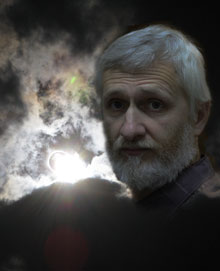Mechanics of Limitlessness

In this work, I present to readers a theoretical model of the formation of physical reality, based on the postulate of the existence of Limitlessness, that which has no limits and stands above all the root causes, since it includes them all. Random fluctuation in Limitlessness gives rise to the process of its multiple projections, going in growing cycles. The zero cycle divides Limitlessness into four identical parts – four dimensions. The eighth cycle in this sequence is our physical Universe, each of whose four dimensions consists of 2128 parts. This is the so-called determining integer of our Universe, its quantum characteristic - the number of state quanta that make up its full cycle. This is the only new and previously unknown to science number that is included in the formulas of this model. Nevertheless this number alone turned out to be enough to reduce to each other the formulas of all basic physical constants, the sizes of elementary particles, atoms and the Universe, to connect the mass of elementary particles with their sizes, to calculate the full cycle of the Universe and its part passed, to get the exact value of the decay time of a free neutron , the value of the mass of an electron relative to the mass of a neutron, to explain the nuclear forces, the phenomenon of inertia, the constancy of the speed of light and its independence from the motion of the frame of reference, and much more.© Any reprint or duplication only with the consent of the author. It is permitted to make copies for personal use.
﻿
 English Русский

Andrej PUZIKOV

Publication Date
of seventh edition
09.02.2023

Mechanics of Limitlessness
Seventh edition

Preface to the Seventh Edition

Content

As the theoretical research progresses, it becomes necessary to seriously correct the conclusions made earlier, to correct errors that are inevitable in such a new and unexplored direction of science, and to add new aspects of the research.

But it is precisely thanks to peripheral errors that the correctness of the main path is strengthened, when each error found does not lead to the destruction of the main building of logic, but, on the contrary, makes it more harmonious and opens up a number of new, previously unnoticed valuable discoveries.

In this seventh edition, the book has been completely revised. In addition to correcting some incorrect and sometimes erroneous logical constructions to explain the found correct formulas, the author set the task to make the reader's perception of the material as easy as possible. The presentation of the material, as far as possible, is adapted to the terminology accepted in modern physical science. The use of new terms has been reduced to the maximum, giving them a systematic, more easy-to-remember character. For the same purpose, some denotations of physical quantities have also been changed.

Foreword

Content

How did it all start?

According to the theories of modern physics based on experimental data, our Universe was born as a result of the "Big Bang". However, can the Big Bang be considered the beginning of everything? The very concept of an explosion implies the existence of conditions for this explosion, in this case, a superdense state of matter. However how did these conditions come about?

Physical science claims to reflect objective reality, while it itself was formed according to the laws of this reality and is its consequence. All the laws of physics were induced from physical experience, which, in turn, is based on the organs of perception of a person and his ability to form models in his internal representation that reflect the logic of the physical world.

Thus, in the process of its formation, physical science is constrained by the rigid limits of the possibilities of human representation, which, in turn, are shaped by the physical conditions of being. Those are these human representations that dictate the production of certain physical practice and experiments. It turns out an enclosed circle.

Mathematics allows you to expand the scope of this circle somewhat, to create models that go beyond the scope of the subject representation, for example: multidimensional spaces. However, in essence, this is just an illusion of going beyond the usual three-dimensional subject representations. The mathematical addition of one or more additional dimensions to three-dimensional space does not change the logic of this space, in other words, it does not go beyond the logic of human spatial representation. Even the introduction of imaginary numbers into formulas, in fact, is just a way to tie the explanation of physical processes to a two-dimensional number series - all the same habit of geometric representation in space, that can be curved, according to Einstein, however at the same time it remains a single space - some kind of original essence, a kind of "god", in which physicists firmly believe, and which was erected on the site of the once overthrown other "god" - ether.

The situation is even sadder with the concept of physical time. The representation of time in modern physics as a continuous, arbitrarily divisible spatial dimension, on the one hand, amuses violent human imagination, but on the other hand, leads science into a dead end of age-old habits of perception.

However, if we try to go beyond all these limits of human ideas and mathematical models? If we take into account a simple fact - any limits and restrictions are the result of some process that forms them. However the process of forming one or another framework itself occurs within the framework defined for it by some previous process.

The question is quite logical: did this process of the consistent formation of frames and restrictions, the consequence of which is our physical world, have a beginning?

The solution of this issue by the methods of modern physical science involves the induction of the root cause from the effect - our physical existence. Perhaps this path will eventually give the necessary answer. However, now, science cannot go further than some indefinite concept of superdense matter that gave rise to the Big Bang.

Nevertheless there is another way - not to induce a cause from an effect, but to assume the existence of the root cause that is not limited by any framework or limits, and on the basis of strict logic to build the theoretical model of what should become the consequence of this root cause.

In this work, I present to readers a theoretical model of the formation of physical reality, based on the postulate of the existence of Limitlessness, that which has no limits and stands above all the root causes, since it includes them all. Random fluctuation in Limitlessness gives rise to the process of its multiple projections, going in growing cycles. The zero cycle divides Limitlessness into four identical parts – four dimensions. The eighth cycle in this sequence is our physical Universe, each of whose four dimensions consists of 2128 parts. This is the so-called determining integer of our Universe, its quantum characteristic - the number of state quanta that make up its full cycle. This is the only new and previously unknown to science number that is included in the formulas of this model. Nevertheless this number alone turned out to be enough to reduce to each other the formulas of all basic physical constants, the sizes of elementary particles, atoms and the Universe, to connect the mass of elementary particles with their sizes, to calculate the full cycle of the Universe and its part passed, to get the exact value of the decay time of a free neutron , the value of the mass of an electron relative to the mass of a neutron, to explain the nuclear forces, the phenomenon of inertia, the constancy of the speed of light and its independence from the motion of the frame of reference, and much more.

The advantage of this model is not only that it provides an explanation for all physical phenomena, explains the dimensionality of space and time, but also reduces all physical laws to a single mechanical process of interaction of projections into the physical space of elementary localizations that are completely identical to the localization of the Universe. Full projections of elementary localizations are free neutrons, and partial projections are all other elementary particles.

In addition, the physical space itself is nothing more than a relative space of conditions in projection into the frame of reference associated with our planet. These or other conditions determine different spaces, which may be completely indefinite with respect to each other, or be bounding by general conditions.

Time, as a sequence of state quanta, holistic and indivisible acts of choosing reality, moving at the speed of light along one of the dimensions of space, instead of the principle of continuous flow of time accepted in traditional physics, is one of the main differences between this model and traditional ones.

-------------------

1. The Limitlessness

Content

We put the following postulate as the basis for further theoretical research:

Postulate:

There is something that has no restrictions and limits.

Let us call it Limitlessness:

Definition (d1):

The Limitlessness is that which has no restrictions.

Let us prove the main theorem of Limitlessness.

Theorem (t1):

Limitlessness, or what has no restrictions, is all-encompassing.

Proof:

Let us assume that there is something that is not covered by Limitlessness. In this case, a restriction is imposed on Limitlessness, expressed in the fact that Limitlessness does not cover this something. This contradicts the definition of Limitlessness. Consequently, the theorem can be considered proved.

The potential property of unlimited possibility of division into parts necessarily follows from the property of all-encompassing. Let us call this the principle of divisibility.

Definition (d2):

The principle of divisibility is the potential property of Limitlessness to be unlimitedly divided into parts.

In this connection, we prove the following theorems:

Theorem (t2):

Any part of Limitlessness is identical with the whole of Limitlessness.

Proof:

If in any part of Limitlessness there are some restrictions, it follows that Limitlessness itself is restricted by these restrictions in this part of itself, which contradicts the definition of Limitlessness. Therefore, parts of Limitlessness cannot have restrictions, and thus is completely identical to all Limitlessness in accordance with the definition. The theorem can be considered proved.

Conclusion (c1):

It follows from Theorem (t2) that all parts of Limitlessness are parts of any other part of it.

Theorem (t3):

The Limitlessness is symmetrical with respect to any parts of itself.

Proof:

If the Limitlessness is not symmetrical with respect to one of its parts, it will mean that Limitlessness is divided into two parts that are not identical to each other, which contradicts Theorem (t2). The theorem can be considered proved.

Conclusion (c2):

It follows from Theorem (t2) and Theorem (t3) that any part of Limitlessness is symmetric with respect to it and any other part of it.

2. localization

Content

As we have already noted in the first chapter, Limitlessness, as a comprehensive phenomenon, includes the principle of divisibility. However, the potential for division into parts does not mean the existence of some particular division.

On the other hand, one hundred percent probability of singling out a certain part in Limitlessness as a random fluctuation necessarily follows from the principle of the absence of restrictions.

This singling out a certain part in Limitlessness is a process that imposes limiting conditions on Limitlessness - the presence of the moment of the beginning of the process and its local position in Limitlessness. Since restrictions cannot be imposed on Limitlessness itself, these limiting conditions are reflected only in the relativity of the limiting process itself, as a frame of reference.

Conclusion (c3):

Any restriction in Limitlessness can exist only in the frame of reference associated with this restriction.

Random fluctuation is the first act of self-imposed limitation. From the condition of the absence of other conditions and restrictions, the complete absence of comparative properties, sizes and characteristics of a random fluctuation necessarily follows, except for the fact that it is localized in Limitlessness, and, accordingly, less than it. In all other respects fluctuation, as a part of Limitlessness, according to the theorem (r2) is completely identical to Limitlessness.

By its act of singling out, a random fluctuation in its own relativity divides Limitlessness into two identical parts: itself, as a material phenomenon, and Limitlessness, as a potential substance in which it has been singled out. But it is worth considering that the singled out part cannot go beyond its own limitation, or, in other words, reflect something that is beyond its own limitation. It follows from this condition that not only the singled out part itself, but also Limitlessness, as a complementary part, is projected relative to the singled out part in a limited variant.

This complex phenomenon of two identical parts of Limitlessness, limited by one limitation, acquires its objective existence.

Let us call it localization and give the following definitions:

Definition (d3):

Localization is a part of Limitlessness with a restricting condition imposed on it for the singling out of a part in its own internal relativity of this condition.

Definition (d4):

The material part of localization is the objectively singled out and localized part that forms the localization.

Definition (d5):

The potential part of localization is the projection of Limitlessness into the limiting conditions of localization.

We can consider processes in localization only in the frame of reference associated with its material part:

Definition (d6):

A local frame of reference is a frame of reference associated with the material part of localization.

Everything that, one way or another, is reflected in the local reference frame, is inside the localization, and nothing outside the localization can be reflected in the local reference frame. Thus, all objective processes within localization are determined by the imposed restriction and exist exclusively within this restriction.

Let us prove another important theorem.

Theorem (t4):

Each subsequent act of imposing restriction conditions in their own relativity of these conditions occurs in already existing conditions and cannot change them.

Proof:

Any restricting condition reduces the entire Limitlessness into conditions completely closed by this restriction. Thus, any new restricting condition imposed within the previous restricting condition is imposed on the Limitlessness in reduction to the previous condition in its closed restriction and cannot go beyond its restriction, that is, change it. The theorem can be considered proved.

Localization generated by a random fluctuation in Limitlessness, we will call primary.

The limiting condition of primary localization is the principle of division of Limitlessness into two parts, with the choice of one of them as a material manifestation, with which the local reference system is connected.

In accordance with the theorem (t2), according to the principle of identity with Infinity in the restriction by the condition of separation into two parts, the material and potential parts should be similarly divided into two parts inside themselves, fig. 1.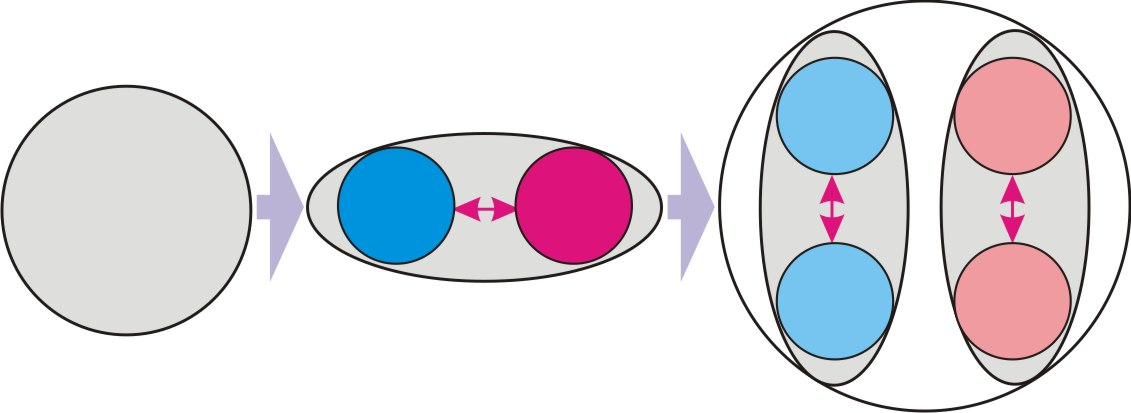Fig. 1.

Thus, the primary fluctuation in its internal relativity consists of four singled out parts. In accordance with the conclusion (c1) all these parts are simultaneously parts of each of them. We can call this property a projection into each other, fig. 2.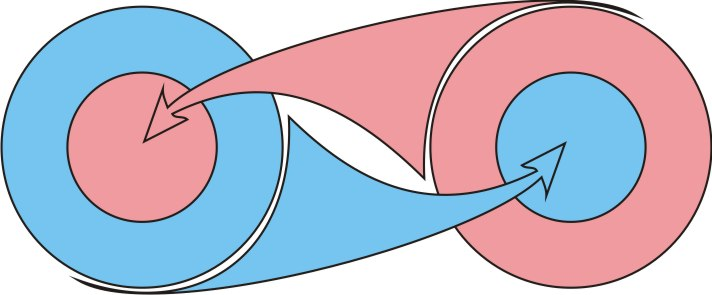Fig. 2.

Definition (d7):

The projection of one part of Limitlessness into another is the property of each part to include the other part as its own.

At first glance, it may seem that it should follow from the principle of the identity of parts that each new part of the divide process must in turn be divided into two parts, and this process of divide with the allocation of new parts will be endless. However, it is not. The divide of each of the parts into two identical secondary parts does not mean the addition of new parts. A similar secondary part of the one part of the primary divide is projected into similar secondary part of second part of the primary divide, fig. 3a).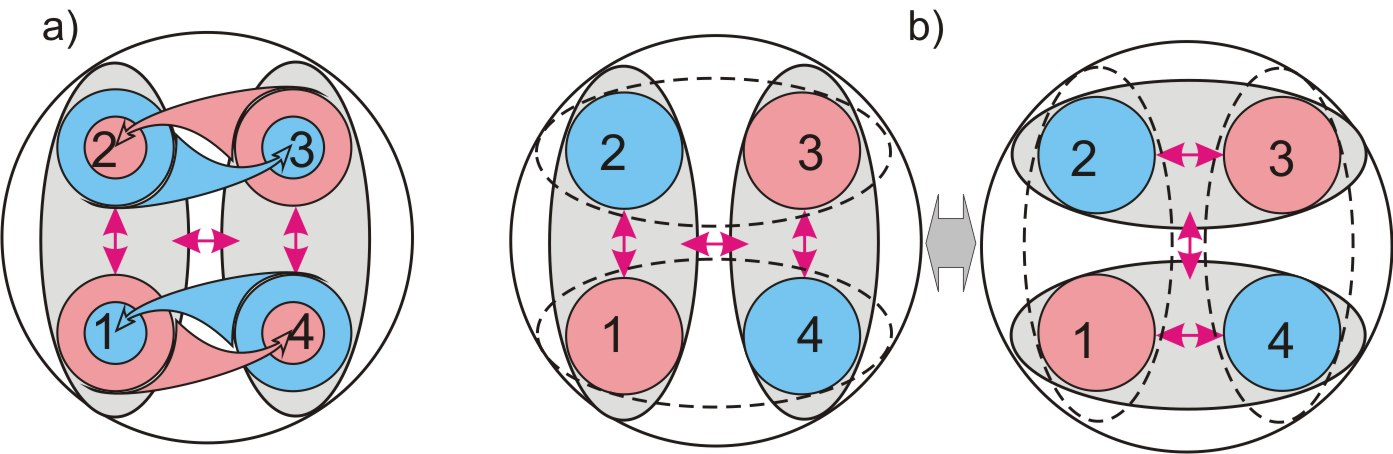Fig. 3.

This process of divide into two parts and mutual projection into each other is completely identical and symmetrical with respect to each of the four parts of the primary divide, fig. 3b). In this case, all other parts are projected into each of them.

Thus, the process of singling out parts by dividing each of the parts into two parts closes on itself and ends with the singled out four identical parts that make up the primary localization.

Conclusion (c4):

Random fluctuation, as the primary act of singling out a part in Limitlessness, causes the process of divide into two parts, which necessarily ends with the singling out of four identical parts in it in the relativity of the singled out part.

We give the following definitions:

Definition (d8):

The main parts of localization are the material part, which forms a local phenomenon and the potential part, which is Limitlessness in reduction to the limiting condition of the singled out part.

Definition (d9):

The secondary parts of localization are four identical parts, singled out by the projection of the process of division into two parts into both main parts.

3. The sequence of localizations

Content

It is correct to consider processes that occur outside the scope of localization only in a frame of reference taken out of its limiting conditions:

Definition (d10):

An external frame of reference is a frame of reference taken out of the bounding framework of localization.

This frame of reference, in some convention, can be considered a "look from the Limitlessness ".

In the external frame of reference, the entire primary localization is a localized material phenomenon. Thus, similarly to random fluctuations, the entire complex primary localization is the material part of the new localization. Let us define the primary localization as a zero-order localization, and the one formed on its basis as a first-order localization.

Let us pay attention to the fact that the local reference frame associated with the first-order localization is external with respect to the zero-order localization.

With respect to the local reference frame associated with the first-order localization, all four singled out parts of the zero-order localization do not differ from each other in any way and, on the basis of theorem (t2) and conclusion (c1), are completely identical.

This condition of preliminary division of the material part into four identical parts determines the process of formation of the first-order localization.

According to the identity principle (theorem (t2)) the potential part of the first-order localization should similarly consist of four singled out identical parts, fig. 4, a).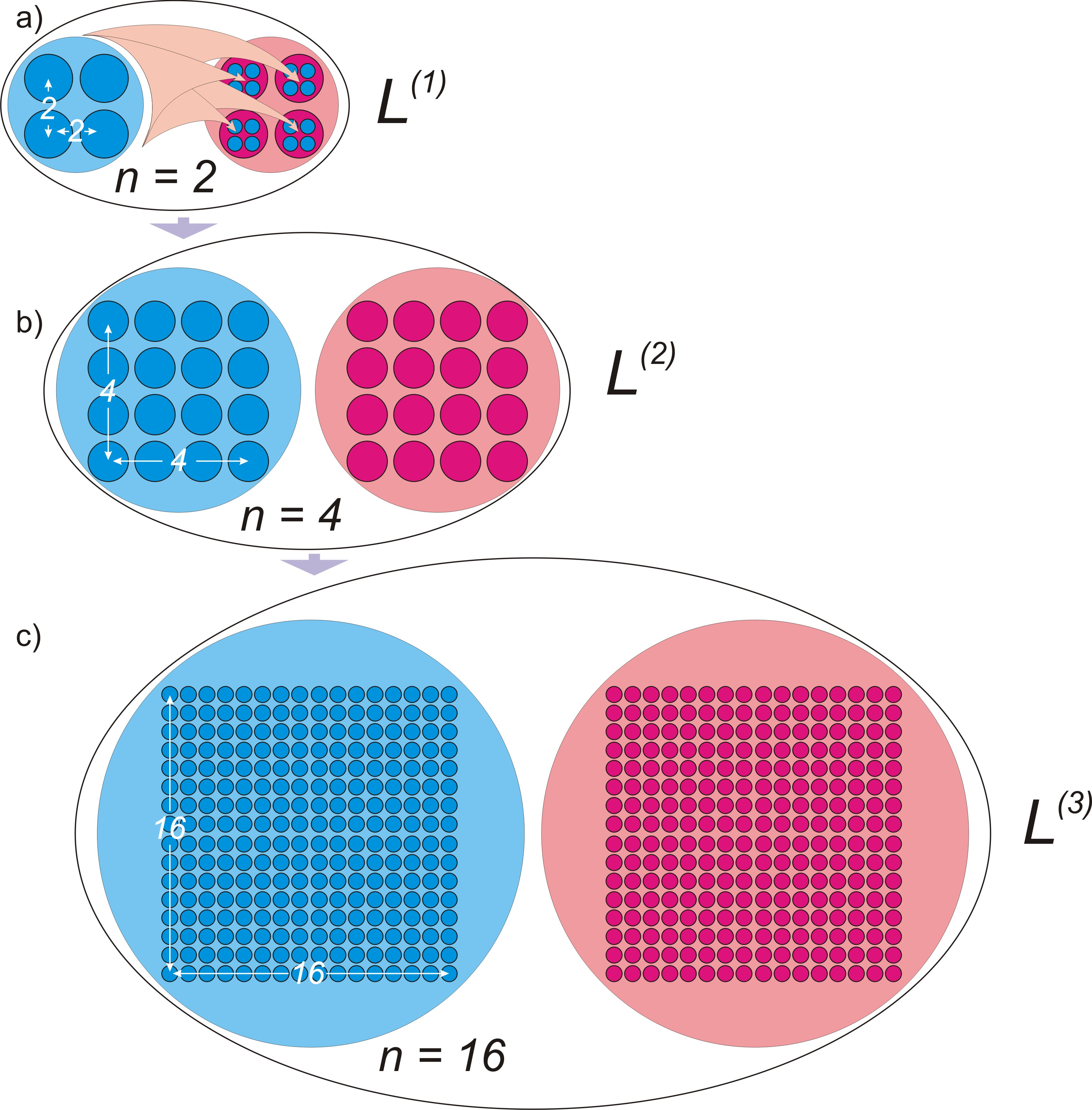Fig. 4.

And in accordance with the conclusion (c1), all parts of the material part must be projected into each of these parts of the potential part. And vice versa, all parts of the potential part must be projected into each of the parts of the material part. It follows from these conditions that the total number of identical parts in a first-order localization is equal to the square of the number of identical parts in each of its main parts – 42 = 16.

This first-order localization with respect to the external reference frame is similarly the material part of the second-order localization, consisting of 16 identical parts, fig. 4b). The total number of identical parts in the second-order localization will be equal to 162 = 256.

Thus, as a result of random fluctuation in Limitlessness, a sequence of localizations is formed with a rapidly growing number of parts of internal divide.

If we denote the number of parts in each of the four secondary parts of localization as n, then, accordingly, the number of parts in each main part will be equal to n2, and the total number of parts will be equal to n4.

Definition (d11):

The determining integer of localization is a positive integer n, equal to the number of identical parts into which each of the four secondary parts of localization is divided.

Conclusion (c5):

The material and potential parts of the localization with the determining integer n consist of n2 of their identical parts, and the total number of parts in the localization is n4.

In primary or zero order localization n = 1, in first order localization n = 2, in second order localization n = 4 in third order localization n = 16.

Conclusion (c6):

The determining integer of localization of the next order, starting from the localization of the first order, is equal to the square of the determining integer of localization of the previous order.

Thus, we can write down the formula for the determining integer of localization in the sequence of formation of localizations, starting from the localization of the first order:

n = 2(2k-1)  (01), where k is the order of localization.

Calculate the size of localizations of the first order of values k:

L(0):      n = 1

L(1):      n = 2

L(2):      n = 4

L(3):      n = 16

L(4):      n = 28 = 256

L(5):      n = 216 = 65536

L(6):      n = 232 = 4294967296

L(7):      n = 264 = 18446744073709551616

L(8):      n = 2128 = 3,40282366920938*1038

L(9):      n = 2256 = 1,15792089237316*1077

The logic of the process tells us that our physical Universe is a localization in Limitlessness and occupies a certain place in this sequence.

In the following chapters, on the basis of the complete coincidence of theoretical calculations with experimental physical data, we will prove that our Universe is an eighth-order localization with a determining integer n = 2128.

4. Dimensions of space

Content

In the local reference system, the material part is limited by the condition of local singled out and, as a result, does not coincide with the potential part. They are identical (theorem (t2)), but they do not coincide with each other. This principle of non-coincidence is identically projected into the secondary parts of localization.

Thus, all four secondary parts of localization do not coincide with each other. Herewith, they must be identical and, in accordance with the conclusion (c1), each of them must include the other three with all their parts. At first glance, these conditions seem to contradict each other. But it is the presence of all these conditions that determines what we are accustomed to perceive as physical space. Let us consider it on the example of two-dimensional space.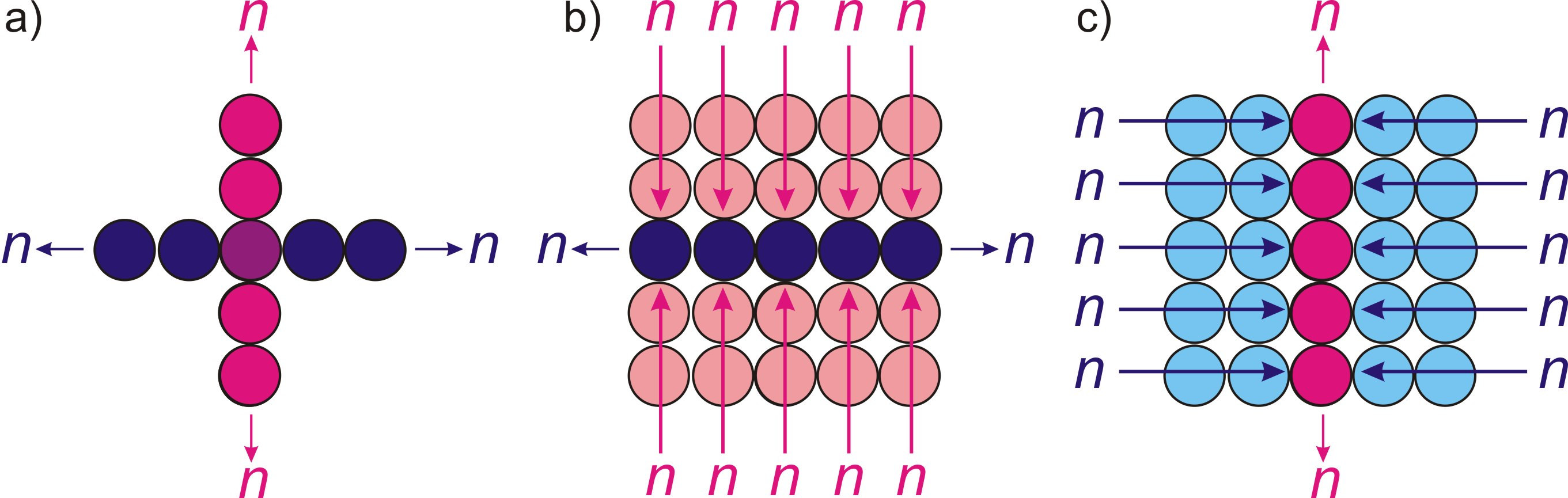Fig. 5.

On fig. 5, a) shows two completely identical and equal parts that do not coincide with each other. Herewith, each of them meets the condition of symmetry with respect to the other.

In accordance with the conclusion (c1) , all parts of one of these two parts are projected (definition (d7)) into each of the parts of the other part, fig. 5, b) and c).

In the case of localization, there are four such, projecting into each other and non-coinciding parts. Two of them are parts of the material part, the other two are parts of the potential parts. But, proceeding from the same conclusion (c1), all of them must be projecting both into the material and potential part.

Thus, localization in its internal structure in the relativity of the local reference system is four-dimensional, and the number of parts in each of its four dimensions is equal to its determining integer n.

This four-dimensional structure consists of two two-dimensional structures, which are the two main parts of the localization.

Conclusion (c7):

The internal space of localization is four-dimensional and is determined by two two-dimensional main parts of localization projected into each other.

Conclusion (c8):

The number of parts in each of the four localization dimensions is equal to the determining integer of localization n.

The process of forming the four-dimensional structure of the internal space of each localization is initiated by the initial act of singling out its material part. Another important conclusion follows from this:

Conclusion (c9):

The four-dimensional localization structure of each order is formed exclusively in the local frame of reference associated with it and does not depend on the similar localization structure of the previous order.

5. Symmetry restoration cycle

Content

In the local reference frame, the material part is not symmetrical with respect to the potential part. Thus, as a result of the act of singling out the material part, there is a disturbance of symmetry in localization. As a consequence, in the absence of other restrictions, except for the conditions that formed the localization, the act of singling out the material part must be repeated in its potential part, and the number of these repetitions depends on the determining integer of localization, until the possibilities of the potential part are completely exhausted.

Conclusion (c10):

The act of singling out the material part is necessarily repeated in the potential part of the localization.

Thus, we can define the entire cycle of repetitions of the material part of localization as a cycle of restoration of symmetry.

Definition (d12):

The symmetry restoration cycle is a complete cycle of repetitions of the act of singling out the material part in localization, until the entire two-dimensional space of its potential part is filled.

In the relativity of the external reference system, the entire full cycle of localization is one holistic phenomenon in which the position of the material part is indefinite, that is, it is a superposition of all alternative versions of its position. Moreover, this superposition uniformly fills the entire limited localization space. Thus, with respect to the external frame of reference, localization is a completely internally symmetrical phenomenon with respect to all its parts.

Conclusion (c11):

Relative to the external reference frame, localization is a superposition of all alternative positions of its parts and is completely symmetrical with respect to each of them.

With respect to the local reference system, the sequence of acts of separating the material part along the symmetry restoration cycle is a one-dimensional process.

Since the potential part consists of two identical parts - dimensions, the local reference system must be associated with one of the dimensions of the two-dimensional structure of the potential part, and the initial act of singling out must determine the moment of counting on it.

The absence of restrictions on the choice of the direction of the process movement, as well as the principle of symmetry (theorem (t3)) determine the movement of the process symmetrically in both directions along the chosen dimension.

Definition (d13):

The definite dimension is a dimension limited by the condition of choosing the moment of counting of the symmetry restoration cycle.

This limiting condition deprives the definite dimension of the property of full internal symmetry with respect to its parts, which is reflected in the symmetry restoration cycle.

Thus, the definite dimension, as an identical part of Limitlessness, is limited by three conditions: the determining integer of localization n, two directions of the cycle movement along it, and the presence of the moment of counting. An important conclusion follows from this:

Conclusion (c12):

The process of repetition of the acts of singling out the material part along the definite dimension sets the primary measure of extension, which is identically projected into all parts and processes within the localization, determining their commensurability.

The second dimension of the two-dimensional structure of the potential part is identical to the defined one, except for one limiting condition - the presence of the moment of counting. Let us call it indefinite.

Definition (d14):

The indefinite dimension is a dimension onto which the moment of counting of the symmetry restoration cycle is not projected.

From the lack of definiteness of the moment of counting of the cycle along the indefinite dimension, the conclusion follows:

Conclusion (c13):

The initial moment of the symmetry restoration cycle with respect to the indefinite dimension is in the superposition of all its alternatives positions on it.

The one-dimensional process of the sequence of acts of singling out the material part must proceed symmetrically throughout the entire material part. From this follows the conclusion:

Conclusion (c14):

The two-dimensional structure of the potential part is formed by the superposition of all alternative directions of its definite dimension from the initial act of singling out the material part.

In each of the variants of this superposition, the process proceeds symmetrically in opposite directions along a definite dimension, Fig. 6.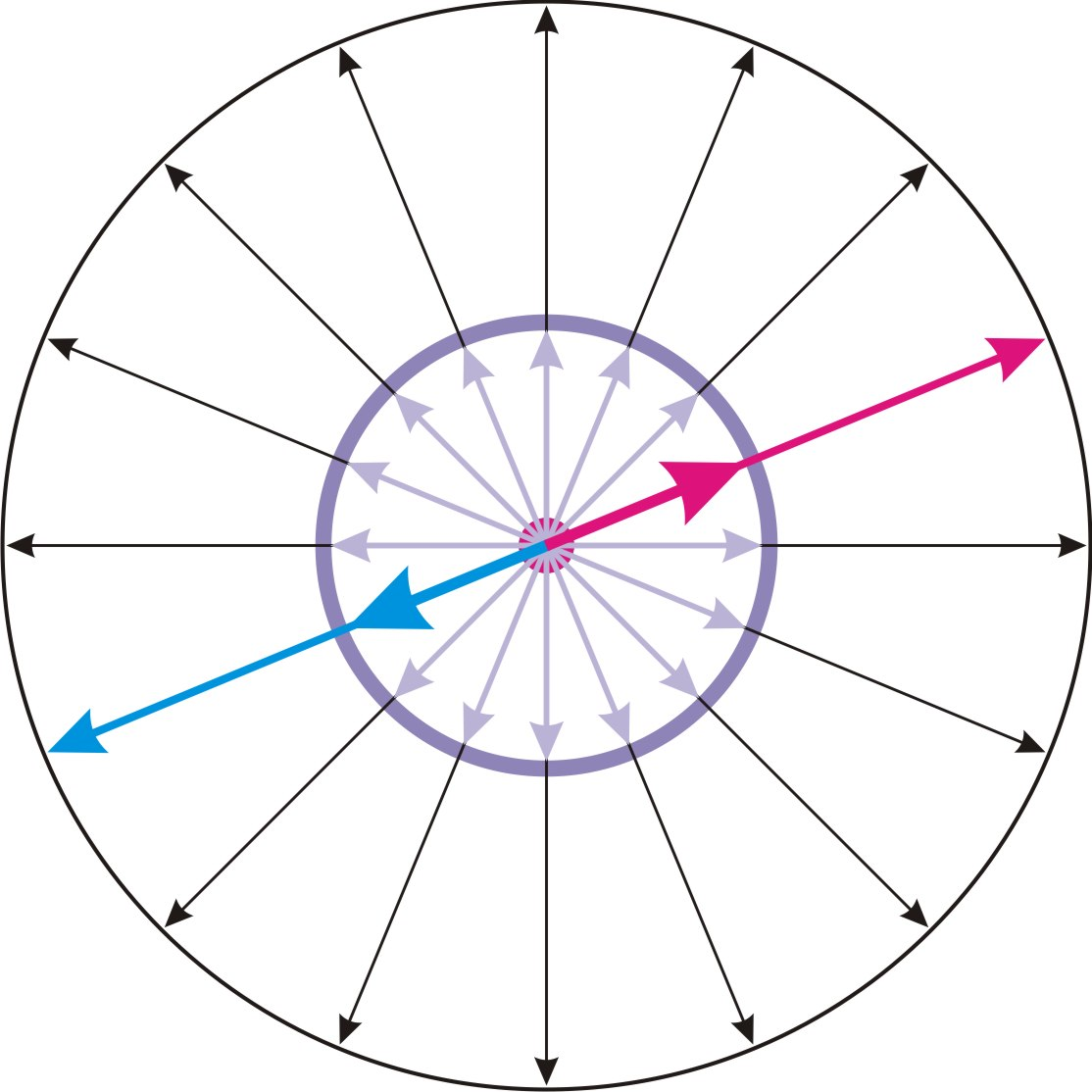Fig. 6.

The presence of two opposite directions in each of the dimensions is a projection in them of the principle of primary division into two parts.

The limiting condition imposed on a definite dimension must necessarily be reflecting in its size.

As a result, the defined dimension must be shorter in size than the second indefinite dimension of the potential part.

Regarding the entire process of restoration of symmetry, we conclude:

Conclusion (c15):

The process of repetition of the material part occurs according to a definite dimension of the two-dimensional structure of the potential part, with which the reference system is connected, symmetrically in all directions and is an expanding circle, in the center of which is the primary act of singling out of the material part.

Conclusion (c16):

The two-dimensional structure of the potential part is a circle, the diameter of which is equal to the full size of the definite dimension, and the circumference is equal to the full size of the indefinite dimension.

The ratio of the full size of the indefinite dimension, as the circumference of a circle, to the full size of the definite one, which is its diameter, determines the numerical value of the number π.

Conclusion (c17):

The reduction of the size of a definite dimension in the associated with him frame of reference relative to an indefinite dimension determines the number π.

From the condition of the identity of both dimensions of the potential part, the principle of relativity of the reference frame binding to one of them follows, Fig. 7.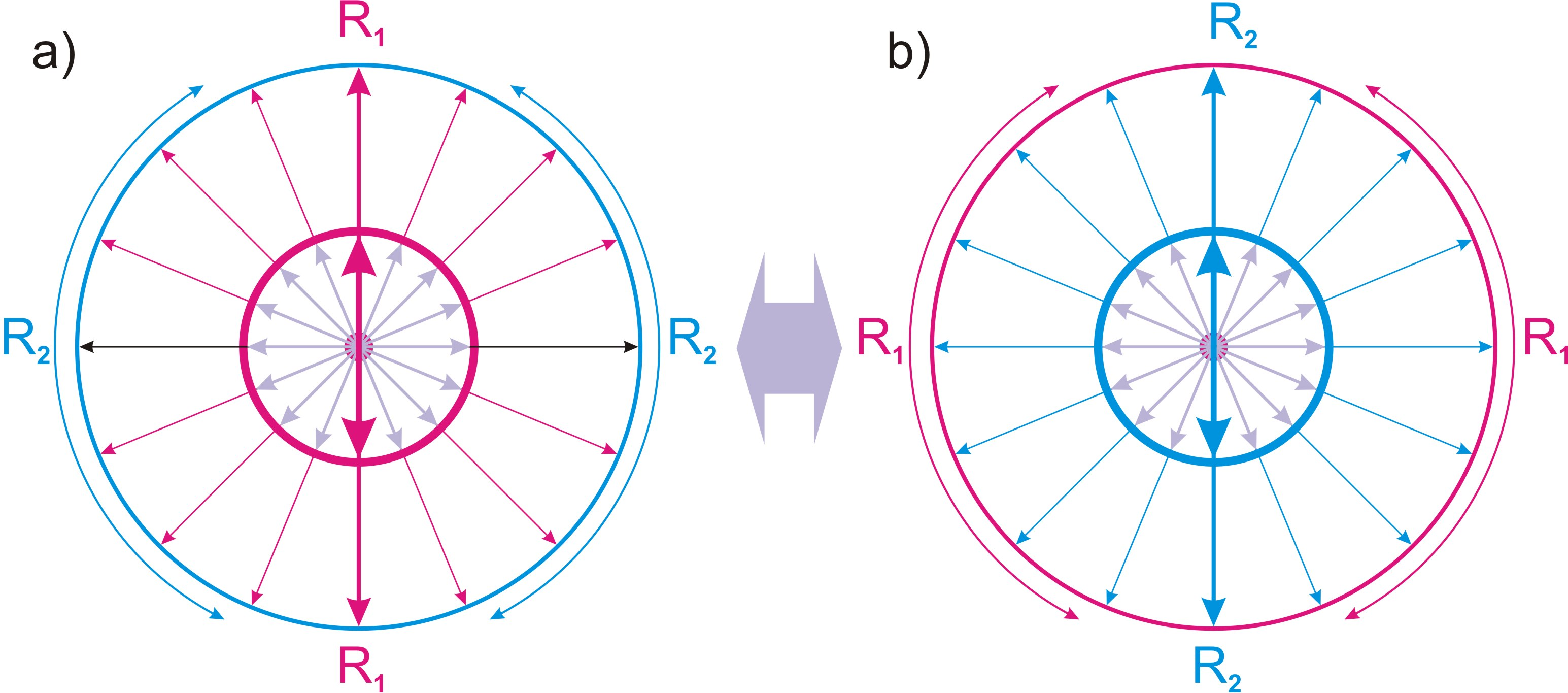Fig. 7.

On fig. 7, a) the local reference system is associated with dimension R1, and in fig. 7, b) with dimension R2.

Conclusion (c18):

The cycle of symmetry restoration inside localization does not depend on the choice of the reference frame associated with one of the two dimensions of its potential part, and proceeds in the same way in each of them.

The two-dimensional structure of the potential part with the condition of the definiteness of its center is reflected in each part of the two-dimensional structure of the material part, from which the conclusions must follow:

Conclusion (c19):

The internal space of localization is a four-dimensional ball, as a four-dimensional superposition of all alternative positions of the two-dimensional structure of its potential part.

Conclusion (c20):

The definite dimension of the potential part is the diameter of the internal localization space with two opposite directions of movement along it of the symmetry restoration cycle.

As for the two dimensions of the material part of localization, in the local reference frame they are not determined by any other conditions, except for the condition of the identity of the two main parts, they are secondary parts of which. Accordingly, the two dimensions of the material part must project the mutual correspondence of the two dimensions of the potential part.

Conclusion (c21):

In the local frame of reference, the material part of the localization is identical to the potential part, and is formed by definite and indefinite dimensions.

6. Time

Content

The local reference system is connected with the material part and is identically repeated in each subsequent act of its singling out. Thus, we can talk about a single local frame of reference for the entire internal cycle of localization, which is a limited sequence of states of the material part.

This sequence of states of the material part is what we perceive as the passage of time. The rapid change of states in comparison with the possibilities of registering this process with the human senses creates the illusion of a continuous flow of time. This concept is firmly rooted in physical science, despite the obvious problems of its use in quantum mechanics.

Time is discrete and consists of separate states. The sequence of these states determines the sequence of causes and effects.

Definition (d15):

A quantum of state is every act of isolation of the material part of localization in its cycle of symmetry restoration.

Conclusion (c22):

Time is a sequence of quanta of state.

For each quantum of state separately, the concept of the flow of time is inapplicable, and internal processes are uncertain and represent a superposition of alternative options and sequences. This conclusion follows from the fact that there are no other restrictions imposed on the material part, except for the condition of its local singling out.

Definition (d16):

One-moment processes are processes occurring inside the quantum of state.

Conclusion (c23):

Each quantum of state in the sequence of time is a superposition of alternative sequences of its internal processes.

The number of alternative options in each particular case is determined by the conditions, which have a place in this case.

Based on the fact that all the dimensional characteristics of the internal processes of localization are determined by the movement of the material part along the definite dimension, we will call it the mainstay dimension.

Definition (d17):

The mainstay dimension is the definite dimension of the two-dimensional structure of the potential part of localization, along which the sequence of quanta of state of the material part or time is realized.

Let us define a projection of a quantum of state onto the mainstay dimension as a quantum of extension.

Definition (d18):

The quantum of extension is the projection of the quantum of state on the mainstay dimension.

Let us denote the size of the quantum of extension in units of length as dr, and in units of time as dt.

The number of quanta of state in the sequence of time is equal to the number of quanta of extension along the mainstay dimension, which in turn is equal to the number of parts allocated in it. Thus, in accordance with definition (d11), the number of quanta of state in the full cycle of localization is equal to its determining integer n.

In the local reference system, the successive process of changing quanta of state of the material part is the expanding three-dimensional surface of the four-dimensional sphere, the diameter of which is the mainstay dimension. Further, we will use the symbol Tuto denote the mainstay dimension, and the symbol Td for the second indefinite dimension of the potential part.

Conclusion (c24):

The superposition of alternative positions of the mainstay dimension Tu determines the diameter of a four-dimensional sphere, the three-dimensional surface of which is a sequence of states of the material part of the localization.

This sequence of states, in turn, is a three-dimensional superposition of a two-dimensional circumference as a projection of the material part onto the two-dimensional structure TuTd of the potential part, the thickness of which is determined by the size of quantum of state dr along the mainstay dimension Tu, fig. 8.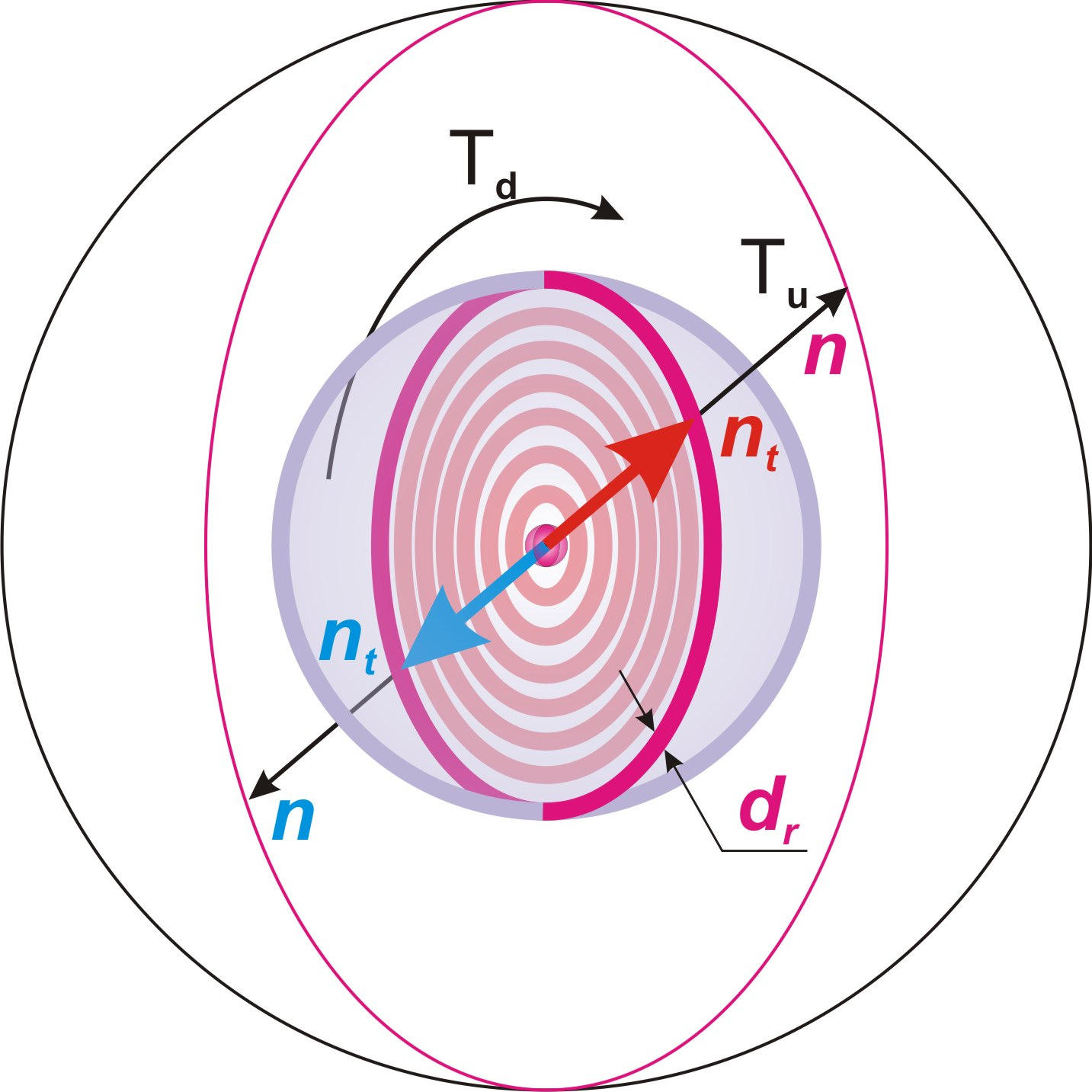Fig. 8.

The realization of a sequence of quanta of state along one of the four dimensions of localization does not mean that this dimension is identical to the process of time. These are two different physical phenomena. Time, as a sequence of states, is always directed in one direction and cannot be turned back. In turn, the mainstay dimension Tu, along which the sequence of time is realized, is identical to other dimensions and has two directions.

The speed of displacement of the material part in state quanta along the mainstay dimension is equal to dr/dt .

None of the parts of the material part can outstrip its movement in the inner space of localization. This gives us reason to denote this maximum speed in the same way as the speed of light is traditionally denoted in physics - the letter c.

In the following chapters, we will prove that light travels in vacuum at precisely this speed.

c = dr/dt (02)

Conclusion (c25):

The speed c = dr/dt яis the maximum possible speed of movement in the inner space of localization.

In the localization of the next order, the entire cycle of the previous localization becomes the first quantum of state. Thus, the size of state quantum of the next order localization in units of length is ndr, and in units of time ndt. Accordingly, the speed of movement through the cycle will be equal to:

ndr/ndt = dr/dt = c =const (03)

Conclusion (c26):

The speed of displacement of the material part along the localization cycle does not depend on its order and is a universal constant.

Since the sequence of time consists of n state quanta, we can write:

Tn = ndt (04), where Tn - time of the full cycle of localization.

Rn = ndr (05), where Rn - radius of the four-dimensional inner space of localization.

Rt = ntdr = Rnnt/n (06), where Rt – radius of the four-dimensional sphere whose three-dimensional surface is the material part of the localization, nt - the number of state quanta passed by the main localization cycle.

In the first act of the sequence of time, the material part was compressed to the size n times smaller than the size of this material part in the last act. Thus, the process of expansion with the maximum possible speed c is nothing else than the "Big Bang", the conclusion about which is pushed by practical measurements and observations of our Universe, Fig. 9.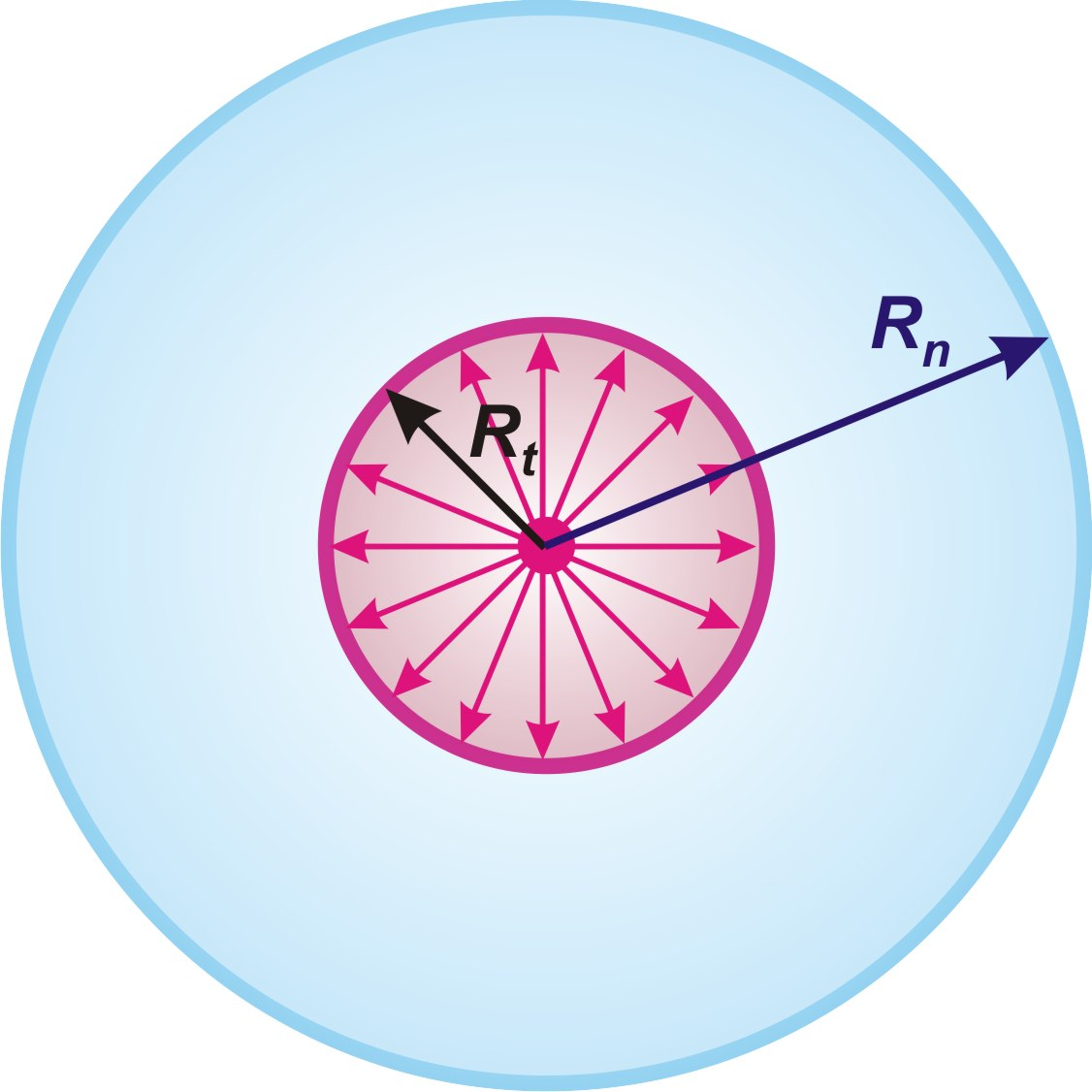Fig. 9.

Conclusion (c27):

The "Big Bang", as the beginning of the existence of our Universe, is the beginning of a sequence of state quanta along the cycle of symmetry restoration in its localization.

Thus, the "Big Bang" is not only the first moments of the existence of our Universe, but its state throughout the cycle. As its size increases at a maximum speed of c, the relative size changes in a small local area, such as our immediate space, become almost imperceptible compared to the historical period of scientific observations.

But what will happen to the material Universe after the completion of its complete cycle

The internal processes of localization during the entire cycle are completely limited by the inner surface of the four-dimensional sphere that closes the localization, and relative to the local frame of reference, nothing exists outside this surface.

Upon completion of the full cycle, the local reference frame associated with the material part passes into the localization of the next order, as a local reference frame associated with the entire localization that has completed its cycle, which in the new localization is the first act of singling out of its material part. In other words, all n quanta of state of the previous localization in the new localization represent one one-moment state. Thus, the local reference frame of a new localization of the next order is a superposition of alternative sequences of quanta of state of the previous localization, as initial one-moment state of itself.

This means that the process of expansion of a four-dimensional sphere generated by a random fluctuation continues indefinitely through a sequence of localization stages, each of which has its own time.

Conclusion (c28):

The entire cycle of localization of the previous order becomes the initial one-moment state of the localization of the next order.

7. Spot frame of reference and physical space

Content

In accordance with the conclusion (c5) , the material part of the localization with the determining integer n consists of n2 of its identical parts.

All parts of the material part have not been singled out in the local reference frame. Thus, the material part is a superposition of all possible alternative positions of its parts.

The process of random fluctuation, as localization in Infinity, must be identically reflected in all its parts. Accordingly, the process of random selection and localization of parts must also take place inside the material part, creating new frames of reference. For the appearance of an internal reference system, it is sufficient to localize one part, which distinguishes it from others. At the same time, according to the principle of identity, all other identical parts of the internal division are automatically singling out and localized.

Definition (d19):

Spot frame of reference is the frame of reference associated with the localized part within the material part of the localization.

The expanding sphere of superposition of alternative positions of the material part moves along the mainstay dimension Tu in all directions, but each point on this sphere moves strictly in one direction, fig. 10, a).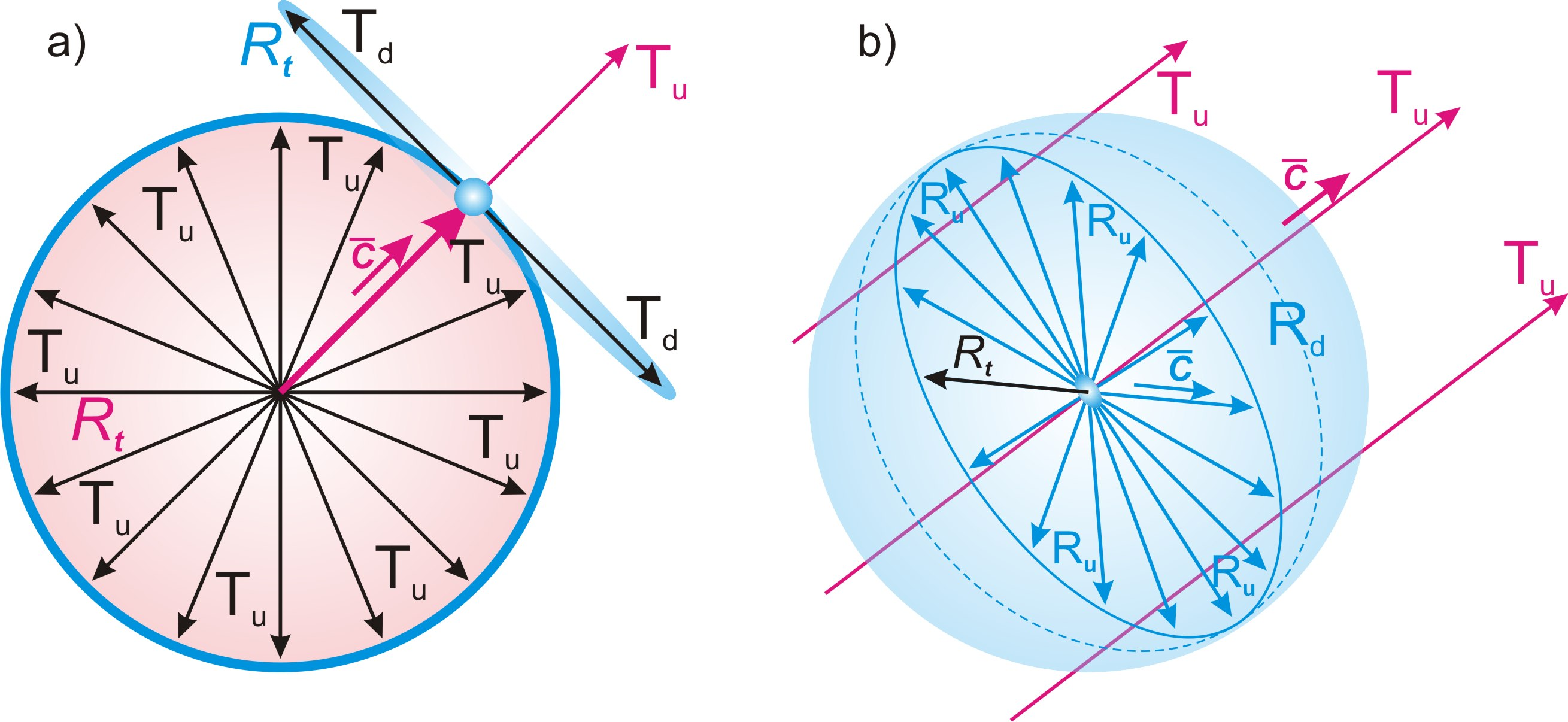Fig. 10.

Conclusion (c29):

In the local frame of reference, the mainstay dimension is projected by a unidirectional vector with respect to each spot frame of reference.

Since the spot reference frame, including all of its potential parts of the internal division, moves along the mainstay dimension with the same speed c, this dimension is not projected into it. No part of the material part in the spot reference system can be displaced relative to another part along this dimension, or have quantitative or qualitative relationships along it, except for the principle of complete identity.

Conclusion (c30):

The mainstay dimension is not projected into a spot frame of reference.

This conclusion necessarily leads to the following important conclusions:

Conclusion (c31):

Time, as a sequence of state quanta of the material part of the localization, in the relativity of the spot reference frame has no spatial properties.

Conclusion (c32):

The space in the relativity of a spot frame of reference is three-dimensional and is formed by the projection of an indefinite dimension Td of the potential part of the localization and two dimensions of its material part.

This is the space that we are accustomed to perceive as physical.

In the future, for convenience, we will call a definite dimension of the material part - a definite dimension of the physical space and denote Ru, and an indefinite one - an indefinite dimension of the physical space and denote Rd.

According to the principle of symmetry, the entire material part of the localization should be projected symmetrically with respect to each of its singled out parts, Fig. 10, b).

Conclusion (c33):

Each spot frame of reference in its own relativity is in the center of physical space.

All sizes in relation to any frame of reference are determined by the projection of the size of its own quantum of extension. From this follows the conclusion:

Conclusion (c34):

All relative combinations of sizes of localization parts are projected with respect to any spot frame of reference in the same way.

Conclusion (c35):

Physical space is relative and depends on the choice of spot frame of reference.

According to the principle of identity of the two main parts of localization (theorem (t2))and the internal symmetry of the material part (conclusion (c2)), the size of the material part along its definite dimension Ru is equal to the radius Rt of its expanding four-dimensional sphere along the mainstay dimension Tu in the quantum of state nt. Considering the two-dimensionality of the material part, we come to important conclusions:

Conclusion (c36):

Relative to each spot frame of reference, the material part of the localization is projected by a three-dimensional superposition of alternative positions of its two-dimensional structure RuRd, which is a flat circle, in the center of which there is a frame of reference.

Conclusion (c37):

The physical space is projected relative to each spot frame of reference by a three-dimensional ball, in the center of which this frame of reference is located.

Conclusion (c38):

The radius of the physical space Rt is determined by the size of a definite dimension Ru of the material part of the localization, which is equal to the product of the number of passed quanta of state nt by the quantum of extension dr.

Thus, the radius of the physical space is determined by formula (06):

Rt = ntdr = Rnnt/n

Conclusion (c39):

The radius of the physical space Rt increases along the sequence of quanta of state with the maximum possible speed c.

It follows from the principle of symmetry of the physical space (conclusion (c2)) that the size of the projection of the indefinite dimension Td of the potential part of the localization into the physical space is equal to the size of its indefinite dimension Rd. In accordance with the conclusions (c17) and (в38)), the total size of the indefinite dimension Rd is equal to πRt.

Conclusion (c40):

The size of the projection of the indefinite dimension Td of the potential part of the localization into the physical space is dynamic and in the quantum of state is equal to πRt.

8. Elementary localizations as fundamental particles of matter

Content

The process of localization of a part within the material part is identical to the process of localization of the material part itself. It follows from this that each of the n2 parts of the material part forms its own localization, identical to the main one. For convenience, we give the following definitions:

Definition (d20):

The main localization is each localization in the sequence of localizations generated by a random fluctuation.

Definition (d21):

Elementary localization is the localization of each of the n2 identical parts of the material part of the main localization with the determining integer n.

According to the principle of identity of parts and whole (theorem (t2)) elementary localizations are identical parts of the main localization.

Conclusion (c41):

Each main localization with determining integer n consists of n2 identical elementary localizations.

Conclusion (c42):

The determining integer n of the main localization is equal to the determining integer of each of its elementary localizations.

The projections of elementary localizations into the physical space are the fundamental particles of matter.

Definition (d22):

Fundamental particles are projections of elementary localizations into physical space.

All four dimensions of the internal space of elementary localization are not connected with the conditions that determine the mutual direction of the dimensions of the main localization and are indefinite with respect to them. This condition of indefiniteness is projected into physical space. According to the conclusion (c19):

Conclusion (c43):

The inner space of a fundamental particle is a four-dimensional ball with an indefinite inner space and an indefinite center relative to the dimensions of the physical space.

According to the principle of identity with the main localization, elementary localization should consist of material and potential parts.

Let us denote: the definite dimension of the potential part of the elementary localization as tu, and the indefinite one as td; a definite dimension of its material part as ru, and an indefinite one as rd.

The material part of elementary localization must be projected into the physical space by a material object. From the condition of indefiniteness, in accordance with the conclusion (c11), it follows that this material object is in a superposition of all its possible alternative positions inside the fundamental particle.

Definition (d23):

The physical body of a fundamental particle is the projection of the material part of the elementary localization into the physical space.

Conclusion (c44):

A fundamental particle is a three-dimensional superposition in physical space of all possible alternative positions, of its physical body.

The conditions of the projection of the physical body of a fundamental particle into the physical space are identical to the conditions of the projection of the entire particle.

In this connection, the conditions of the physical space are superimposed on the conditions of the internal space of the fundamental particle.

From this conclusion, together with the conclusion (c43), the following conclusions follow:

Conclusion (c45):

The physical body of a fundamental particle is a four-dimensional ball in physical space with a completely indefinite inner space and its center, which is simultaneously in the conditions of the projection of the inner space of elementary localization and in the conditions of physical space.

Conclusion (c46):

The physical body of a fundamental particle is in the physical space a three-dimensional superposition of all possible alternative positions of its center.

The size of the fundamental particle along the mainstay dimension Tu, , as well as the size of the entire material part along it, is equal to the quantum of extension dr.

From the principle of division into n parts (conclusions (c8),(в42))по each of the two dimensions of the material part, and the conclusion (c38), the conclusion follows:

Conclusion (c47):

The size of a fundamental particle in physical space is determined by its size along the definite dimension of physical space Ru, which is n times smaller than its total size Rt.

Taking into account formula (06) and conclusion (c38):

rt = Rt/n = drnt/n (07) , where rt — the size of the elementary localization along the definite dimension Ru of the material part of the main localization in the quantum of state nt.

Taking into account the uncertainty of the position of the physical body inside the fundamental particle (conclusion (c46)) let us call the value rt as the size of presence the fundamental particle.

Definition (d24):

The size of the presence of a fundamental particle rt is the diameter of a spherical region, which is a superposition of all possible alternative positions of its physical body.

On the other hand, from the identity condition of the elementary localizations of the main localization, it follows that the size of a definite dimension tu of the potential part of the elementary localization must be n times smaller than the analogous size Tu of the main localization. That is, it must be equal to the same size rt.

A certain dimension tu of the potential part of the elementary localization is the radius of the inner four-dimensional space of the fundamental particle. Herewith, the size of presence of a fundamental particle rt is its diameter in physical space.

It turns out that the radius of the inner space of a fundamental particle is equal to its diameter in the outer physical space. There is no contradiction in this conclusion.

In accordance with the conclusion (c45), the internal four-dimensional space of a fundamental particle is indefinite with respect to the physical space. The only condition that determines the dimensional relations of these spaces is the maximum possible distance between the alternative positions of the physical body in their superposition inside the fundamental particle.

Thus, the four-dimensional ball of the internal space of a fundamental particle of radius rt, determined by the total size of a definite dimension tu of the potential part of the elementary localization, fig. 12, a) is projected into the physical space along its definite dimension Ru by the maximum possible distance between the positions of the physical body inside the fundamental particle. To this it is necessary to add the condition of uncertainty of the center of the physical body (conclusion (c46)), fig. 12, b).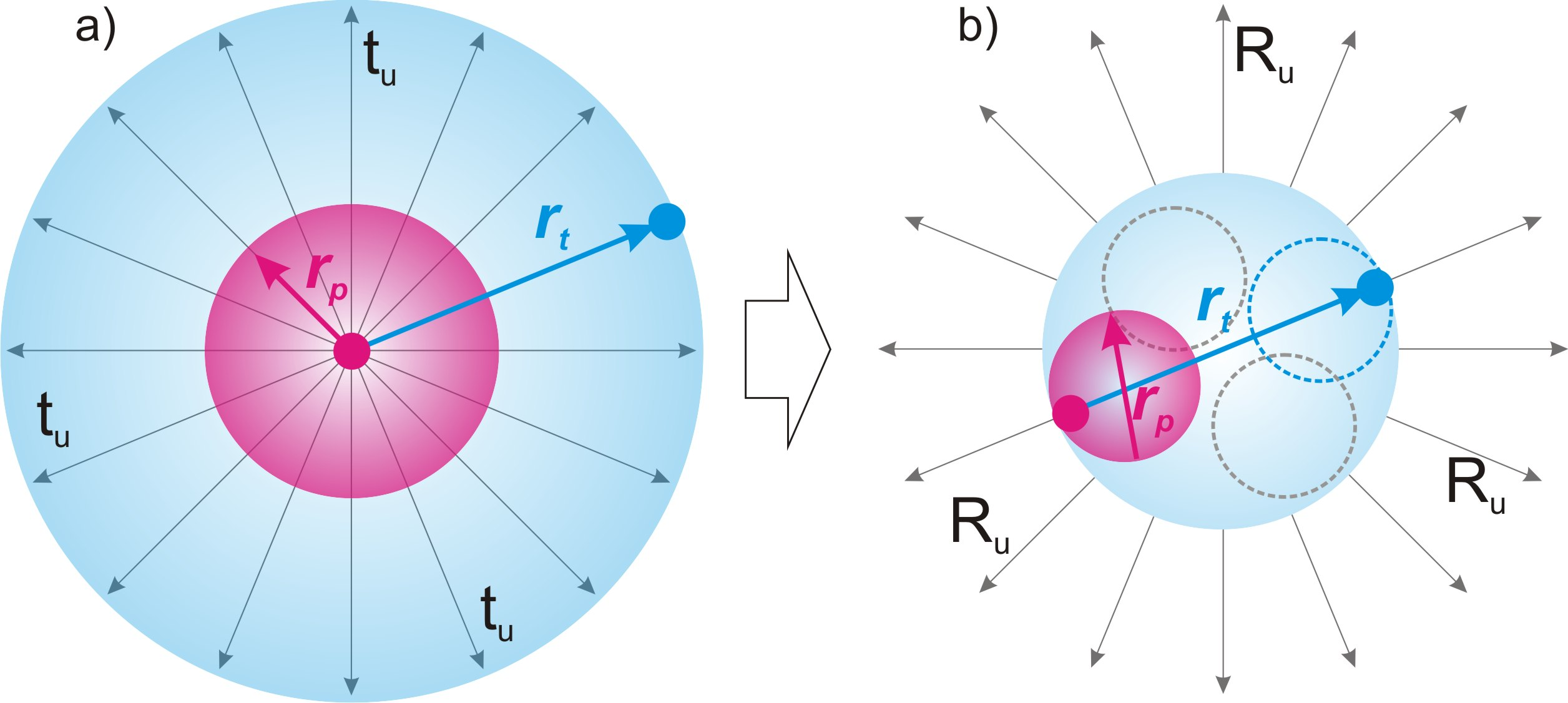Fig. 12.

Conclusion (c48):

The radius of the inner space of the elementary localization rt according to a definite dimension rt according to a definite dimension tu of the potential part of the elementary localization is projected into the physical space by the diameter of the fundamental particle according to a definite dimension of the physical space Ru.

Conclusion (c49):

The radius of the inner space of the material part of the elementary localization rp is projected into the physical space as the diameter of the physical body of the fundamental particle.

According to the principle of identity with the main localization, the ratio of the radius of the inner space of the physical body of the fundamental particle rp to the radius of its inner space rtshould be equal to the similar ratio of the radius of the physical space Rt to the radius of the inner space Rn of the main localization.

rp/rt = Rt/Rn = nt/n (08), where rp — the radius of the internal space of a physical body in the quantum of state nt.

Taking into account formula (07), we can write:

rp = rtnt/n = nt2dr/n2 (09)

The physical body of a fundamental particle in each of the alternative positions within its size of presence is a material manifestation of the fundamental particle. Thus, we can call the size rp the physical size.

Definition (d25):

The physical size фof a fundamental particle is the diameter of its physical body rp along the definite dimension Ru of the physical space.

The completion of the cycle of elementary localization occurs together with the completion of the cycle of main localization in the last quantum of the state of main localization at nt = n, when its size rt becomes equal to dr.

Conclusion (c50):

The size of a complete cycle of elementary localization is equal to the quantum of extension dr.

9. Spin of a fundamental particle

Content

In accordance with the conclusion (c15) and the conclusion (c24), the cycle of restoration of the symmetry of the main localization moves simultaneously in two opposite directions along the mainstay dimension Tu. This movement should be identically reflected in the second indefinite dimension of the potential part Td. It follows from the uncertainty condition of this dimension that this movement is a uniform expansion of the material part along it simultaneously in two directions, Fig. 13, a).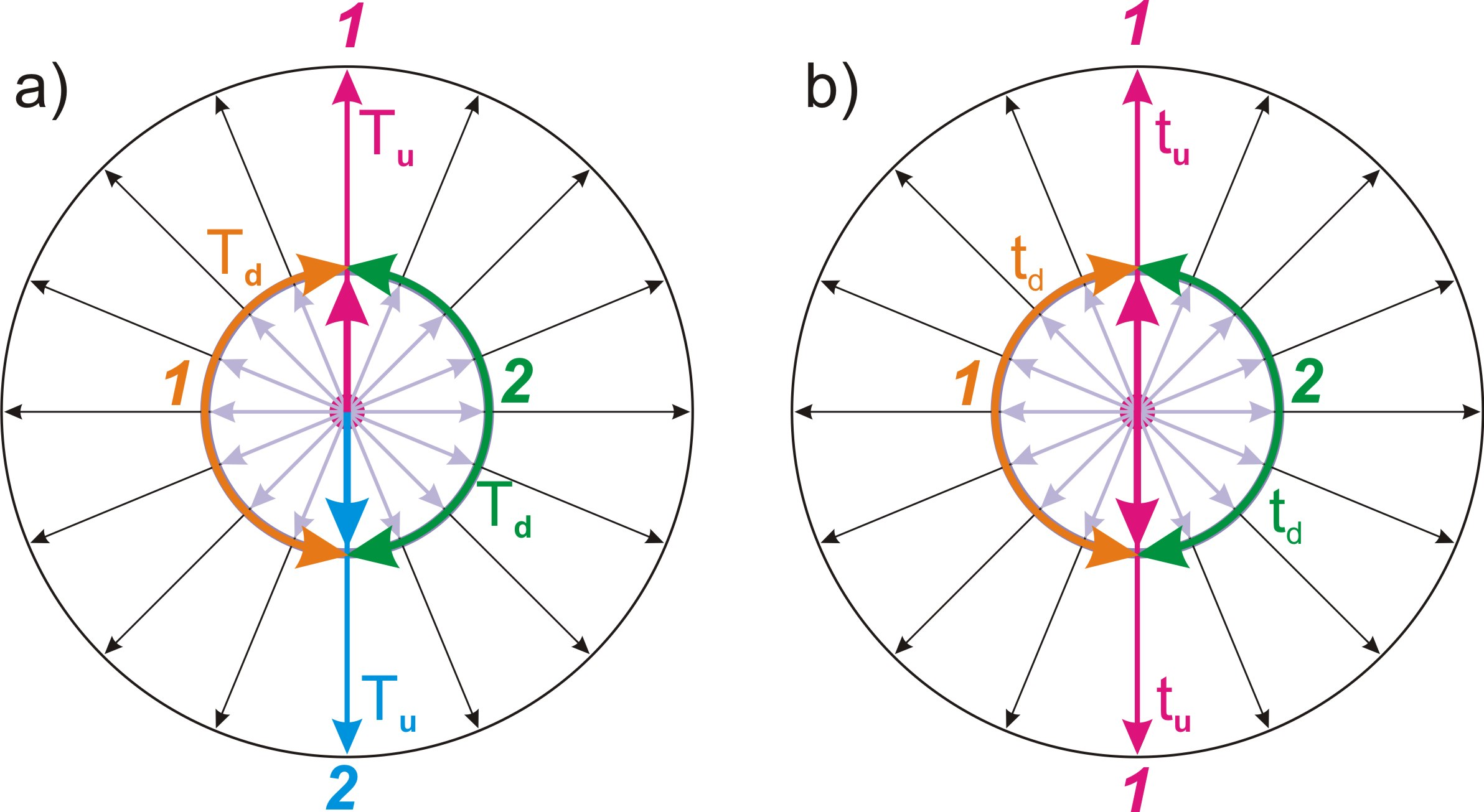Fig. 13.

Thus, the size of the complete cycle of the material part along the indefinite dimension Td is equal to a half of circumference.

In accordance with the conclusion (c29), the spot reference frame moves along the mainstay dimension Tu in one direction. This condition of movement in one direction is identically reflected in the internal space of fundamental particles, as the movement “from inside - out”. As a result, their internal space is formed by a two-dimensional projection of the elementary localization not as a superposition of alternative positions of a bidirectional diameter, as in the case of the main localization, fig. 13, a), but by a superposition of alternative positions of a unidirectional radius, fig. 13, b).

Conclusion (c51):

Relative to the local reference frame, the internal space of elementary localization is formed by a superposition of alternative positions of one direction of the definite dimension, as a radius directed “from inside - out”.

The position of the main localization in the external frame of reference relative to the rotation of its space is indefinite. Consequently, the variant of displacement of its material body by the full value of the cycle along the indefinite dimension Td is one of the variants of the superposition of its positions. In this case, the direction of the mainstay dimension Tu changes to the opposite, fig. 14, a).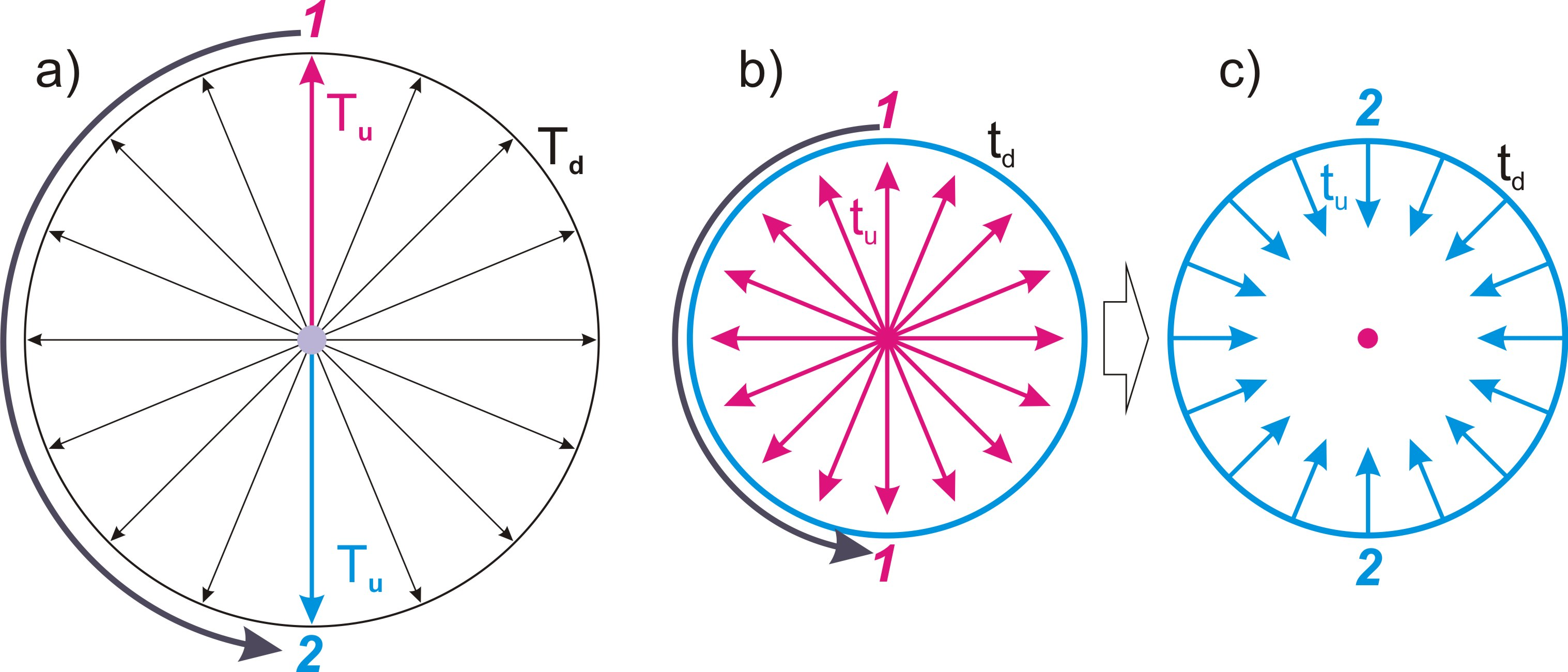Fig. 14.

Essentially, in relation to the main localization, this is a static condition that does not change anything in its state.

With regard to the fundamental particle, the direction of the projection of the definite dimension tu of which has one direction - “from inside - out”, fig. 14, b), such a shift for a full cycle along the projection of the indefinite dimension td in the spot reference frame leads to a change this direction to the opposite - “outside - in”, fig. 14, c).

Conclusion (c52):

As a result of the displacement of the fundamental particle for a full cycle along the projection of the indefinite dimension td of the potential part of the elementary localization, the direction of the projection of the definite dimension tu changes to the opposite.

In accordance with the conclusion (c48), the inner radius of the elementary localization is projected into the physical space as the diameter of the fundamental particle. Accordingly, the total size πdr of the indefinite dimension td of the potential part of the elementary localization, which is a half of circumference in its internal space, Fig. 15, a), is projected into the physical space with the size πdr of the full circumference. And two directions along the indefinite dimension td – two directions of bypass along it, fig. 15, b).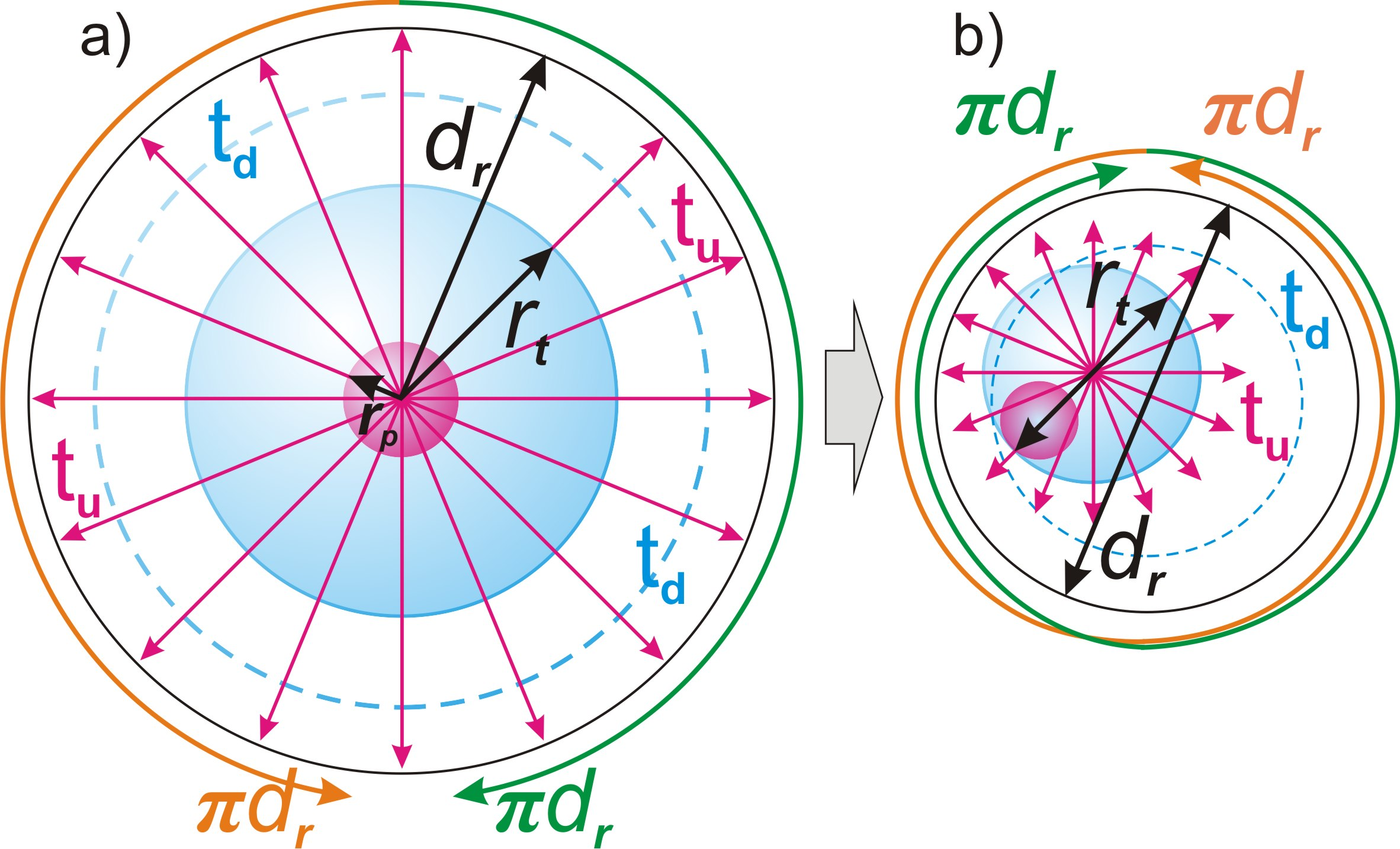Fig. 15.

This follows from the fact that the ratio of the sizes of the definiteо tu and the indefinite td dimension when projected into physical space should not change. On the other hand, the closing sphere of the internal space of a fundamental particle, in accordance with definition (d3), should not have an external side, that is, it should be a “Mobius strip”.

Thus, the full cycle of the transition of the physical body of the fundamental particle, fig. 17, a), along the projection of its internal indefinite dimension td is in the physical space a complete revolution by 360o relative to the area of its location, as a result of which the direction of the projection of the internal definite dimensiontu changes on the opposite, fig. 16, b).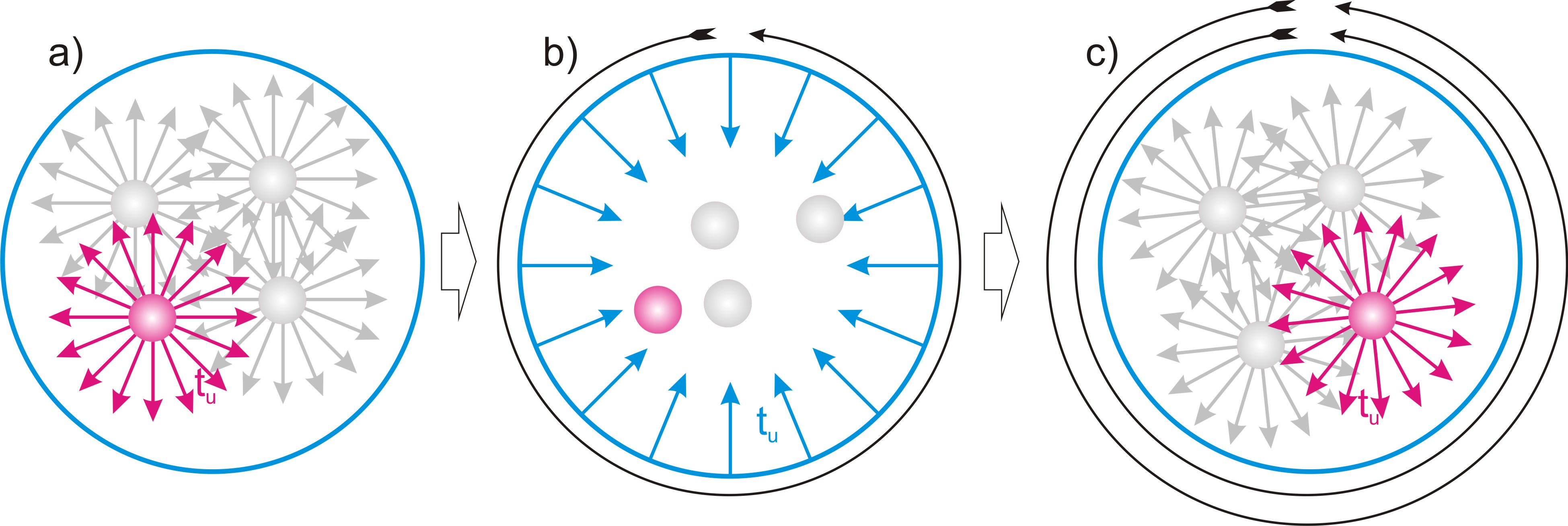Fig. 16.

To return the fundamental particle to its initial state, another complete transition cycle will be required along the projection of the indefinite dimension td, corresponding to the second revolution at 360o, fig. 16, c).

This corresponds to the concept of spin equal to 1/2 accepted in quantum mechanics.

Conclusion (c53):

The spin of a fundamental particle is 1/2.

10. Movement in physical space

Content

In the relativity of each local frame of reference, the breaking of the symmetry of one of the fundamental particles relative to the other causes a cycle of symmetry restoration, which makes them move towards each other. We will discuss this issue in the chapter “Gravity”, and now the important thing for us is that the particles begin to move relative to each other.

This shift occurs during the transition from one quantum of state to another.

As an identical part of the material part of localization, each fundamental particle in its own relativity moves along the mainstay dimension Tu with a constant speed c = dr/dt.

Conclusion (c54):

Any local frame of reference associated with any of the fundamental particles, or their group, moves along the mainstay dimension with the maximum possible speed c.

The sequence of quantums of the state of the material part is displaced along the two-dimensional structure TuTd of the potential part. It follows from this that any movement of fundamental particles, as parts of a material part, is possible only along this two-dimensional structure. But if we take into account the fact that, on the one hand, the movement along the mainstay dimension Tu always occurs at the same speed, and on the other hand, only one of the dimensions of this two-dimensional structure is reflected in the physical space - the indefinite Td, the conclusion follows:

Conclusion (c55):

Any movement of material objects in the physical space is carried out along the indefinite dimension Td of the two-dimensional structure of the potential part.

In accordance with this conclusion, for the convenience of further reasoning, we will give the corresponding name to this dimension:

Definition (d26):

A mobile dimension is an indefinite dimension Td of the two-dimensional structure of the potential part.

The localization conditions that determine the rate of displacement of the material part across the two-dimensional structure TuTd of the potential part do not in any way limit the range of possible directions of this process. As a result of imposing the conditions of the spot cycle of symmetry restoration on the main cycle, the direction of the mainstay dimension Tu in relation to one or another particle changes.

Conclusion (c56):

The direction of the vector of the mainstay dimension Tu in relation of two fundamental particles moving relative to each other is different.

As a consequence of this difference in the direction of the mainstay dimension vector Tu in the reference frame associated with one particle, its projection onto the mobile dimension Td in the relativity of another particle arises, fig. 17.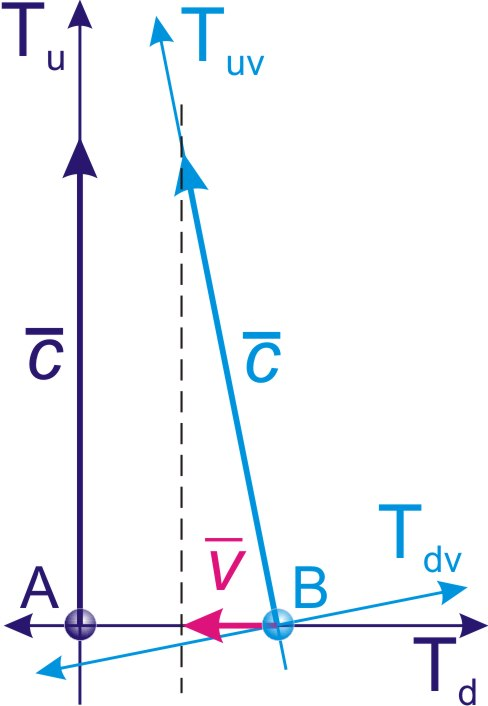Fig. 17.

On fig. 17 shows the reference frame TuvTdv moving with velocity v relative to the reference frame TuTd.

The motion vector along the mainstay dimension Tu of the reference frame TuvTdv is projected onto the mobile dimension Td of the reference frame TuTd by the motion vector with the velocity v relative to it.

Conclusion (c57):

The movement of material bodies in the physical space is a projection of their movement along the eigenvector of the mainstay dimension Tu onto the mobile dimension Td of the reference frame.

Thus, the condition determined by direction of vector of motion each moving physical object is superimposing on uncertainty condition of the mobile dimension Td in relation to it.

Conclusion (c58):

The direction of the mobile dimension Td of the spot reference frame is determined relative to each moving material object by its motion vector.

The combination of alternative conditions of the definite dimension of the physical space Ru, which determines its size, and the mobile dimension Td, along which movement is carried out in it, creates a region of spatial uncertainty of diameter ds, in which the conditions of the full size Rt of the definite dimension Ru and the size πRt of the indefinite dimension Td are combined, fig. 18.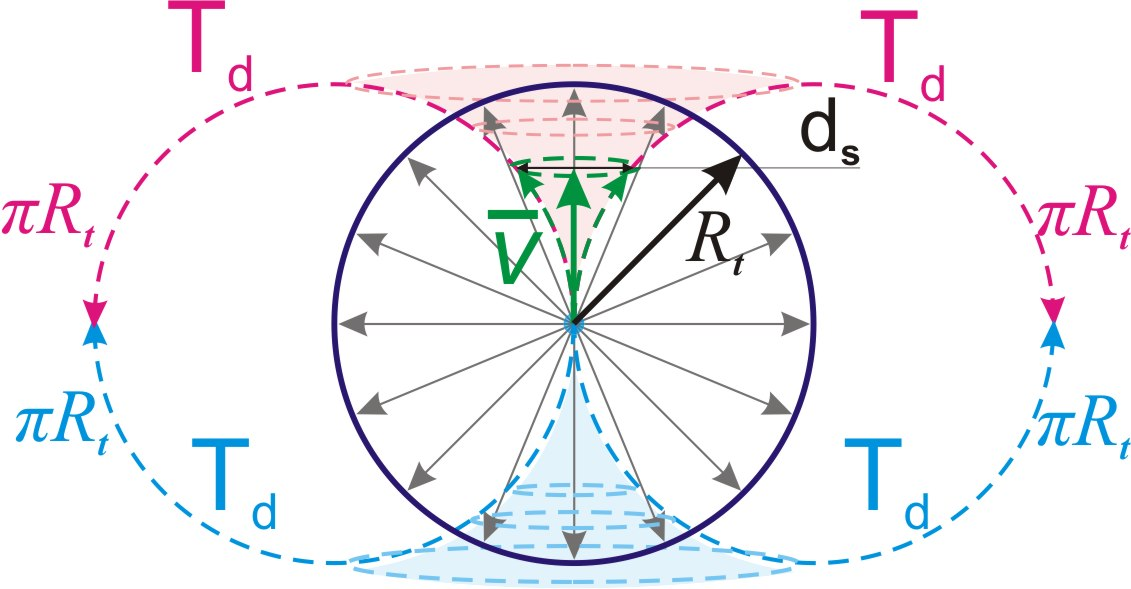Fig. 18.

When approaching the center of the reference frame, the deviation of the indefinite dimension Td from the definite dimension Ru of the physical space tends to zero.

The farther from the center of the reference frame, the larger the diameter ds of the uncertainty region. When approaching the boundary of the physical space, ds tends to the maximum size πRt .

From this it follows that the concept of motion in a straight line for physical bodies is a mathematical abstraction that does not exist in real physical space (this does not apply to the motion of light quanta, which will be shown in the corresponding chapter).

Conclusion (c58-1):

Our planet Earth is located in the center of the Universe in relation to all reference systems associated with it. At the same time, outer space, together with space objects, as it moves away from the Earth, becomes more and more uncertain in terms of spatial, and, consequently, physical properties, and distant space bodies are projected with an increased size due to an increase in the uncertainty of their position.

If you move (for example, on a spaceship) from one spot reference system to another, the geometry of the Universe will change.

It follows from this that all physical calculations relative to deep space, based on the extrapolation of the geometry of the near-Earth space and the invariance of the time vector over long distances, carry an error, the greater the more remote the region of space under consideration.

The mobile dimension Td relative to a particle at rest in the spot frame of reference is indefinite, that is, it is in a superposition of all alternative positions determined by the condition of perpendicularity to other dimensions of the physical space Ru and Rd. From this follows the conclusion:

Conclusion (c59):

The size of the projection of a fundamental particle onto the mobile dimension Td is equal to its size rt along the definite dimension Ru of the physical space.

Since, relative to the moving particle, the mobile dimension Td is projected by its motion vector, the other two dimensions of the physical space Ru and Rd are projected by a plane perpendicular to the motion vector, fig. 19, a), and the region of its location is a cylinder, fig. 19, b).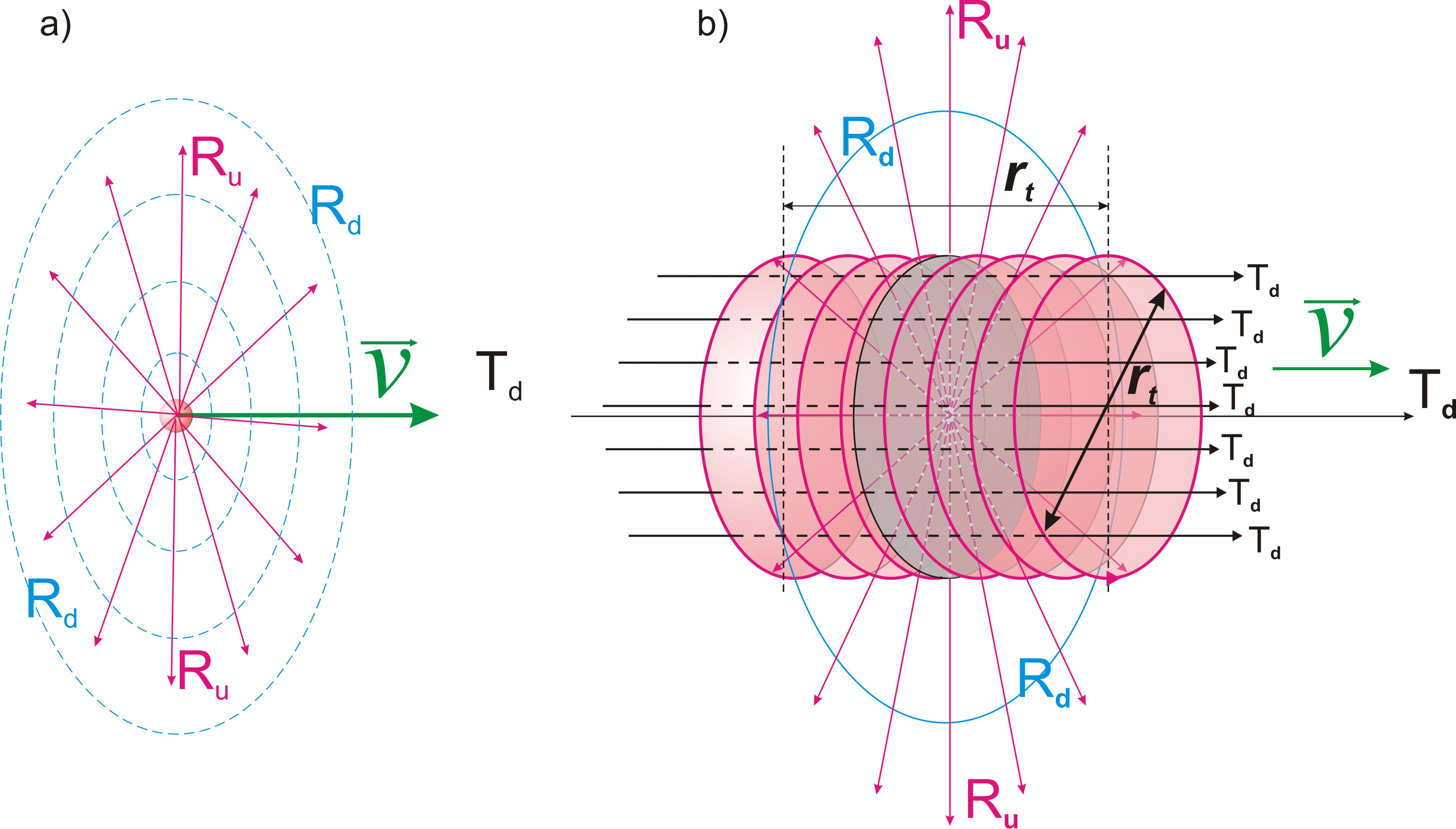Fig. 19.

Conclusion (c60):

The presence area of a moving fundamental particle in physical space is a cylinder oriented along the motion vector, the diameter and length of which are equal to its size of presence rt.

11. Inertial motion and the shape of galaxies

Content

The material part is always located in the center of localization and in each quantum of state it picks up the speed of displacement along the mainstay dimension only relative to itself. Considering the absence of any points of reference in Limitlessness, this gained speed in the quantum of state does not correlate with anything except the state quantum itself, and in each subsequent step the process of gaining speed for the transition to the next state is repeated anew.

Conclusion (c61):

The process of gaining speed by the material part in the quantum of state is identically repeated in the next quantum of state.

Thus, in each quantum of the state, the force of the cycle gives the material part acceleration:

a0 = c/dt = dr/dt2 (10)

Conclusion (c62):

The material part of the localization moves along the mainstay dimension with the same speed c = dr/dt and the same acceleration in each state quantum a0 = dr/dt2.

Each fundamental particle in its own relativity moves along the mainstay dimension Tu with the maximum possible speed c (conclusion (c54)). The direction of the vector of this motion is determined by the state of broken symmetry with respect to each particle.

Conclusion (c63):

In the absence of influences that change the state of symmetry, the direction of the motion vector of the fundamental particle along the mainstay dimension Tu is unchanged.

Accordingly, the projection of this motion into the spot frame of reference is also unchanged.

This means that if the state of symmetry remains unchanged, the speed of the particle relative to the spot reference system is preserved. Thus, we have found the reason that determines the inertia of motion in physical space.

We give the following definitions:

Definition (d27):

Inertial movement or inertia is movement in the conditions of maintaining its own relative direction of vector of the mainstay dimension.

Definition (d28):

An inertial frame of reference is a spot frame of reference associated with one or a group of fundamental particles moving by inertia.

Further, for convenience, when referring to a spot frame of reference, we will assume that we mean an inertial frame of reference.

However, in contrast to classical physics, where rectilinear uniform motion is considered inertial, it follows from this theory that only motions of cosmic bodies in circular orbits are inertial.

In this case, the angle φ between the mainstay dimension of the reference frame associated with the center of mass of both cosmic bodies and the mainstay dimension of each of the cosmic bodies remains unchanged, fig. 20.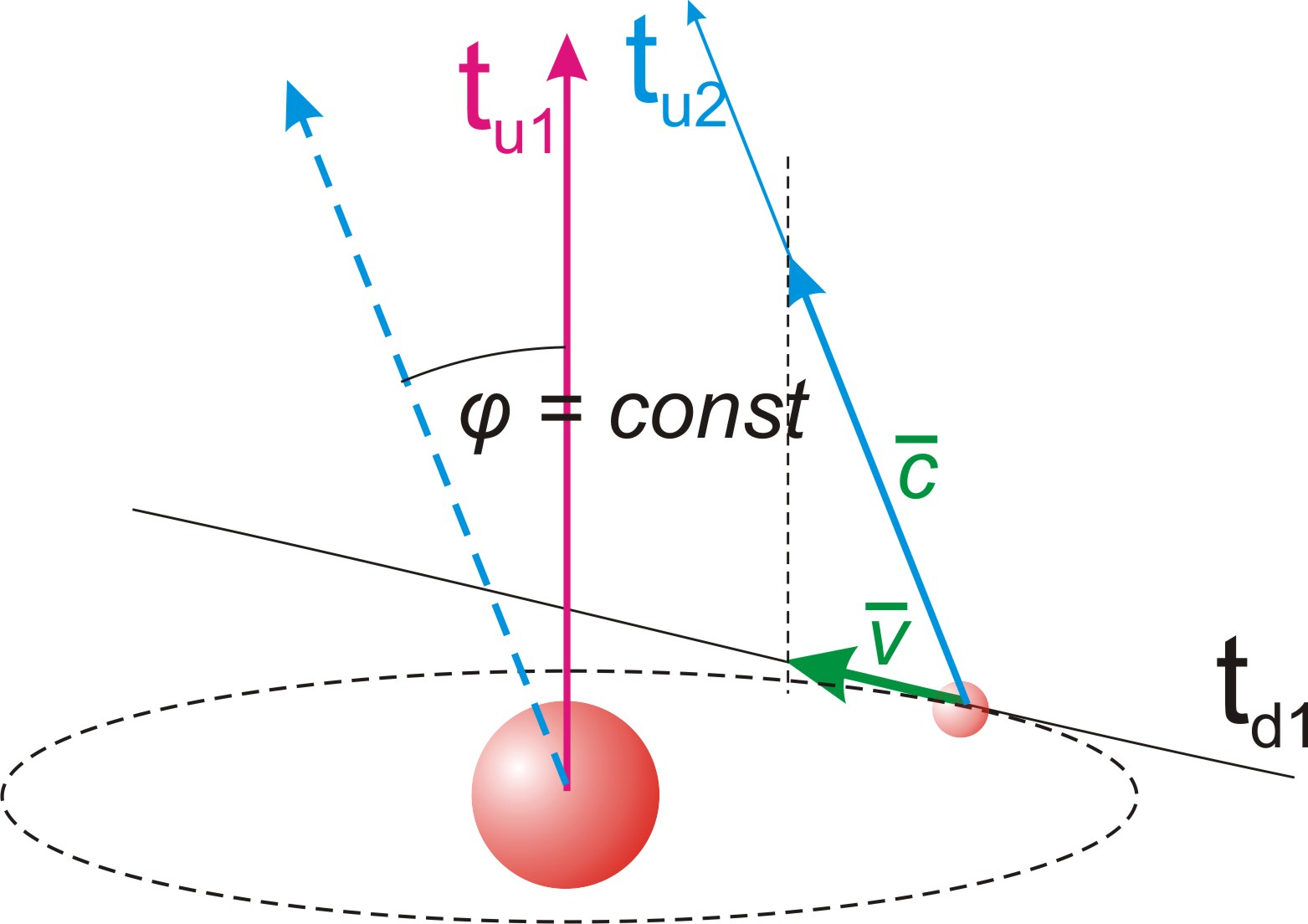Fig. 20.

But such a circular inertial motion of cosmic bodies is possible only under the condition of an insignificant radius of the orbit in comparison with the size of the Universe, in which the vector of the mainstay dimension retains its direction on the sides of the orbit remote from each other.

At large distances from super massive bodies, this movement will have a shape resembling a divergent spiral, which is what we observe in the form of galaxies, fig. 21.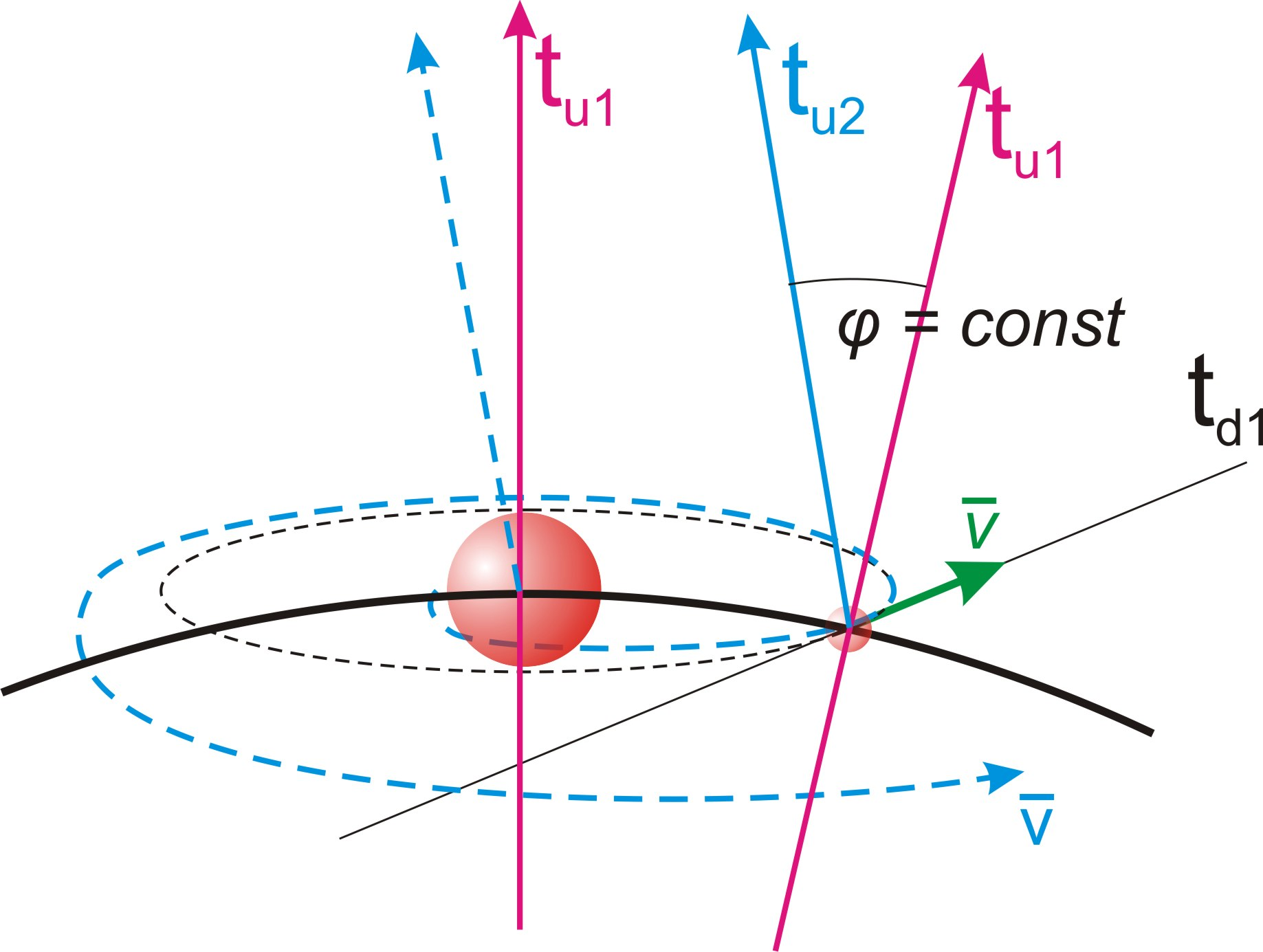Fig. 21.

Thus, this is one of the rather weighty confirmations of this theory.

Conclusion (c64):

The inertial motion at galactic distances is a divergent spiral, the divergence value of which tends to zero at small distances comparable with stellar systems, as a result of which the inertial motion of the planets occurs in circular orbits.

To this conclusion, we are forced to add another important conclusion:

Conclusion (c65):

The principle of inertiality of uniform rectilinear motion as applied to cosmic scales is a systemic error of classical physics.

If any impact on an elementary particle leads to a change in its relative direction of the mainstay dimension Tu, this changed state is preserved by the principle of inertia (conclusion (c63)) after the impact is terminated.

Conclusion (c66):

The speed of movement of elementary particles in the physical space, imparted by any influence in the quantum of state, is added to the already existing speed and remains after the termination of the influence.

Thus, if some interaction of particles gives any of them in the quantum of state an acceleration a of motion along the mobile dimension Td, its motion becomes uniformly accelerated:

v = anidt (11), where ni – the number of state quanta in which the acceleration acted.

nidt = t (12), where t – the time of action of the acceleration.

Accordingly with this:

v = at (13)

12. Quantum of the state of the physical process

Content

Any physical process is an interaction of projections of elementary localizations into the physical space, the movement in which (conclusion (c55)) is carried out along the mobile dimension. In this case, the rate of interaction cannot exceed the maximum possible speed c. It follows from this that the projection of the motion of one fundamental particle into a reference frame associated with another fundamental particle will be reflected in it no faster than after a time interval equal to the ratio of the distance between them to the speed c.

Conclusion (c67):

A change in the state of one fundamental particle can affect the state of another fundamental particle no faster than after a time interval equal to the ratio of the distance between them to the speed c.

This ratio represents the minimum time interval or quantum of extension of the process of interaction of two particles.

Definition (d29):

The size of a physical process is the maximum distance between its parts in physical space.

Definition (d30):

The quantum of extension of a physical process is the ratio of its size to the maximum possible speed c.

dtr = Rf/c = Rfdt/dr (14), where dtr – the size of the quantum of extension of the physical process according to the mainstay dimension Tu in units of time, Rf – spatial size of the physical process.

Within the frames of the process determined by the quantum of extension, the relationship of the parts of this process is indefinite.

Definition (d31):

The quantum of state of a physical process is its minimal indivisible state in the sequence of states, in which each of the parts of this process is reflected in every other part of it.

Thus, the quantum of state of a process in the physical space is equal to its size Rf, and in the projection onto the mainstay dimension its size is equal to the size of its quantum of extension dtr.

Within a state quantum of a process, its parts are in uncertainty relative to each other.

Conclusion (c68):

In the state quantum of a physical process, its parts are a superposition of all possible alternative combinations.

Each state quantum of a process is a realized act of the relationship of all parts of this process.

Conclusion (c69):

During the transition of the physical process by quanta of state, in each previous quanta of state, the superposition of alternative combinations of all parts of the process is reduced to one realized variant.

If the spot frame of reference is associated with a certain physical process or body, then it must reflect the quantum of the state of the process (body) as the own quantum of state of the frame of reference.

Definition (d32):

The quantum of the state of the spot reference frame is the holistic one-moment state of the reference frame corresponding to the quantum of the state of the physical process or body with which this reference frame is associated.

Conclusion (c70):

The sequence of events and physical processes in the spot reference frame within its own state quantum is indefinite and is a superposition of all possible alternatives.

Another important conclusion follows from the conclusions drawn in this chapter:

Conclusion (c71):

Any comparison of processes located in space at a distance R is correct only with respect to a reference frame, the size of which is greater than R, and the size of the state quantum along the mainstay dimension in units of time is greater than R/c.

The uncertainty of events separated by distance R and time less than R/c is called the relativity of simultaneity in Theory of relativity. However, this conclusion about the relativity of simultaneity is completely ignored when asserting that the flow of time in a moving reference frame slows down relative to a resting one, which leads to a conceptual error.

On fig. 22 shows the resting frame of reference tutd. And the frame of reference tuvtdv moving with speed v relative to the frame tutd.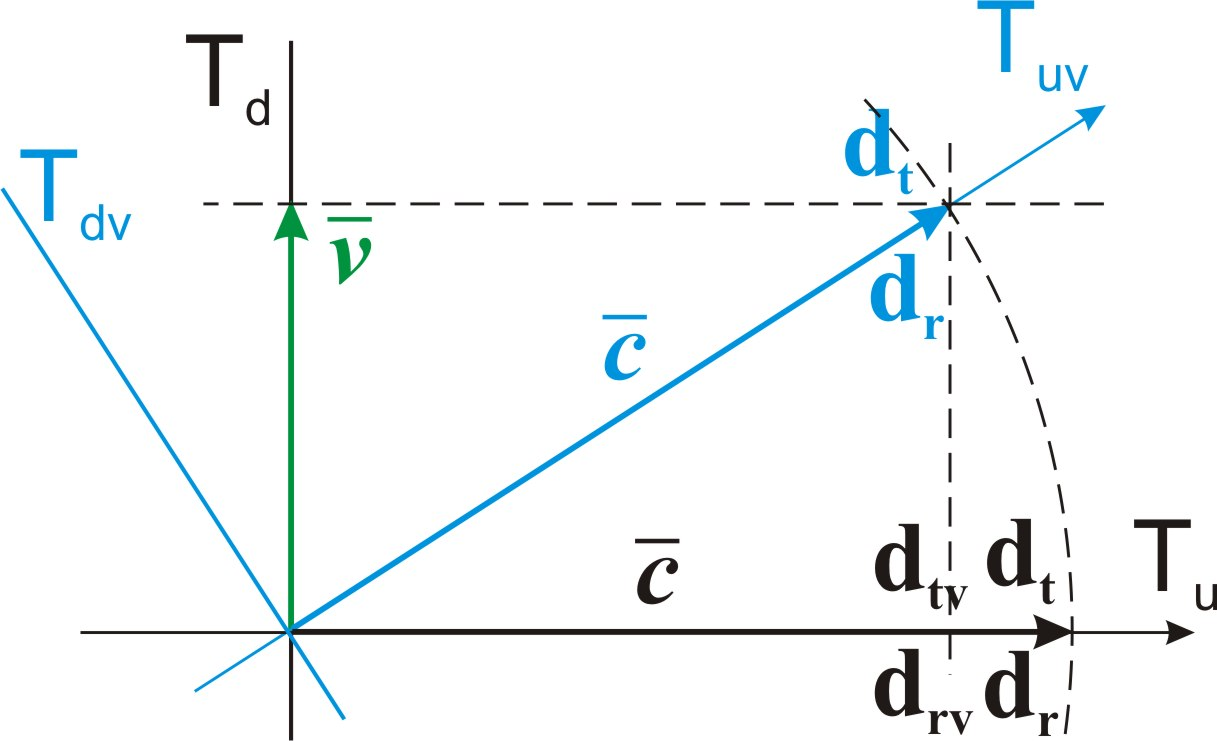Fig. 22.

The size of the quantum of extension drv of the moving frame of reference is reduced in projection onto the mainstay dimension of the frame of reference at rest.

From the identity of triangles, we find:

drv/dr = dtv/dt =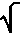(c2-v2)/c =(1-v2/c2) (15), where drv – the size of the quantum of extension of the moving frame of reference in units of length, dtv – the size of the quantum of extension of the moving frame of reference in units of time.

drv = dr(1-v2/c2) (16)

dtv = dt(1-v2/c2) (17)

The interpretation of the reduction in the size of the quantum of extension dtv of the moving reference frame relative to the quantum of extension dt of the resting frame in units of time is erroneous.

In accordance with the conclusion (c70), all processes within the size of the quantum of extension dt according to the mainstay dimension are indeterminate. From this it follows that the boundaries of the quantum of extension dtv of the moving frame of reference are indefinite in the relativity of the quantum of extension dt of the resting frame, fig. 23.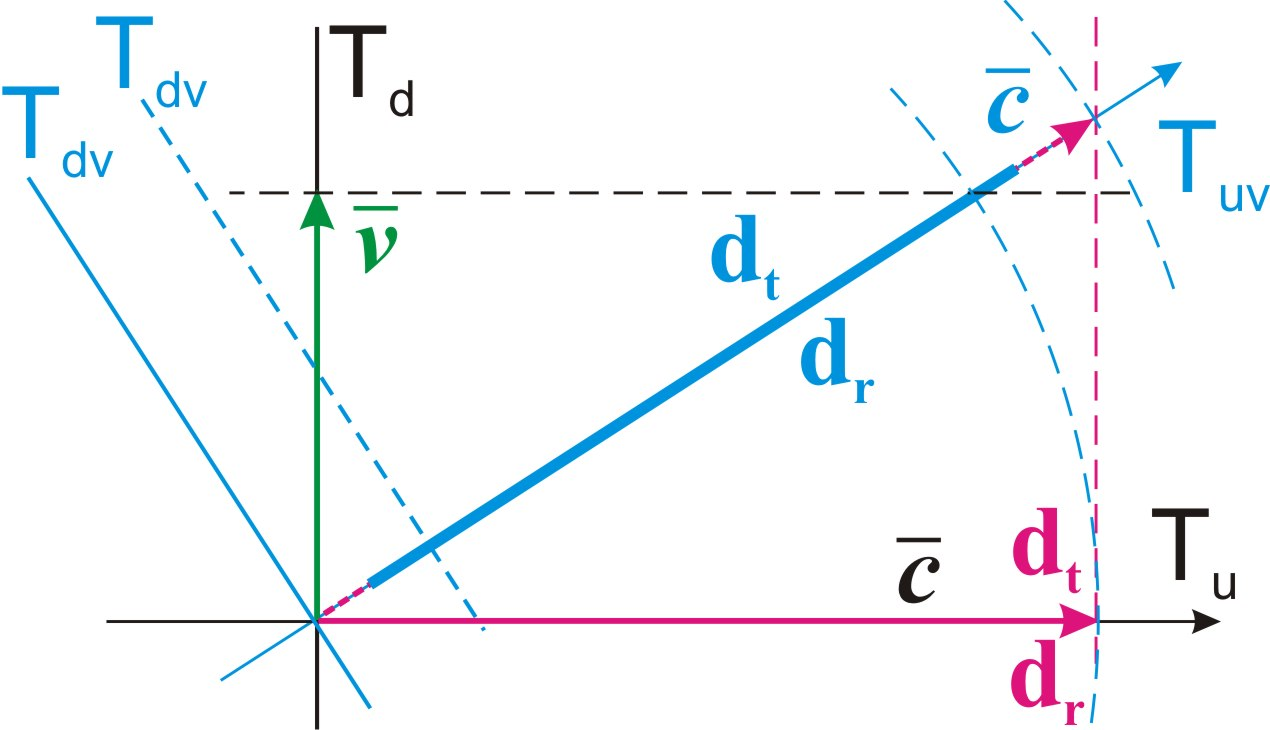Fig. 23.

On the other hand, in the relativity of any spot reference frame, all material objects projected into it, regardless of the relative speeds of their movement, pass with it from one state quantum to another in the sequence of time and cannot lead or lag behind this process.

Conclusion (c72):

In the relativity of the spot reference frame, the displacement in the state quantum is the same for all parts of the material part of the localization, regardless of their velocities, and is equal to dr.

Taking into account the uncertainty of processes within the quantum of extension, we conclude:

Conclusion (c73):

The size of quantum of extension of a resting frame of reference includes the quanta of extension of all moving frames of reference, as a superposition of all their possible alternative positions.

Conclusion (c74):

Time, as a sequence of state quanta, flows in the same way for all material objects in the relativity of any spot reference frame, regardless of the speed of their movement.

In accordance with the conclusion (c47), the size of a fundamental particle in the physical space is determined by its size according to the definite dimension Ru of the physical space, which is projected from the quantum of extension (formula (07)).

rt = Rt/n = drnt/n

Thus, relative to the resting frame of reference, the size of a moving fundamental particle is reduced:

rtv = ntdrv/n = ntdr(1-v2/c2)/n (18)

rtv/rt =(1-v2/c2) (19)

rtv = rt(1-v2/c2) (20)

Conclusion (c75):

The size of a moving fundamental particle relative to the spot frame of reference with a speed v is reduced relative to the size of a particle at rest in proportion to(1-v2/c2).

Let us pay attention to the fact that the size of the state quantum of the reference frame associated with the planet Earth is equal to the ratio of its diameter to the speed c. This ratio is exactly equal to the minimum period of time for a person to perceive the successive stages of the movement of the objects he observes (24 frames per second). If we take into account the fact that the interval for taking readings by an experimenter from any physical device cannot be less than the size of this state quantum in units of time, we can come to interesting conclusions, but this topic is beyond the scope of this study.

13. The force and the mass

Content

According to the tradition of mechanics, the action that gives acceleration is called force, and the material characteristic of a physical body, inversely proportional to which the force gives it acceleration, is called mass.

In accordance with the conclusion (c44), the area of physical space, determined by the size of presence of a fundamental particle, is a space uniformly filled with matter. Thus, the size of presence of a particle is its only material characteristic.

According to our theoretical model, all forces are determined by the action of symmetry restoration cycles.

With respect to the spot reference frame, the magnitude of the symmetry breaking in the main localization is determined by the ratio of the size of its material part to the size of the potential part. Along the mainstay dimension Tu, the ratio of its total size Rn to the size of the material part along it dr is equal to n. According to formula (05):

Rn = ndr

The same magnitude of symmetry breaking along the mainstay dimension also pertains to fundamental particles, since they have the same size dr along it as the entire material part of the main localization. Moreover, this size, in accordance with conclusions (c73) and (в74), does not depend on the velocity of the particle.

Along the mobile dimension Td, the material part of the main localization is not singled out and occupies entire size πRt of this dimension in each quantum of state nt. In accordance with the conclusion (c40), the disturbance of symmetry by fundamental particles along it should be counted with respect to this size.

In accordance with the conclusions (c8) and (в42), the elementary localization should be n times less than the material part of the main localization along both its dimensions Ru and Rd. Thus, the fundamental particle is singling out along the indefinite dimension Rd of the material part with the size πrt, which is identically reflected by the similar singling out size πrt along the second indefinite dimension of the physical space - the mobile dimension Td. This does not contradict the conclusion (c59), according to which the fundamental particle is projected in physical space onto a mobile dimension of size rt, fig. 24, a).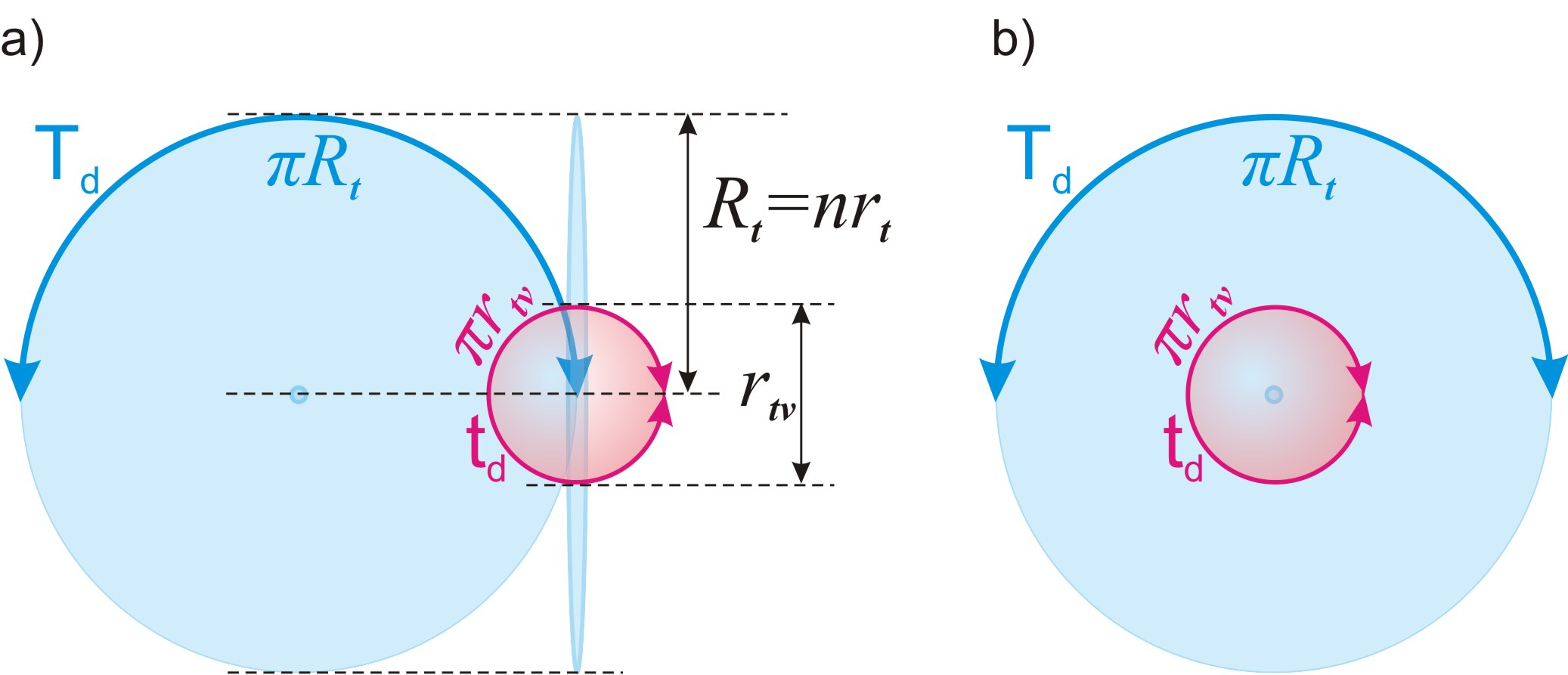Fig. 24.

The mobile dimension Td is projected relative to the elementary localization by the indefinite dimension td of its potential part, and it, in turn, is projected into the physical space with size πrt, fig. 24, b).

But in accordance with the conclusion (c75), this size πrt relative to the local reference frame associated with the material part of the main localization will have only that fundamental particle, the speed of which according to the mobile dimension Td relative to the local frame of reference is equal to zero.

We give the following definitions:

Definition (d33):

The ideal frame of reference is a spot frame of reference, the speed of which along the mobile dimension Td relative to the local frame of reference is equal to zero.

Definition (d34):

The state of rest is the state of a fundamental particle, the speed of which relative to the ideal reference frame is equal to zero.

Thus, the fundamental particle at rest is singled out for each of the dimensions of the potential part with a coefficient n.

We give the following definitions:

Definition (d35):

Definiteness by dimension is the ratio of the size of the extent of a dimension in the ideal reference frame, relative to which a fundamental particle is singled out, to its size of singling out along this dimension.

Definition (d36):

The material definiteness of a fundamental particle is the product of its definiteness along each of the dimensions of the two-dimensional structure TuTd of the potential part of the localization.

Conclusion (c76):

The definiteness along the mainstay dimension Tu should be counted relative to its full size Rn, and the definiteness along the mobile dimension Td should be counted relative to its size πRt in the quantum of state nt.

Thus, if we define material definiteness as mass:

Definition (d37):

The mass of a fundamental particle is its material definiteness, expressed in units of mass.

m = ku (21), where m – the mass of the fundamental particle, u – he material definiteness, k – coefficient of the system of measures,

we come to the traditional formula of mechanics:

f = ma (22)

For a fundamental particle at rest, we can write:

un = n2 (23), where un – material definiteness of a fundamental particle at rest.

Let us take the mass of a fundamental particle at rest as a mass unit and denote it by dm.

dm = kun = kn2 (24)

k = dm /n2 (25)

Thus, the mass of a fundamental particle does not depend of the stage of the symmetry restoration cycle, which is determined by the number of passed state quanta. The same applies to the mass of any physical bodies, consisting of fundamental particles and their identical parts (projections).

Conclusion (c77):

Mass as a material characteristic of singled out parts of localization does not depend of the stage of the main cycle and the number of passed state quanta.

Reducing the relative size of a moving fundamental particle (conclusion (c75)) relative to the ideal frame of reference leads to an increase in its definiteness along the mobile measurement Td.

Considering these conditions, we can write:

uni = unrt/rtv (26), where uni – material definiteness of a fundamental particle at motion.

According to formula (19):

rtv/rt =(1-v2/c2)

uni = unrt/rtv = un/(1-v2/c2) (27)

mnv = dm/(1-v2/c2) (28), where mnv — the mass of a fundamental particle in motion with a speed v relative to the ideal frame of reference.

This formula is valid for any physical body consisting of fundamental particles, their parts and identical projections.

mv = m0/(1-v2/c2) (29), where m0 — the mass of a physical body at rest, mv — the mass of a physical body in motion with a speed v relative to the ideal frame of reference.

Conclusion (c78):

The mass of a physical body when it moves along the mobile dimension Td relative to the local reference system increases proportionally to 1/(1-v2/c2).

Unlike the reduction in size, this increase in the mass of a moving fundamental particle is in a certain sense absolute and does not depend on the choice of frame of reference.

Thus, regarding the change in the size and mass of a moving body, our model is in full agreement with the formulas of relativistic motion accepted in physics.

Considering the movement of the spot reference frame associated with our planet Earth relative to the ideal reference frame, the mass of the fundamental particle under Earth conditions will be greater than the mass of the fundamental particle dm at rest. Let us designate the mass of the fundamental particle under the conditions of the Earth as dmv.

dmv = dm/(1-vg2/c2) (30), where vg – the speed of the Earth relative to an ideal frame of reference.

14. The energy of the mass

Content

According to the tradition of mechanics, energy is defined as the product of force on distance and represents the work of the force.

Each fundamental particle in its relativity moves only along the mainstay dimension Tu with the same speed c under the action of the force of the main cycle.

Since each quantum of state along the mainstay dimension Tu correlates only to itself, the work of the force of main cycle along the mainstay dimension Tu in each quantum of state is completely reset to zero and is performed again in the next state quantum.

Conclusion (c79):

The energy of motion of a fundamental particle along the mainstay dimension Tu is the work of the force of the main cycle on its displacement in the quantum of state.

The force of the main cycle of symmetry restoration in each quantum of state produces a displacement of the fundamental particle, on which it acts, by the size of the quantum of extension dr.

ei = fidr (31), where ei – energy of motion of a fundamental particle along the mainstay dimension Tu, fi – the force of the main cycle acting on the fundamental particle along the mainstay dimension Tu.

All fundamental particles move along the mainstay dimension Tu with the same acceleration in the state quantum a0 = dr/dt2 , formula (10).

fi = dma0 = dmdr/dt2 (32)

ei = fidr = dmdrdr/dt2 = dmc2 (33)

Thus, we arrive at a well-known formula in physics.

Zeroing the energy of movement of fundamental particles along the mainstay dimension Tu in each previous state quantum does not mean the "disappearance" of this energy. It passes into their potential energy in physical space by increasing its size and, as will be shown in the following chapters, the forces acting in it. Thus, we come to the conclusion:

Conclusion (c80):

The potential energy of fundamental particles in the physical space grows in proportion to the passed quanta of state nt.

15. Gravity

Content

The mutual arrangement of fundamental particles creates a precedent for the disturbance of symmetry of one particle relative to another. This disturbance causes many cycles of symmetry restoration, identical to the main cycle. Namely identical in accordance withtheorem (t2), since each of them is a part of the localization. Let us call this phenomenon gravity.

Definition (d38):

The gravitational cycle or gravitation is the process of restoring symmetry with respect to the spot reference frame, broken by one fundamental particle relative to another.

The identity of the gravitational cycle to the main cycle is reflecting in the circular motion of cosmic bodies, fig. 25, a).Fig. 25.

According to the conclusion (c64), this circular motion is inertial.

The force vector of the interaction of bodies at opposite ends of the orbit is directed oppositely, which identically corresponds to the two poles of the symmetry restoration cycle in the main cycle, fig. 25, b). Thus, the movement of a cosmic body along a semi-orbit must be identical to the complete main cycle. After passing through the half-orbit, the gravitational cycle is repeated again in the second half to return to its original state.

The force of main cycle acting on a fundamental particle is a consequence of its material definiteness in respect of the two-dimensional structure TuTd of the potential part of localization.

It follows from the principle of identity of cycles that the force of the gravitational cycle should be determined similarly by the definiteness of a fundamental particle in relation to size of the cycle.

Accordingly, if we divide the distance R by the size of presence of the fundamental particle rt, we get the determining integer of the gravitational cycle:

ng = R/rt (34), where ng – the determining integer of the gravitational cycle, R – the distance between space bodies.

In the main cycle, the material and potential parts are identical and differ only in that the frame of reference is connected with the material part. Each of them consists of n2 identical parts, fig. 26, a).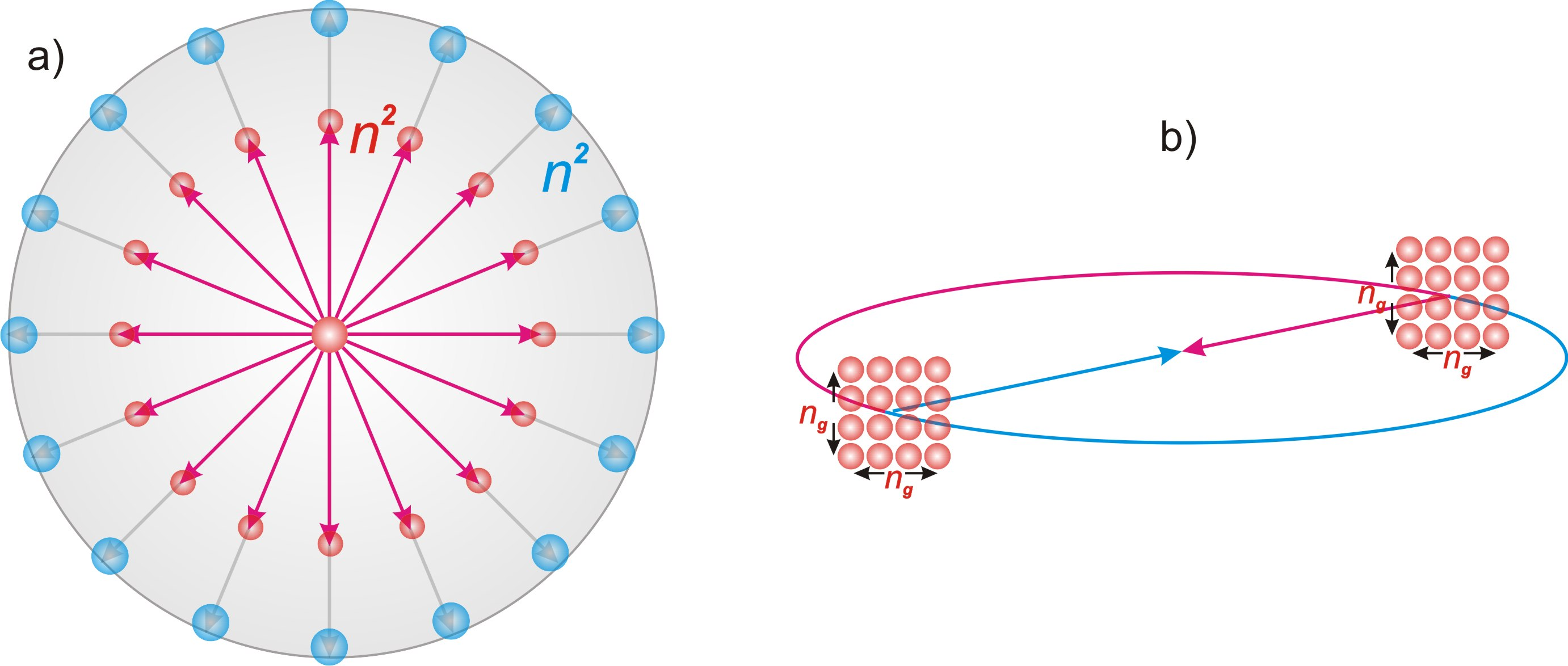Fig. 26.

From this, it follows that the presence of ng2fundamental particles at each opposite poles of the gravitational cycle is one of the main conditions for its identity to the main cycle, fig. 26, b).

Let us call this condition a complete gravitational cycle.

Definition (d39):

A complete gravitational cycle is a gravitational cycle, on each of the opposite poles of which there is a number of fundamental particles equal to the square of its determining integer.

However, there is an important difference between the compared cycles. It consists in the fact that the symmetry disturbance in the main cycle occurs with respect to the two-dimensional structure TuTd of the potential part, Fig. 27a). In turn, the gravitational cycle occurs in physical space, in which movement is possible only along the mobile dimension Td (conclusion (c55)). Thus, the process of symmetry disturbance in the gravitational cycle is one-dimensional, fig. 27, b).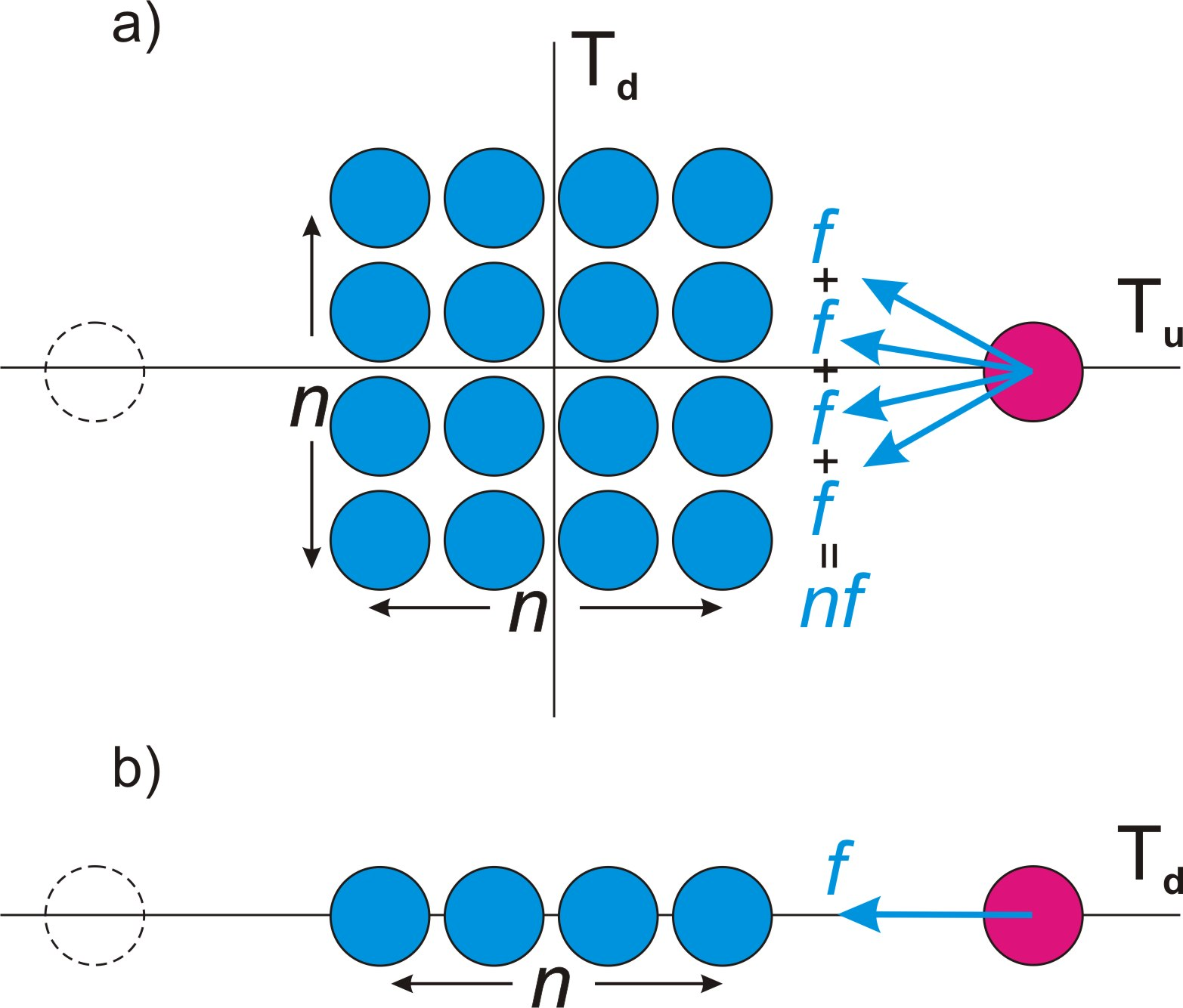Fig. 27.

Conclusion (c81):

The main cycle is passing through the two-dimensional space of potential part of main localization; in turn, the gravitational cycle is a one-dimensional projection into the physical space of it.

From the condition of the projection of each part of one dimension into each part of another, it follows that the force of the two-dimensional main cycle in the projection onto the one-dimensional process decreases by n times.

The second condition for the identity of cycles follows from the fact that the force of the main cycle going along the definite mainstay dimension Tu is projected into the physical space onto the mobile dimension Td, which is indefinite.

The indefinite dimension Td is π times longer than the definite Tu (conclusion (c17)). The work of the force of the cycle on the displacement of the material part for a full cycle for each of the dimensions should be the same. From this follows the conclusion:

Conclusion (c82):

The effect of the force of the symmetry restoration cycle along the indefinite dimension is π times weaker than along a definite one.

Taking into account the previous condition, we conclude:

Conclusion (c83):

The force of the complete gravitational cycle is πn times less than the force of the main symmetry restoration cycle, where n – is the determining integer of localization.

In the main cycle, a fundamental particle with mass dm moving along the mainstay dimension at a speed of c = dr/dt is affected by the force f0, which gives the acceleration a0 = c/dt = dr/dt2 (formula (10)) in a quantum of state.

f0 = dma0 = dmdr/dt2 = dmс2/dr (35)

Accordingly, in a complete gravitational cycle along the mobile dimension, a force πn times less will act on a fundamental particle:

fgn = f0/πn =dmс2/πndr (36), where fgn – force of influence of a full gravitational cycle on one fundamental particle.

This force must act on each of the ng2 fundamental particles of one pole from all ng2 fundamental particles of the other pole.

Accordingly, the force of gravitational attraction between two fundamental particles fg0 will be ng2 times less than the force of a complete gravitational cycle. According to formula (34):

fg0 = fgn/ng2 = dmс2/ng2πndr = dmс2rt2/R2πndr (37)

Taking into account formula (07):

rt = drnt/n

fg0 = dmс2nt2dr /R2πn3 (38)

This force is the force of gravitational attraction between two unit masses dm, in the ideal frame of reference.

The force acting between any two masses consisting of the fundamental particles will be proportional to the ratio of each mass of them to the mass unit dm.

fg = (m1/dm)(m2/dm)dmс2nt2dr /R2πn3 = m1m2с2nt2dr /R2πn3dm (39)

Let us equate the resulting formula for the force of gravitational interaction to that adopted in physics:

Gm1m2/R2 = m1m2с2nt2dr /R2πn3dm (40)

We get the value of the gravitational constant:

G = с2nt2dr /πn3dm (41)

All components of this formula are constants, except for nt, the number of quanta of state that localization have passed in main cycle.

Conclusion (c84):

The gravitational constant grows in proportion to the square of the passed quanta of state nt.

This conclusion agrees with the conclusion (c80) about the increase in the potential energy of fundamental particles in physical space in the course of the main localization cycle.

Let us pay attention to the fact that if, in accordance with formula (09), in the formula of the gravitational constant we replace drnt2/n2 with rp, we will get the formula: G=c2rp/πndm. Further, if we substitute in this formula instead of dm the known mass of the neutron and the value n = 2128, we will obtain a value for rp, which coincides with the experimental value of the neutron diameter within the frame of experimental error. Given the orders of numbers used, this "coincidence" is more than enough to know that we are on the right track. But further, we are waiting for much more "surprising" coincidences of a number of theoretically obtained values with the corresponding experimental data.

16. Decay of a fundamental particle or beta decay of a free neutron

Content

Any spot frame of reference associated with one or another cosmic body has a non-zero speed of inertial motion relative to the ideal frame of reference.

As a consequence of the motion of the spot frame of reference associated with the Earth with the speed vg, its own relative vector of the mainstay dimension Tu has an angle of deviation from the direction of the mainstay dimension Tu0 of the ideal frame of reference, fig. 28, a).

This angle of deviation of the mainstay dimension Tu must be identically projected into the inner space of elementary localization by the angle of deviation of the direction of its definite dimension of the potential part tu from the analogous direction at rest tu0.

This deviation of the direction of the internal definite dimension tu of the elementary localization is projected into the physical space in relation to the fundamental particle.

As a result, the physical body of the fundamental particle acquires the corresponding shift speed in the state quantum along the projection of the internal indefinite dimension td, fig. 28, b).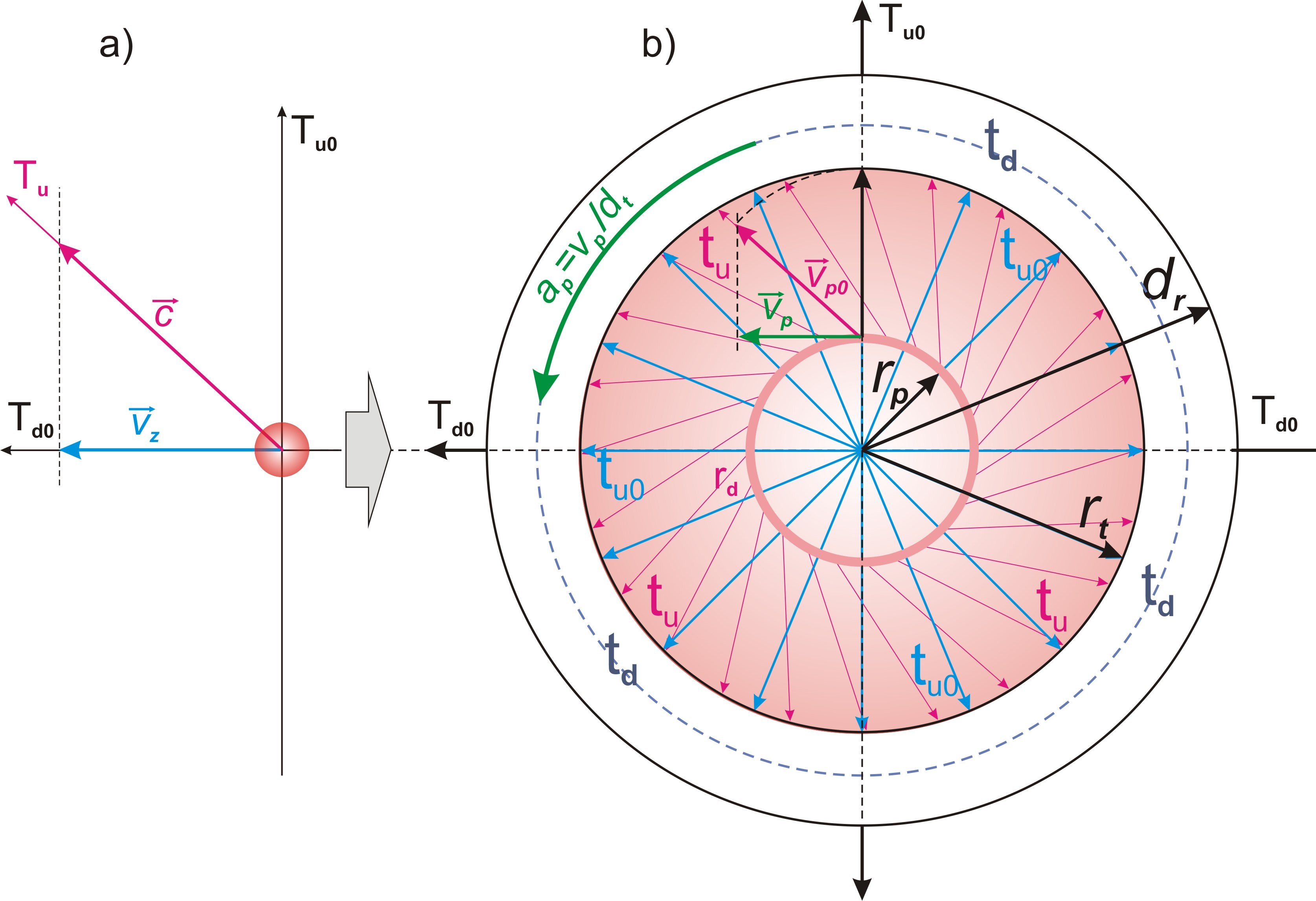Fig. 28.

The ratio of the speed vp of this shift of the material part of the elementary localization along the internal indefinite dimension td to the speed vp0 of the shift along the internal definite dimension tu in the state quantum, according to the principle of identity with the main localization (theorem (t2), conclusion (c41)) should be equal to the analogous ratio of the speed vg movement of the spot reference frame along the mobile dimension Td to the speed c of its movement along the mainstay dimension Tu in the main localization, fig. 28, a).

vp/vp0 = vg/c (42)

In accordance with the conclusion (c45), the physical body of a fundamental particle is simultaneously in the conditions of the projection of the inner cycle of elementary localization and in the conditions of physical space.

According to the conditions of physical space, the speed of this movement, gained by the physical body in the state quantum, is preserved, and, in accordance with the conclusion (c66), this change in speed begins to be summed over the sequence of state quanta, and the movement of the physical body along the internal indefinite dimension td becomes uniformly accelerated, fig. 28, b).

The acceleration of this internal movement of the material part of the elementary localization ap is equal to the ratio of the speed vp it picks up in each quantum of state to the quantum of extension dt:

ap = vp/dt = vgvp0/cdt (43)

Let us find the speed vp0.

The time of passing physical processes is so much less than the time of the full cycle of the Universe that the size of presence of the fundamental particle rt can be considered unchanged.

The increase in the size of the physical body of the fundamental particle rp for one one-moment interval dt is equal to rt/n.

Accordingly, the displacement velocity of the physical body of a fundamental particle along the indefinite dimension td of its localization is equal to:

vp0 = rt/ndt (44)

Taking into account formula (07):

rt = drnt/n

vp0 = rt/ndt = ntdr/dtn2= cnt/n2 (45)

Thus, we get:

apv = vgvp0/cdt = vgnt/dtn2 (46)

Taking into account formula (2):

apv = vgnt/dtn2= vgntc/drn2 (47)

In accordance with the conclusions (c50) and (c17), the total maximum size of an indefinite dimension td of elementary localization is equal to πdr.

Thus, as a result of passing by the physical body of a fundamental particle of this entire distance equal to πdr, the cycle of elementary localization will be completed, and it will pass into a new cycle. Let us find the time tn for the physical body of the fundamental particle to pass throw its inner cycle along the projection of the indefinite dimension td f the potential part of the elementary localization. According to the formula for the distance traveled in uniformly accelerated motion:

πdr = apvtn2/2 (48)

tn =(2πdr/apv) =(2πdr2n2/cntvg) = ndr(2π/cntvg) (49)

As a result of the transition along the full cycle of elementary localization, the physical body must leave the space determined by the conditions of the projection of this cycle and go into the physical space external to it. As a result, the fundamental particle decay into three parts, which are separately projected into the physical space. These parts are: 1. - the physical body, as a projection of the material part of the elementary localization, 2. - the projection of the potential part of the elementary localization, 3 - the projection of the full completed cycle of the elementary localization along its internal indefinite dimension td.

Conclusion (c85):

The movement of the spot reference frame relative to the ideal reference frame leads to the decay of the fundamental particle, as a projection of the inner cycle of elementary localization into the physical space, into three separate parts.

Conclusion (c86):

The time interval tn = ndr(2π/cntvg) is the lifetime of a fundamental particle before its decay in the relativity of the spot frame of reference, connected with the Earth and moving at a speed vg relative to the ideal frame of reference.

This decay of a fundamental particle fully corresponds to the beta decay of a free neutron, which leads us to the logical conclusion:

Conclusion (c87):

The fundamental particle of our Universe, as a localization in Limitlessness, is a free neutron.

In the following chapters, we will confirm this conclusion with precise calculations based on experimental data.

All new material particles resulting from the decay of a fundamental particle are identical projections into the physical space of elementary localization or its parts under certain limiting conditions.

We give the following definition:

Definition (d40):

An elementary particle is a full or partial projection of an elementary localization into physical space, identical to it and representing a material object with a strictly limited and indefinite inner space.

17. Proton and electron

Content

In accordance with the conclusion (c52), as a result of the displacement of the physical body of the neutron, as a fundamental particle, by a full cycle along its internal indefinite dimension td, the direction of projection of the definite dimension tu of the inner cycle of elementary localization is reversed.

Conclusion (c88):

The projection of a definite dimension tu f the inner cycle of elementary localization relative to the physical bodies of the neutron and proton has the opposite direction.

At the same time, as a result of reversion the direction of the projection of the internal definite dimension tu from “inside - out” to “outside - in”, the projection of a new cycle of the internal space of elementary localization should “turn inside out” and be superimposed on the physical space.

Conclusion (c89):

As a result of the decay of a fundamental particle, the projection of the inner cycle of elementary localization is superimposed on the physical space.

The exit of the physical body of the neutron beyond the projection of the potential part of the elementary localization does not affect its physical size rp, which is determined exclusively by the stage of the main cycle.

This condition leaves no doubt that in its free position in the new cycle imposed on the physical space, the physical body of the neutron becomes a proton.

Conclusion (c90):

The proton is an independent projection into the physical space of the material part of the elementary localization with the projection of the internal definite dimension tu reversed to the opposite direction. .

Conclusion (c91):

The size of the physical bodies of a neutron and a proton is the same.

Until the decay of the projection of the inner cycle of elementary localization, the neutron is in the physical space a spherical area of superposition of alternative positions of its physical body (conclusion (c44)). The size of this area rt is determined by the projection of the internal definite dimension tu of the potential part of the elementary localization.

The act of decay of a neutron, as a fundamental particle, occurs after its physical body is displaced along the internal indefinite dimension td by an amount exceeding the total size of the internal cycle πdr along this dimension. At the moment the physical body leaves the projection of the internal cycle, its movement along the internal indefinite dimension td is projected onto the mobile dimension of the physical space Td, along which the movement in the physical space is carried out (conclusion (c55)). Accordingly, the exit moment has an uncertainty equal to the size of the projection of the physical body on the mobile dimension, equal to rp. This uncertainty is projected onto the size rt of the area of presence of the physical body in the physical space, determined by the projection of the internal defined dimension tu.

In accordance with the ratio of the sizes of indefinite and definite dimensions, equal to π (conclusion (c17)), the size of the presence area increases by rp/π, fig. 29.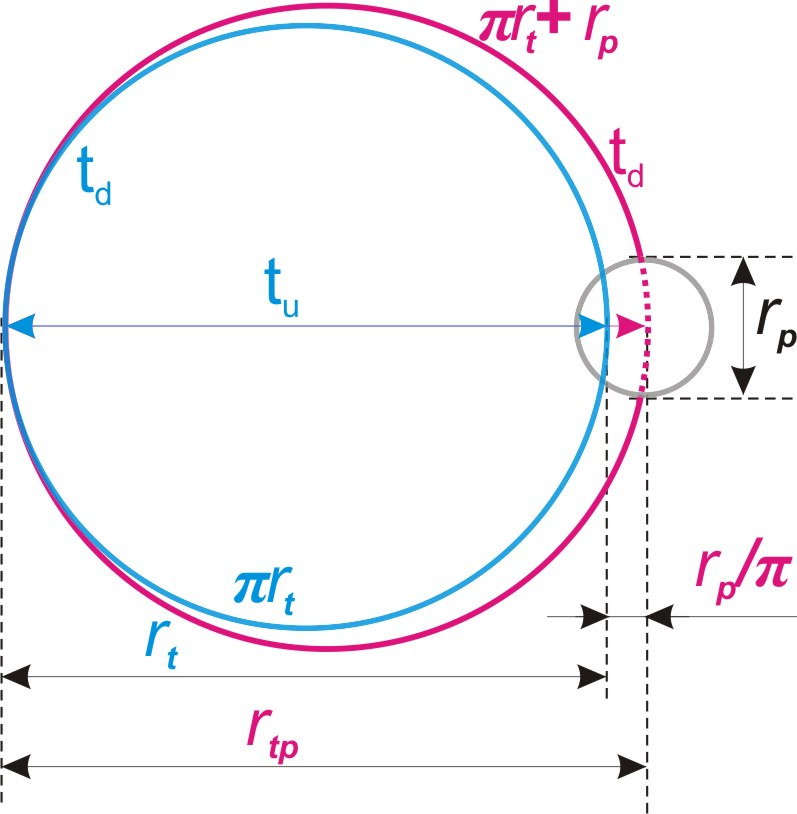Fig. 29.

rtp = rt + rp/π (50), where rtp – the size of the presence a proton.

Conclusion (c92):

In physical space, a proton is a spherical area of diameter rtp as a superposition of all possible positions of its physical body.

In contrast to a neutron, the size of presence area of a proton is not bound by the conditions of the cycle, and can change depending on external influences.

Conclusion (c93):

The size of presence area of a proton, and, consequently, its mass is not a constant value, but a statistical one.

Taking into account formula (09):

rp = rtnt/n

rtp = rt (1 + nt/nπ) (51)

Until the decay of a free neutron as a fundamental particle, its entire internal space was a superposition of all possible positions of the projection of the two-dimensional potential part of the elementary localization. It follows from the uncertainty condition of this space relative to the conditions of the physical space that it is not only a superposition of all alternative positions of the physical body of the particle, but also a superposition of all alternative positions of the projection of the boundary of the complete cycle of elementary localization.

After the decay of the neutron and the exit of the physical body beyond this space, it turns into an independent elementary particle. This follows from the fact that this space is determined by the conditions that singling out it in the main localization. Together with the condition of internal uncertainty, it corresponds to the определению (о40) of an elementary particle.

Taking into account the condition of reversion the direction of the projection of a definite dimension tu of the inner cycle of elementary localization (conclusion (c52)), the new particle is the area of presence of the boundary of the end of the cycle, projected onto the physical space (conclusion (c89)) with moving “outside - in”, fig. 30..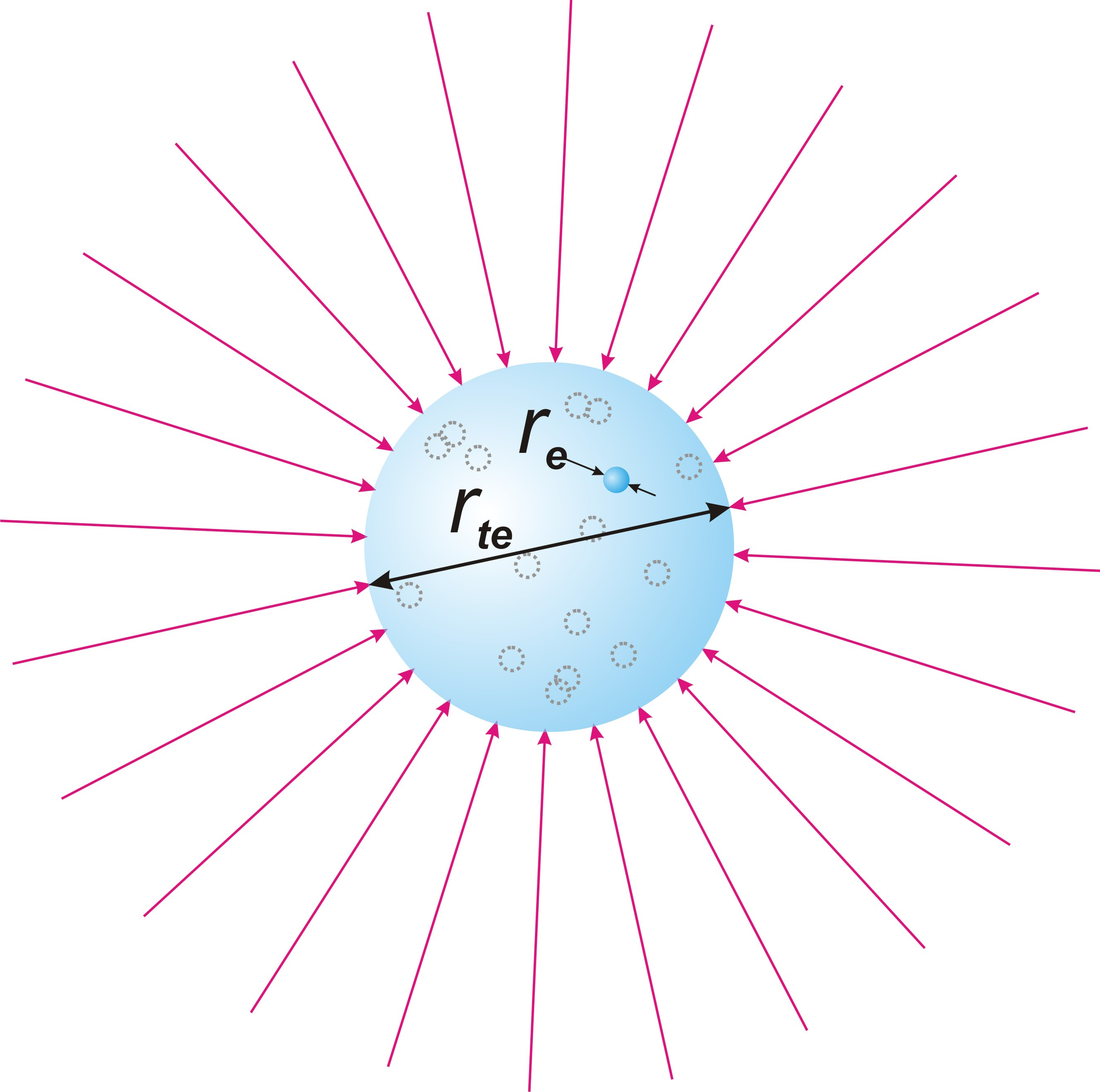Fig. 30.

The final state of elementary localization in the reverse cycle is the initial state in its forward cycle. The projection of the initial state of the cycle of elementary localization into the physical space is a fundamental particle in the first quantum of the state of the main cycle, at nt = 1.

In accordance with the logic of the decay of a free neutron, this second new elementary particle is an electron, and the size of the fundamental particle in the first quantum of the state of the main cycle can be considered the physical size (diameter) of the electron. Since in the first state quantum Rt = dr, we find the physical size of the electron:

re = dr/n = const (52), where re – the size of the physical body of an electron.

Conclusion (c94):

The physical body of an electron is a projection of the initial state of the inner cycle of elementary localization, at nt = 1.

Conclusion (c95):

The size of the physical body of the electron is unchanged throughout the cycle of the Universe localization.

Herewith the internal space of the physical body of the electron is indefinite with respect to the physical space.

Conclusion (c96):

An electron is an elementary particle and represents in the physical space the area of presence of its physical body.

The output of the physical body of the neutron from the projection of the internal cycle of elementary localization is projected into the physical space by the output from the area of uncertainty, which is a fundamental particle. Thus, from the moment of exit, the movement of the physical body must obey the conditions of movement in the physical space, that is, move along the mobile dimension Td. As a result, the entire path it has traveled along the internal indefinite dimension td is superimposed on the mobile dimension Td.

Conclusion (c97):

The path traversed by the physical body of the neutron within the cycle is projected onto the mobile dimension Td as a linear distance.

From the uncertainty condition of the physical body of a fundamental particle along the internal indefinite dimension td, it follows that it moves one-moment in its both opposite directions for the full length of the cycle corresponding in the internal space to a semicircle of size πdr. And since the physical body moves relative to the physical space in one direction, it follows that it travels the full path along the indefinite dimension td of the inner space one-moment in two directions, fig. 31.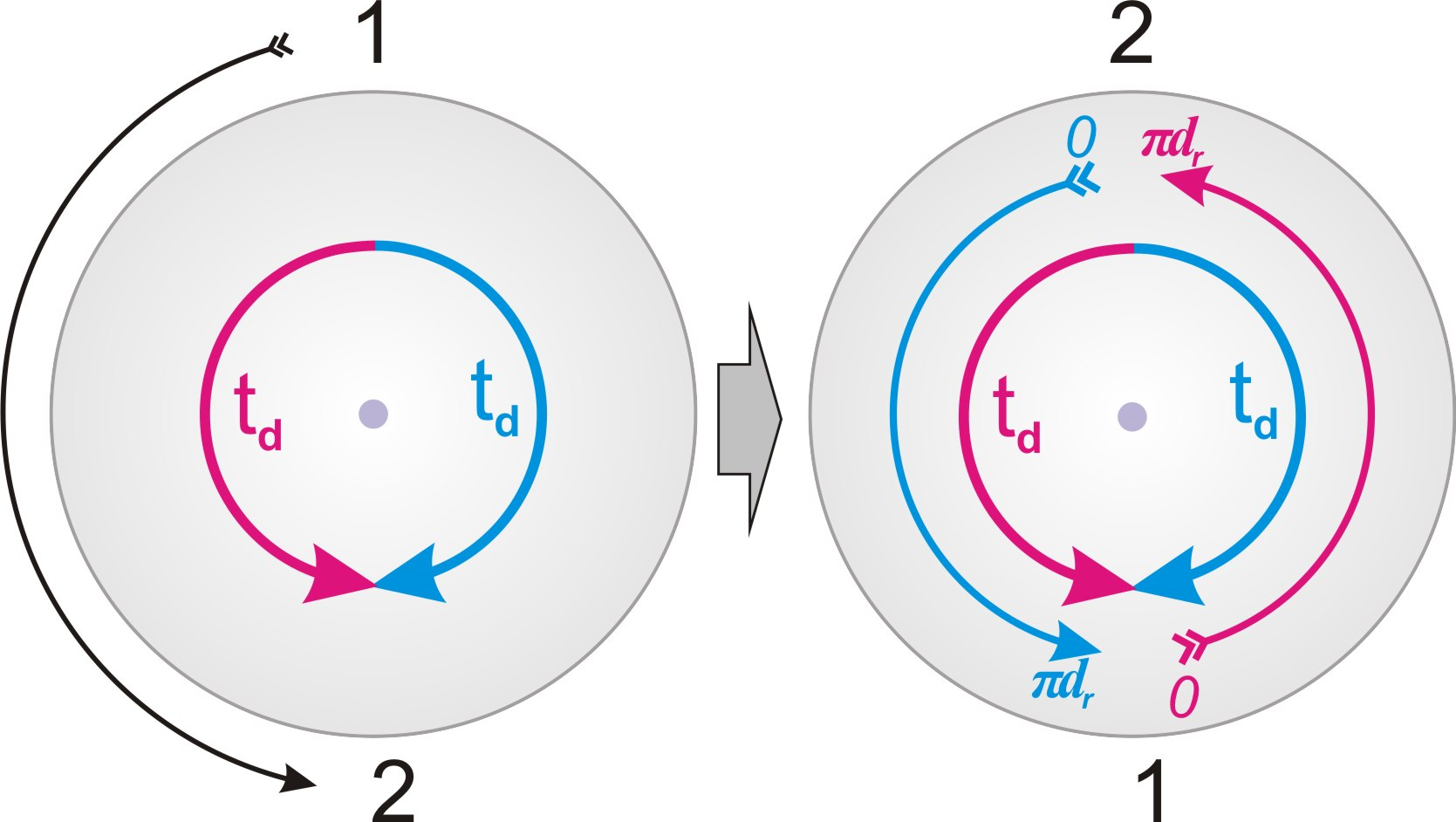Fig. 31.

Thus, the full size of the path traveled by the physical body is projected onto the mobile dimension Td of the physical space by two sizes πdr of the complete internal cycle, with a direction change point between them, fig. 32.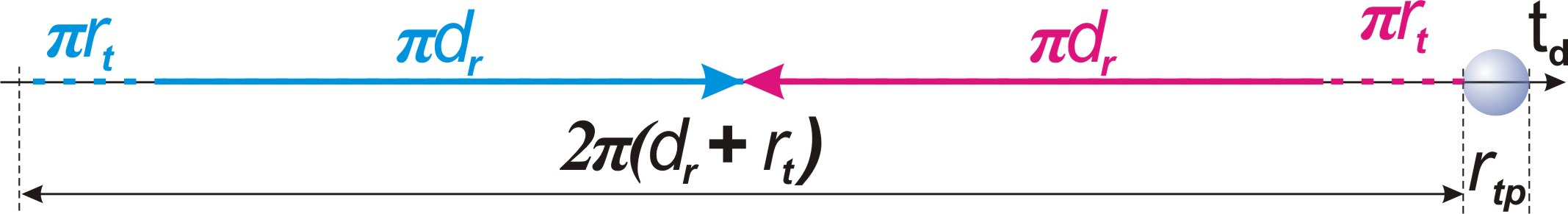Fig. 32.

This path is projected with an uncertainty equal to the size of presence πrt of the physical body along the indefinite dimension td in relation to the size of each of the two opposite cycles.

It should also take into account the size rtp of the uncertainty of the position of the physical body of the proton along the mobile dimension, relative to which the entire path is projected.

Thus, the total size 2πdr + 2πrt + rtp is the determining condition for the presence area of the electron at the moment of its formation as an independent elementary particle.

rtev = 2πdr + 2πrt + rtp = 2πdr + 2πrt + rt + rtnt/nπ (53), where rtev – the presence area of the electron at the moment of its formation along the mobile dimension Td.

The value of rtnt/nπ in this size is too small and can be neglected

Taking into account formula (07):

rtev = 2πdr + 2πrt + rt = 2πdr(1 + nt/n(1+1/2π)) (54)

In accordance with the conclusion (c59), the projection of the size of a fundamental particle onto the mobile dimension Td is equal to its size rt along the definite dimension Ru of the physical space. This conclusion is based on the fact that in the absence of motion of a fundamental particle, the mobile dimension Td and a definite dimension Ru are in a superposition of alternative variants of perpendicularity to each other, which has the shape of a ball in physical space.

By a similar logic, the area of presence of an electron is also formed, but the principle of size projection from one dimension to another is opposite. In the case of an electron, its size is determined by the projection of the movement along the inner cycle onto the mobile dimension Td in the physical space, and only then, this size rte is projected onto a definite dimension Ru of the physical space.

Conclusion (c98):

The diameter of the area of presence of an electron is determined by the projection of the full cycle of the material part of an elementary localization along its internal indefinite dimension td onto the mobile dimension Td

The increase in the size of the area of presence of an electron from rt to rtev cannot happen instantly. The maximum movement speed in physical space cannot exceed c (conclusion (c25)). At the same time, it is necessary to take into account the fact that the internal indefinite dimension td is projected onto the direct path along the mobile dimension Td by its turn, fig. 33.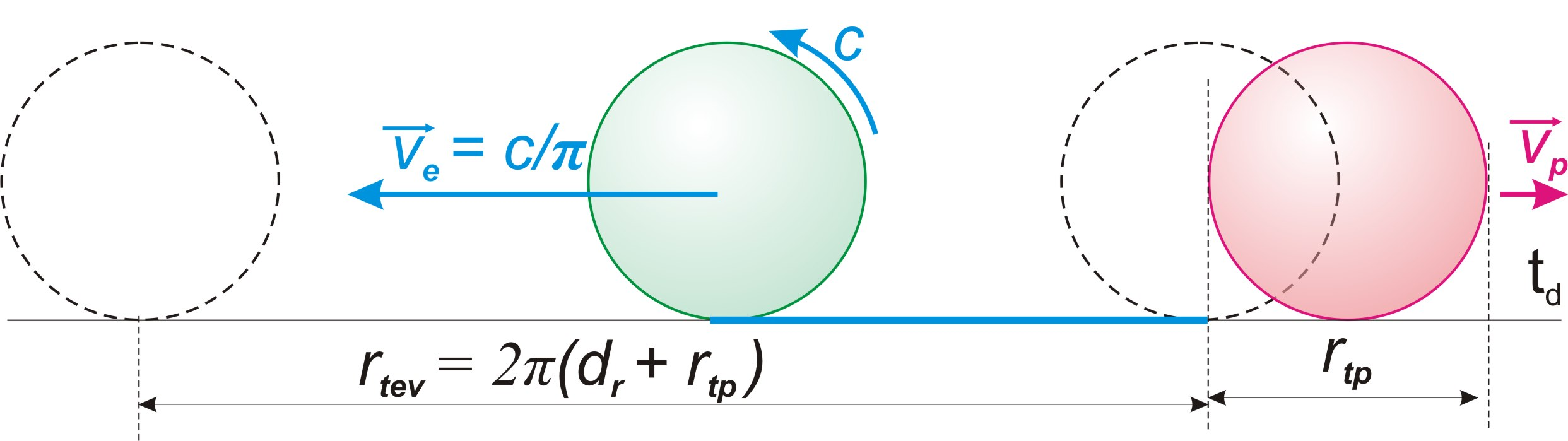Fig. 33.

The ratio of the mass of the proton to the mass of the electron is quite large, and the speed vp, which it acquires in the process of "repulsing" with the electron, can be neglected.

Thus, taking into account the maximum speed of movement c, an electron in the process of its growth acquires the speed c/π of its movement along the mobile dimension Td, in the spot reference frame associated with the Earth.

In accordance with formula (31), we find the size of presence of an electron at rest relative to the spot reference system associated with the Earth.

rte = rtev/(1-c2/π2c2) = rtev/(1-1/π2) (55), where rte – the size of presence of an electron in the conditions of the Earth.

In the process of neutron decay, when its physical body moves with acceleration along the internal indefinite dimension td, fig. 34, a), its movement in the inner cycle along the definite dimension tu is completely superimposed on it, and they change places, fig. 34, b)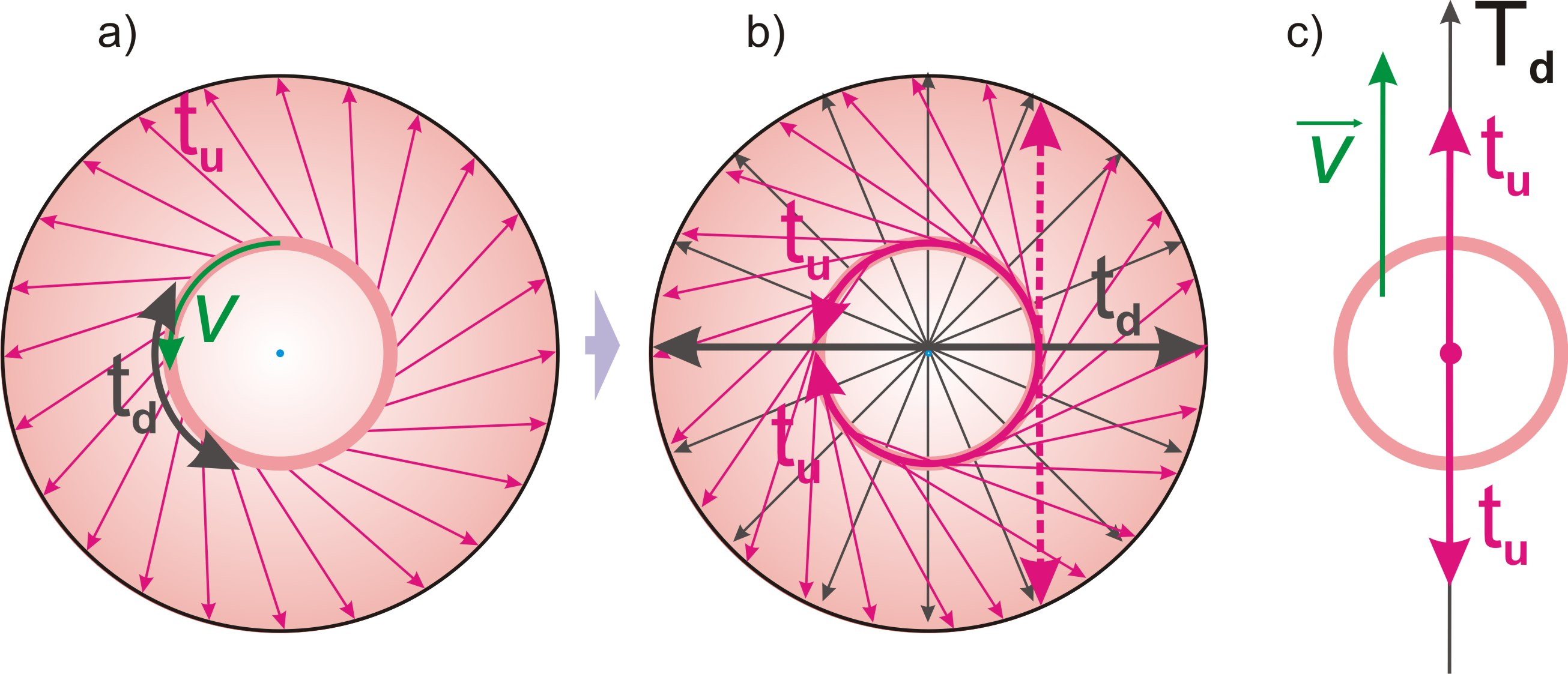Fig. 34.

Thus, when the physical body of the decayed fundamental particle enters the conditions of the physical space, the internal indefinite dimension of the elementary localization tu in relation to the electron and proton is projected onto the mobile dimension Td of the physical space, fig. 34, c).

This displacement of the internal definite dimension of the potential part tu to the place of its indefinite dimension td, when projected into the physical space, should be identically reflected in all other dimensions, as well as in the main parts themselves, fig. 35, a).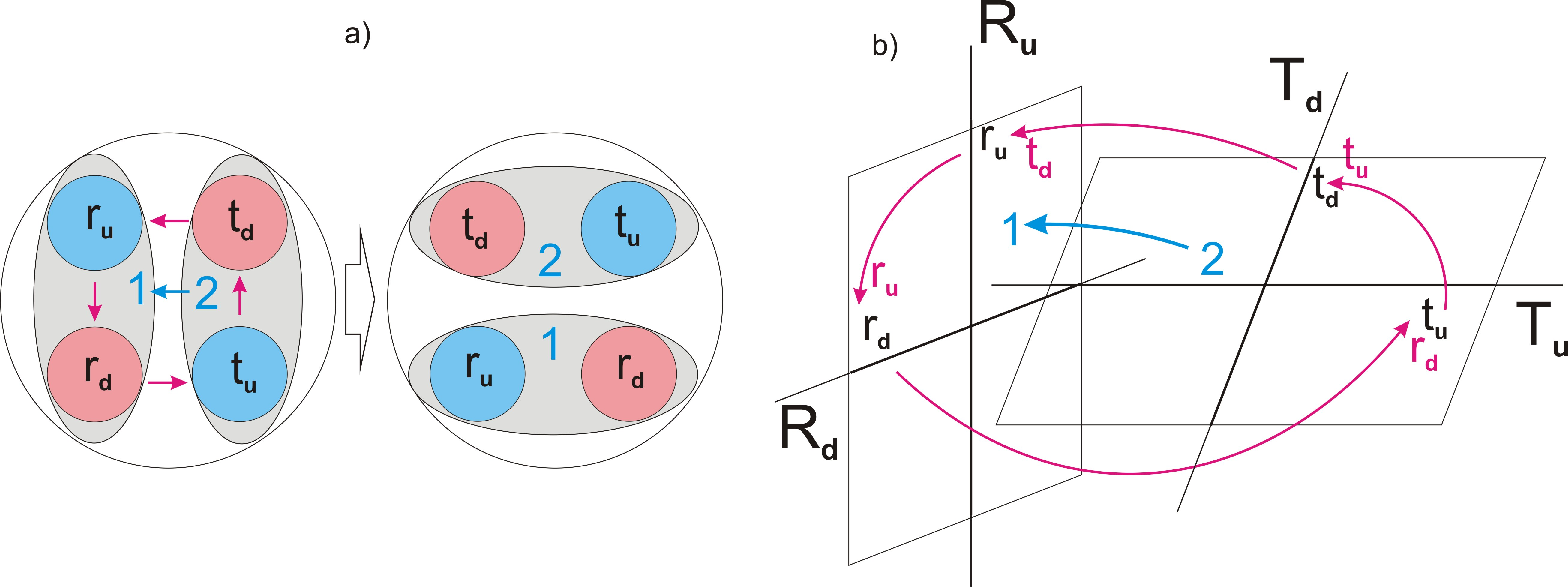Fig. 35.

As a result, when projecting the internal space of a fundamental particle into the physical space, all four of its dimensions are shifted by 90o.

Conclusion (c99):

As the result of the decay of a fundamental particle: the internal definite dimension of the potential part of an elementary localization tu is projected onto the mobile dimension Td; the indefinite dimension of the potential part of an elementary localization td is projected onto the definite dimension of the material part of the basic localization Ru; the definite dimension of the material part of an elementary localization ru is projected onto the indefinite dimension of the material part of the main localization Rd; the indefinite dimension of the material part of an elementary localization rd is projected onto the definite dimension of the potential part of the basic localization Tu.

Taking into account the change in the direction of the projection of the definite dimension tu of the inner cycle at the moment of neutron decay (conclusion (c88)), as well as the two-dimensionality of the potential part of elementary localization (conclusion (c7)), we conclude:

Conclusion (c100):

The presence area of an electron at rest in the frame of reference is a superposition of all possible positions of a flat circle, as a projection of the two-dimensional structure tutd of the potential part of an elementary localization, the diameter of which is the unfolded projection of two opposite directions of the internal definite dimension tu in such a way that they are always turned inwards, with a refraction of the direction to the opposite in the middle.

This situation with an electron at rest is shown in fig. 36, a).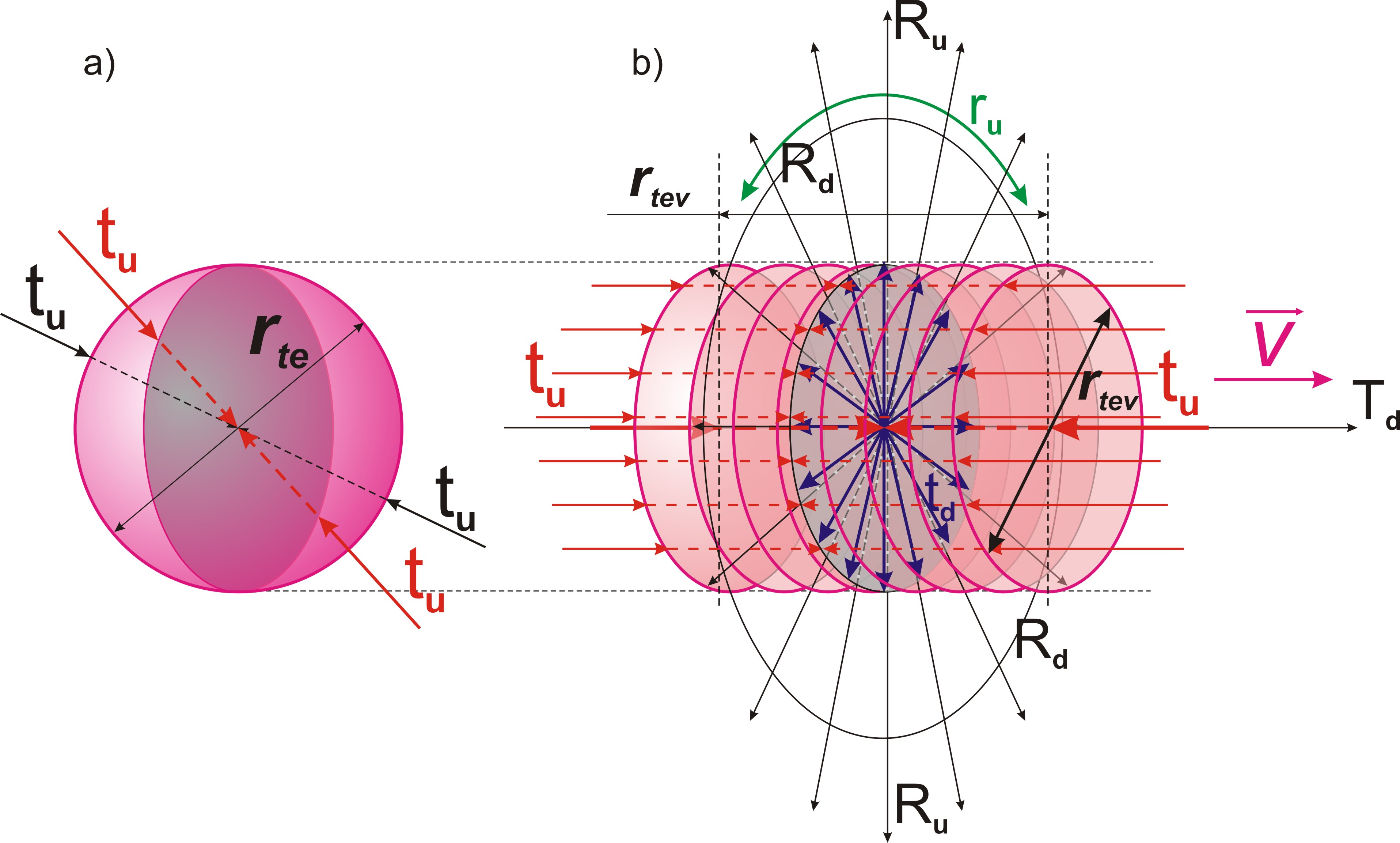Fig. 36.

When an electron moves, the mobile dimension Td, onto which the internal definite dimension tuof the potential part of the elementary localization is projected, is projected relative to it by a directed vector, fig. 36, b).

The definite dimension Ru of the material part of the main localization, onto which the internal indefinite dimension td of the potential part of an elementary localization is projected, is projected perpendicular to the motion vector and is a superposition of all its alternative positions.

Conclusion (c101):

The presence area of a moving electron is a cylinder of diameter and height rtev, the axis of which is directed along the motion vector, which is a superposition of all possible positions of the projection of the two-dimensional structure tutd of the potential part of an elementary localization, oriented along the mobile dimension Td and undefined in the other two dimensions Ru and Rd of the physical space.

18. Electrical forces

Content

From the conclusion (c89) made in the previous chapter, it follows that the projection of the force of the inner cycle of elementary localization into physical space determines the force of attraction between the proton and the electron.

Using the terms accepted in physics, we come to the following conclusions:

Conclusion (c102):

Electric forces represent a projection of the force of the inner cycle of elementary localization into the physical space.

Conclusion (c103):

An electric charge is an indivisible quantum of force, as a projection of the force of the internal cycle of one elementary localization into the physical space.

Since the electrical charge of a proton is traditionally considered positive, and that of an electron is negative, the conclusion follows:

Conclusion (c104):

The negative charge is determined by the direct projection of the direction of the mainstay dimension of elementary localization into the physical space, and the positive charge is determined by its inverse projection.

Since two elementary particles with the charges of the same sign are projections of internal cycles of two different elementary localizations, superimposed on the same physical space, a force must arise between them, tending to push their own cycles apart. Due to the indefiniteness of the mobile dimension, this repulsion of the projections of cycles is reflected by the repulsive force between two unlike charges along the mobile dimension Td.

From the principle of symmetry and identity (theorem (t2) and (т3)) it follows:

Conclusion (c105):

The repulsive force between two unlike charges is equal in magnitude to the attractive force between two like charges.

The force of the internal cycle of elementary localization is projected into the physical space by the force of the internal cycle of the fundamental particle.

To find the formula for the electrical interaction force, it is necessary to find the force of the internal cycle of the fundamental particle.

The increase in the size of the physical body of a fundamental particle in a quantum of state is determined by the ratio of its size rp to the number of passed quanta of state nt. Taking into account formula (09):

rp/nt = rt/n (56)

This value is a quantum of extension of elementary localization, or the size of the physical body of a fundamental particle along the internal definite dimension tu.

Let us find the acceleration of this process:

ap = rt/ndt2 (57), where ap - acceleration of the movement of the physical body of a fundamental particle along its internal cycle in the state quantum.

Taking into account formula (22):

fn = mpap = mprt/ndt2 (58), where fn – force of the inner cycle of a fundamental particle, mp - the mass of the physical body of a fundamental particle in its inner cycle.

The mass of the fundamental particle dm in relation to the main cycle is its material definiteness with respect to two dimensions Tu and Td of the potential part of the main localization, calculated relative to the corresponding projections of its size of presence. In essence, this is the mass of the physical body of the fundamental particle, but with the condition of uncertainty of its position relative to the area of diameter rt. Accordingly, with regard to the internal cycle, the mass of the physical body will be as many times larger as its size is less than the size of the internal potential space of the fundamental particle.

Along the projection of the internal indefinite dimension td of the potential part of an elementary localization, the physical body is not singled out, and, accordingly, its definiteness along this dimension is equal to 1.

Along the projection of the internal definite dimension tu of the potential part of an elementary localization, the size of the physical body of a fundamental particle is determined by the ratio of the size of presence of a fundamental particle rt to the determining integer n, fig. 37.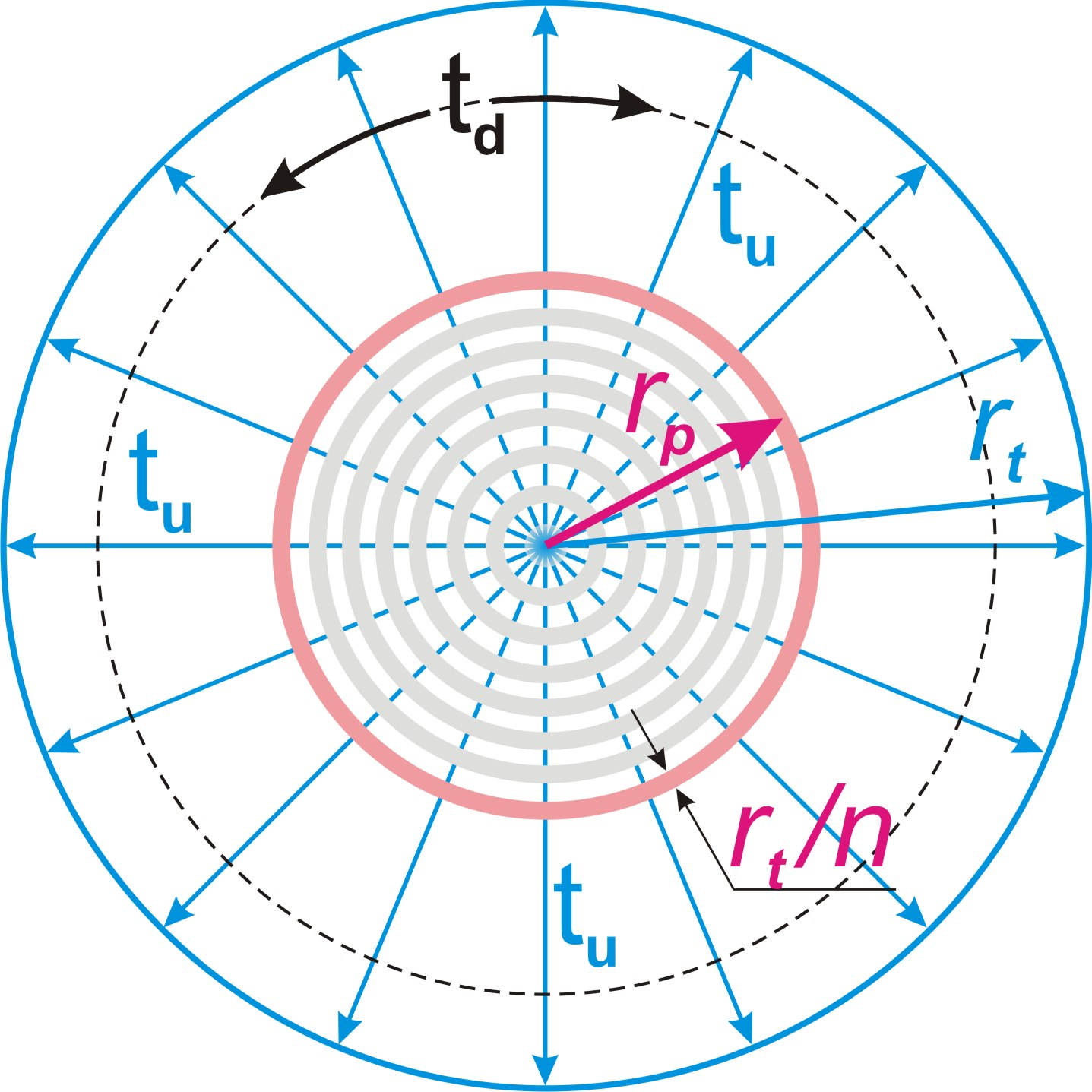Fig. 37.

Thus, we come to the conclusion:

Conclusion (c106):

The material definiteness of the physical body of an elementary particle in its internal cycle is n times greater than its material definiteness in relation to the main cycle.

Accordingly, the mass of the physical body of a fundamental particle in relation to the internal cycle, taking into account its motion relative to the ideal frame of reference, will be equal to dmvn. With this in mind, for the force of the inner cycle we get:

fn = mprt/ndt2= dmvnrt/ndt2 = dmvrt/dt2 (59)

We also apply the formula (07) and (02):

fn = dmvrt/dt2 = dmvdrnt/ndt2 = dmvc2nt/ndr (60)

It must be taken into account that the force acting along the internal definite dimension tu is projected onto the mobile dimension Td, which is indefinite. Similar to the conclusion (c82) as a result of this projection condition, the magnitude of the force is reduced by a factor of π.

fen = fn/π = dmvc2nt/πndr (61) , where fen – projection of the force of the internal cycle of a fundamental particle into the physical space.

According to the principle of identity with the gravitational cycle (глава15, definition (d39)), as a projection of the main cycle into physical space, this force will act in a complete electric cycle, when there will be a number of opposite charges on its poles, equal to the determining integer ne of the electric cycle.

The determining integer of the electric cycle should be determined similarly to the determining integer of the gravitational cycle according to the formula (34):

ne = R/rt, where R – distance between interacting charges.

Taking into account formula (07):

ne = R/rt = Rn/ntdr (62)

We get the formula for the force of interaction of two charges at a distance R:

fe0 = fen/ne2 = dmvc2nt/πndrne2 = dmvc2nt3dr/πn3R2 (63)

Since an increase in the charge on each side increases the interaction force accordingly, this force is proportional to the product of the number of unit charges:

fe = (q1/q0)(q2/q0)fe = q1q2fe/q02 = q1q2dmvc2nt3dr/πn3q02R2 (64), where q0 — unit charge or ratio of system of measures, q1 and q2 — interacting charges.

Compare with the formula of electrical interaction known in physics:

fe = Qq1q2/R2 , where Q — electrical constant.

We get the value of the electrical constant:

Q = dmvc2nt3dr/πn3q02 (65)

This formula contains a variable value of the number of state quanta passed by the Universe nt.

Conclusion (c107):

The electric constant grows in proportion to the cube of the number of state quanta nt passed by the Universe.

Considering that the number of opposite charges in the relativity of any spot reference frame is equal to the number of decayed fundamental particles, we come to the following conclusion:

Conclusion (c108):

In the relativity of any spot reference system, the number of positive charges is equal to the number of negative ones.

Let us pay attention to the fact that by dividing formula (65) of the electric constant by formula (41) of the gravitational constant, we obtain a formula for the number of state quanta nt passed by our Universe:

Q/G = dmvdmnt/q02 (66)

nt = Qq02/Gdmvdm (67)

If we neglect the difference between the mass of the neutron dmv in the reference frame associated with the Earth and its mass at rest dm, then we can easily find the value for nt as the ratio of the electrical interaction force of two unit charges to the force of the gravitational interaction of two neutrons. We get the value:

nt ≈1,2•1036

The degree of the resulting number leaves no doubt that the determining integer of the localization of our Universe is:

n = 2128 = 3,40•1038 (68)

It was this discovery (only the mass of the proton was used instead of the mass of the neutron) that prompted the author the direction of all further research. As a result, much more accurate values were obtained, including the speed of the Earth's movement in the Universe, which results in the difference between the neutron mass dmv and its theoretically calculated mass in the ideal reference frame dm. Therefore, we will not rush with calculations, and first we will find several more important formulas that will allow us to obtain the theoretical value of the neutron mass.

19. Hydrogen atom

Content

The movement of an electron and a proton under the action of the electric force is carried out along the mobile dimension Td.

The mass of an electron is much less than the mass of a proton, and the electron acquires the main momentum of mutual motion.

By the time of the collision, the momentum of the electron is large enough, and it continues to move so that the proton passes through its area of presence. In this case, the proton from the moment of penetration into area of presence of the electron is in the conditions of two spaces: the physical and internal space of the electron.

When the proton, moving towards the boundary of its cycle, passes a distance in the internal space of the electron exceeding πdr, the projection of the internal definite dimension tu changes its direction to the opposite one. Thus, oscillatory motions of the area of presence of the electron relative to the proton arise, creating a stable state of hydrogen atom, fig. 38, a).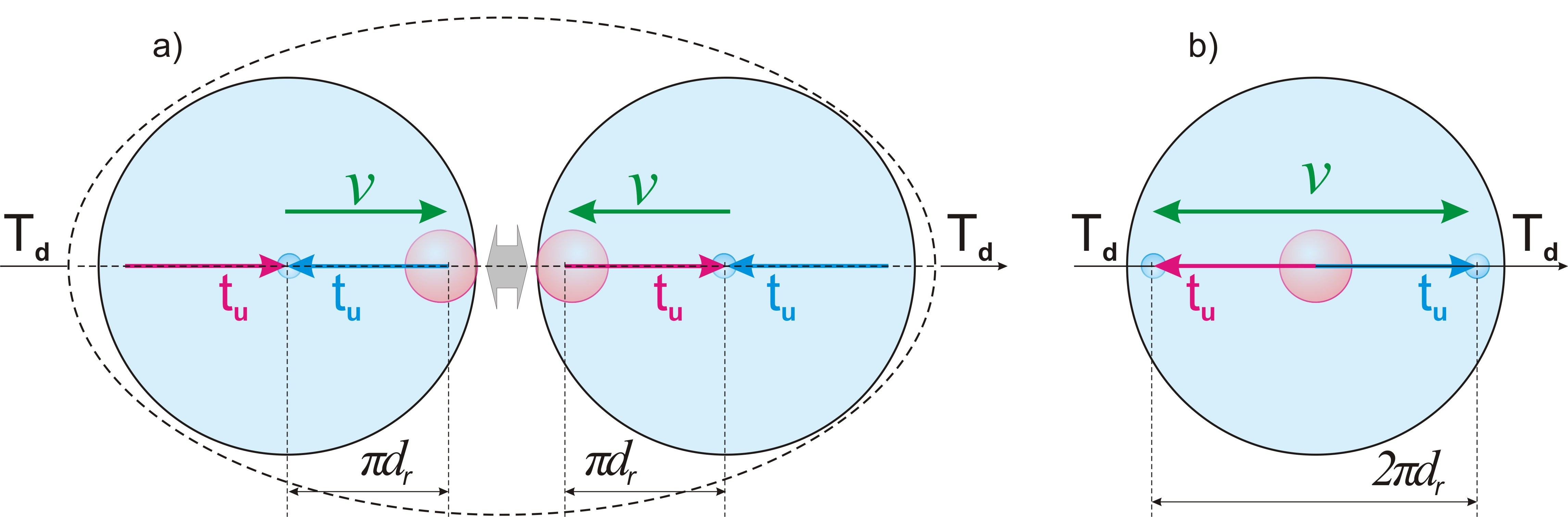Fig. 38.

Conclusion (c109):

The hydrogen atom is a stable process of oscillations of the projection of the boundary of cycle of elementary localization relative to the proton in the inner space of the area of presence of the electron.

This process in the internal space of the electron is projected into the physical space by the indefiniteness of the position of the area of presence of the electron relative to the proton, fig. 38, b).

Thus, there are no oscillations of opposite charges relative to the physical space inside the hydrogen atom.

Conclusion (c110):

The oscillatory interaction of the electron and the proton occurs relative to the inner space of the area of presence of the electron, and relative to the physical space, the hydrogen atom has no vibrations and moves as an integral physical object.

Conclusion (c111):

The size of the hydrogen atom is determined by the size of the area of presence of the electron, which, in turn, is determined within its minimum value of the two sizes of the indefinite dimension td of the full cycle of elementary localization, equal to 2πdr.

The comparative increase in this size due to the loss of energy by the electron is insignificant. Thus, we must come to an important conclusion:

Conclusion (c112):

The sizes of atoms during the cycle of the Universe remain unchanged, while the size of the Universe increases by n = 2128 times.

From this conclusion it follows that the sizes of solid cosmic bodies, such as our planet, change slightly in the course of the cycle of the Universe, and the cosmic distances between them increase. Thus, taking into account the conclusion (c84), we arrive at the following conclusion:

Conclusion (c113):

The acceleration of free fall on the surface of the planet increases in proportion to the square of the state quanta passed by the Universe.

This increase in the forces squeezing the planet is perhaps one of the main reasons for the internal heating of the planet.

20. Photon and Planck's constant

Content

If the oscillatory motion of the area of presence of an electron in one direction relative to a proton exceeds the size 2πdr of the deployed projection of two internal cycles of elementary localization along its indefinite dimension td, the electron will be forced to move to a new cycle with a change in the direction of the projection of the internal definite dimension tu of elementary localization.

Taking into account that the transition to a new cycle is carried out along the deployed projection of two opposite cycles of the indefinite dimension td, the projection of the definite dimension tu must change its direction twice.

As a result of this double change in the direction of the projection of the definite dimension tu, an electron returns to its original state of projection into physical space.

In the relativity of physical space, this double cycle along the projection of the internal indefinite dimension td is carried out along the definite dimension Ru of the physical space onto which it is projected.

The projections of successive cycles of the same elementary localization cannot overlap each other. This condition necessarily follows from the principle of complete internal closure of the cycle. As a result of these conditions, the completed double cycle must move away from the new cycle at the maximum possible speed c.

At the maximum speed of movement c, the quantum of extension of the physical process (definition (d30)) of the overlap of the previous and subsequent cycles is invariably equal to the distance separating them. In accordance with the conclusion (c68), their spatial relationships remain indefinite, and they are in a superposition of two alternative options - a projection into space of one of them in the absence of a projection of the other.

It follows from this that this double cycle is a singled out identical part of the material part of the main localization and is a material particle, fig. 39, a).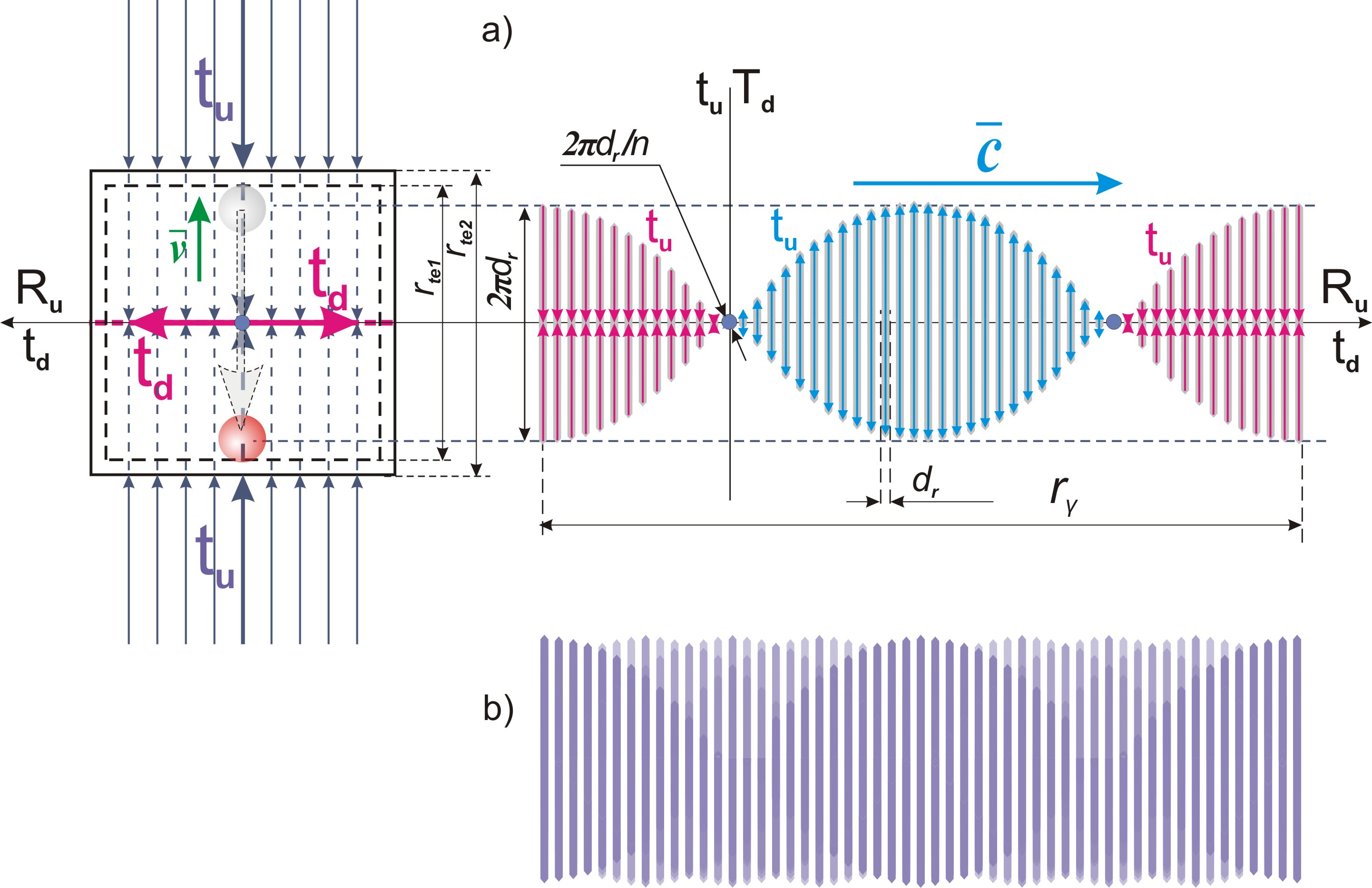Fig. 39.

The same conclusion about the motion of a new particle with speed c follows from the identity of its motion along the definite dimension Ru of the physical space, the motion of a fundamental particle along the definite (mainstay) dimension Tu.

It is easy to guess that in this case we are dealing with a photon.

As a result of the emission of a photon, the mass of the electron decreases, and its size of presence increases with rte1 and rte2. This difference in the size of presence determines the difference in the masses of the electron before and after the emission of a photon and is equal to the energy of the separated particle.

Conclusion (c114):

As a result of the emission of a photon, the size of presence of the electron increases, and the mass decreases.

The entire secession process of a photon is projected onto the definite dimension Ru of size rγ, which, by definition (d30), is a quantum of extension of a physical process, or, as it is commonly called, a quantum of light.

In accordance with the conclusion (c68), the sequence of parts of the process within the quantum of extension rγ is indefinite, fig. 39, b).

The size of quantum of extension of a photon ry, traditionally in physics is called its wavelength.

rγ = сd (69), where d – the size of a quantum of extension of the process in units of time.

Conclusion (c115):

The process of secession of a photon from an electron is one-moment and is a superposition of all alternative sequences of its parts.

This means that the inner space of a photon is indefinite, and corresponds to the определению (о40) of an elementary particle.

Conclusion (c116):

A photon is an elementary particle that separates from an electron as a result of the displacement of the projection of the potential part of the elementary localization by two successive cycles along its indefinite dimension td.

Conclusion (c117):

The movement of a photon is carried out along a definite dimension Ru of the physical space with the maximum possible speed c.

In accordance with the conclusion (c101), the area of presence of an electron in motion is a superposition of alternative positions of the projection of the two-dimensional structure of the potential part of the elementary localization tutd onto two dimensions of physical space: the mobile Td and the definite Ru, fig. 40, a).

The process of secession of photon occurs in one randomly chosen position of this two-dimensional structure, Fig. 40, b).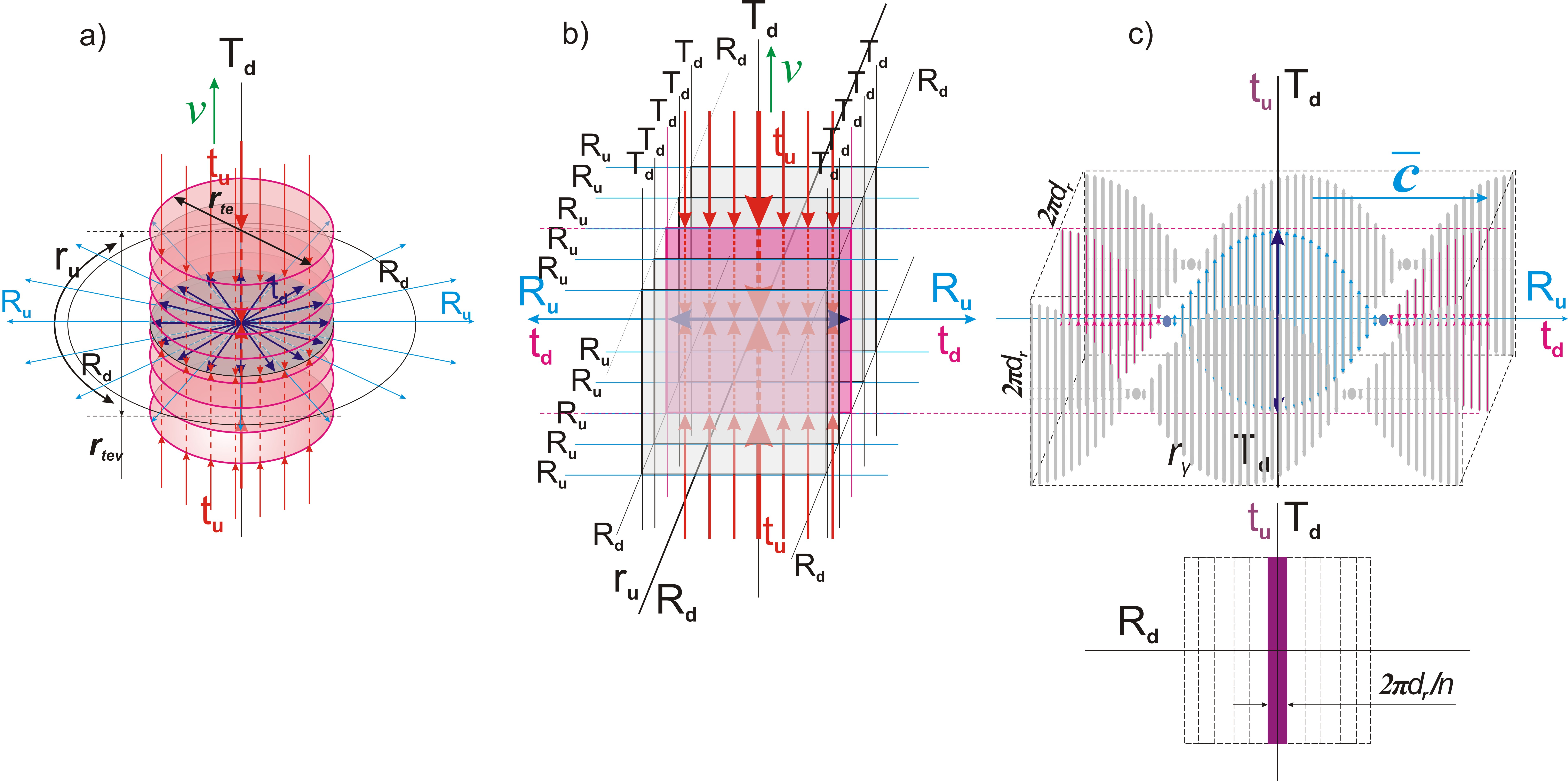Fig. 40.

Conclusion (c118):

The direction of the mobile dimension Td in relation to a photon, as a projection of the two-dimensional structure of the potential part of an elementary localization, has a strict vector orientation.

Taking into account the indefiniteness of the position of this two-dimensional structure along the indefinite dimension of the physical space Rd of size 2πdr, we conclude:

Conclusion (c119):

A photon, as a strictly oriented two-dimensional structure along the definite Ru and the mobile Td dimensions of physical space, along its third indefinite dimension Rd is a superposition of its alternative positions of size 2πdr.

In each of these alternative positions, the size of the photon along the mobile dimension Td is determined by the minimum size of the state quantum of the inner cycle of the photon. This minimum size is a projection of the physical body of the electron and is determined by its physical size 2πdr/n.

Conclusion (c120):

The physical size of a photon along the mobile dimension is 2πdr/n, and the size of its area of presence along this dimension is 2πdr.

The orientation of a photon in physical space determines its polarization property, fig. 40, c).

Conclusion (c121):

The polarization of a photon is determined by the direction of the projection of the mobile dimension Td relative to it.

All spot reference systems in their relativity are in the center of the physical space, relative to which its definite dimension Ru is projected, along which the photon moves with the maximum possible speed c. Mutual movement of spot reference frames occurs along the mobile dimension Td, which is indefinite. This explains the independence of the movement of photons from the movement of physical bodies - they move along different, perpendicular to each other dimensions.

Conclusion (c122):

A photon moves with the maximum possible speed c along the definite dimension Ru of the physical space relative to any spot frame of reference, regardless of the speed of its movement.

In accordance with the definition (d36), the material definiteness of fundamental particles and their parts, the cycle of which is carried out along the mainstay dimension, is determined relative to the two-dimensional structure TuTd of the potential part of the main localization, along which they are displaced in state quanta.

The displacement of a photon in the state quanta, in accordance with the conclusion (c119), occurs across the two-dimensional structure of the mobile Td and definite Ru dimensions of the physical space. Thus, according to the principle of identity (theorem (t2)), the material definiteness of a photon must determining with respect to these two dimensions.

The size of a photon along the definite dimension of the physical space Ru is equal to rγ, and its definiteness along this dimension in accordance with the definition (d35) is equal to Rt/rγ.

According to the second mobile dimension Td, the size of a photon is determined by the deployed projection of two opposite cycles of elementary localization along its internal indefinite dimension td of the potential part and is equal to 2πdr. Accordingly, for each of the two opposite directions of this dimension, the photon is determined by the size πdr.

Taking into account the size πRt of the mobile dimension Td, the definiteness of a photon along it is equal to πRt/πdr.

On the basis of the definition (d36) we find the material definiteness of a photon:

uγ = (πRt/πdr)(Rt/rγ) = Rt2/drrγ (70), where uγ – the material definiteness of a photon.

Taking into account formula (06):

uγ = Rt2/drrγ = nt2dr/rγ (71)

Let us find the mass of a photon using the formula (21):

mγ = kuγ, where mγ – the mass of a photon.

Taking into account formula (25):

k = dm/n2

mγ = kuγ = knt2dr/rγ = dmnt2dr/n2rγ (72)

The frequency of a photon in physics is the value of the ratio of the speed of its movement c to the length of its wave rγ.

γ = c/rγ = 1/d (73) , where γ – the frequency of a photon.

The size of a photon does not depend on the stage of the main cycle. Accordingly, the frequency of the photon remains unchanged.

Conclusion (c123):

The frequency and size of a photon do not depend on the number of passed state quanta and do not change during the main cycle.

The energy of a photon should be determined by the work of the force of the symmetry restoration cycle in its quantum of state when moving along the definite dimension Ru:

eγ = mγaγrγ (74), where aγ – acceleration of gain the speed c by a photon in its quantum of state.

aγ = c/d

Taking into account formula (69):

rγ = cd

eγ = mγaγrγ = mγc2 (75)

Taking into account formula (72):

eγ = mγc2 = dmc2nt2dr/n2rγ (76)

Let us replace the wavelength in it with the frequency:

rγ = c/γ (77)

eγ = dmc2nt2dr/n2rγ = γdmcnt2dr/n2 (78)

Comparing this formula with the formula accepted in physics:

eγ = hγ, where h - the Planck's constant.

We get:

h = dmcnt2dr/n2 (79)

Conclusion (c124):

The value of Planck's constant and the energy of a photon grow in proportion to the square of the passed state quanta.

21. The determining integer of the Universe and the mass of the fundamental particle

Content

Finally, we have obtained all the formulas we need, which make it possible, on the basis of experimental data of some physical characteristics of our physical world, to obtain the theoretical value of its other main characteristics and compare with their experimental value. This will allow not only to prove the correctness of our theoretical model, but also to obtain some completely new for science exact data on the physical characteristics of our Universe.

We divide (79) of the Planck constant by (41) of the gravitational constant:

h = dmcnt2dr/n2

G = c2drnt2/πn3dm

h/G = dm2πn/c (80)

dm2 = hc/Gπn (81)

dm =(hc/Gπn) (82)

All components of this formula are known to us, taking into account the conclusion already made in chapter 18 and formula (68) regarding the value of the determining integer of localization of our Universe:

n = 2128

G = 6,67384(80)•10-11 m3/kgs2

c = 299 792 458 м/с

h = 6,62606957(29)•10-34 Js

We get the value of the unit of mass:

dm =(hc/Gπn) = 1,66861•10-27 kg (83)

Experimentally obtained neutron mass:

dmv = 1,674927351(74)•10-27 kg

Such a coincidence with a discrepancy of only 0,4% leaves no doubt that our Universe is an eighth-order localization with a determining integer n equal to 2128. It makes no sense to carry out calculations for other orders of localizations, since the difference will be dozens of orders of magnitude.

Conclusion (c125):

Our Universe is an eighth-order localization with a determining integer n = 2128.

22. The exact value of the speed of the Earth in the Universe

Content

The small difference between the mass unit dm and the mass of the neutron dmv is explained by the movement of the spot reference frame associated with our planet. With the help of the formula (30) obtained by us earlier for increasing the mass of a fundamental particle (neutron) in a spot frame of reference moving with a speed vg relative to the ideal frame of reference, we can find the exact value of this speed of movement of our planet in outer space.

dmv = dm/(1-vg2/c2)

1- vg2/c2 = (dm/dmv)2

vg2/c2 =1- (dm/dmv)2

vg2 =(1- (dm/dmv)2)c2

vg = c(1- (dm/dmv)2)= 26 013 292 m/s (100)

Conclusion (c126):

The spot frame of reference associated with the planet Earth is moving relative to the ideal frame of reference with a speed 26 013 292 m/s.

According to the logic of the process, this speed should be determined by the movement of the Earth around the center of the galaxy. But the space observations and calculations accumulated to date do not show such a high speed. The problem lies in the calculation method, which does not take into account the distortion of the projection of cosmic processes at long distances into the conditions of the spot reference system associated with the Earth conclusion (c58-1). The same applies to the conclusions about the existence of "dark matter" and "dark energy". The fact that the found speed value corresponds to reality will be shown by further calculation on its basis of the decay time of a free neutron, which exactly coincides with the experimental one.

23. The number of quanta of state passed by the Universe

Content

As we noted in главе 18, formula (41) for the gravitational constant and formula (65) for the electric constant obtained by us allow us to find the exact value of nt, the number of state quanta passed by our Universe.

G = c2drnt2/πn3dm

Q = dmvc2nt3dr/πn3q02

Let us divide these formulas into each other:

Q/G = dmdmvnt/q02 (85)

nt = Qq02/Gdmdmv (86)

Let us substitute the known table values:

Q = 8,9875517873681764•109 Nm2/C2

q0 = 1,602 176 565(35)•10-19 C

G = 6,67384(80)•10-11 m3/kgs2

dmv = 1,674927351(74)•10-27 kg

And the value of the unit of mass found by us (83):

dm = 1,66861•10-27 kg

We get:

nt = 1,23690•1036 (88)

Let us find the coefficient of the ratio of the full cycle to the passed part:

kt = n/nt = 275,1084 (89)

Conclusion (c127):

The localization cycle of our Universe has passed 1/275 of its full size.

24. Exact characteristics of the neutron and quantum of state

Content

Now we have all the necessary values to calculate the size of presence rt of a neutron and its physical size rp, as well as the size of the quantum of extension in units of length dr and units of time dt.

From formula (41) we find the size dr:

G = c2drnt2/πn3dm

dr = Gπn3dm/c2nt2 = 1,0025089•10-10 m (90)

dt = dr/c = 3,3440099•10-19 s (91)

Using formulas (07) and (09) we find the sizes rt and rp:

rt = ntdr/n = Gπn2dm/c2nt = 3,644042•10-13 m (92)

rp = nt2dr/n2 = Gπndm/c2 = 1,324581•10-15 m (93)

Experimentally obtained the approximate physical size (diameter) of the neutron (rp) - 1,6•10-15 m.

25. The exact physical characteristics of the Universe

Content

Based on the obtained values, we calculate the main characteristics of the Universe and compare them with those known to science.

Let us find the lifetime of the Universe:

T = ntdt = 4,13620•1017 s = 1,31•1010 years, or 13,1 milliard years. (94)

As we can see, this result is in full agreement with modern scientific data.

Let us find the radius of the Universe in accordance with formula (06):

Rt = ntdr = 1,244695•1026 m (95)

26. Calculation of the decay time of a free neutron (beta decay)

Content

Using the formula (49) we obtained earlier, we calculate the decay time of a free neutron:

tn = ndr(2π/cntvg)

We know all the components of this formula and we can calculate this time:

tn= 870,647 s (96)

The experimentally determined decay rate of a free neutron is 880 and 865 seconds under different experimental conditions. The result obtained does not need comments.

27. Calculation of the mass of an electron and a proton

Content

The size of all elementary particles along the mainstay dimension Tu is equal to the quantum of extension dr. Thus, the material definiteness (definition (d36)), and, accordingly, the mass of elementary particles having a rest mass, in accordance with the definition (d35), should be inversely proportional to their the size of presence.

mi0 = dmrt/rti (97), where mi0 – the mass of an elementary particle at rest, rti – the size of presence of an elementary particle.

Taking into account the speed of the Earth:

mi = dmvrt/rti (98), where mi – the mass of an elementary particle resting in a reference frame associated with the Earth.

In accordance with формулами (55) and (54), we find the size of presence an electron.

rte = 2πdr(1 + nt/n(1+1/2π)) /(1-1/π2)

rte = 6,66539•10-10 (99)

Find the mass of an electron:

me = dmvrt/rte = 9,15701•10-31kg (100)

The experimentally known electron mass is considered to be: 9,10938291(40)•10-31kg. The difference of 0,52% is another clear confirmation of this theory.

In accordance with formula (51), we find the size of presence a proton.

rtp = rt (1 + nt/nπ)

rtp = 3,64825847•10-13 (101)

Find the mass of a proton:

mp = dmvrt/rtp = 1,6729916•10−27 kg (102)

Experimentally known proton mass: 1,672621777(74)•10−27 kg

The difference is 0,02% between the calculated mass and the experimental one, and 16% between the experimental neutron and proton mass difference and its theoretical value. Of course, the second is more important, but this discrepancy of 16%, nevertheless, is a good result. It is possible that the proton loses energy during interactions and the formation of atoms, and its size of presence increases, which does not contradict this theory (conclusion (c93)).

28. Antineutrino and neutrino

Content

The third particle of beta-decay of a free neutron (conclusion (c85)) is an antineutrino, which is a projection of the full cycle of elementary localization along the internal indefinite dimension td onto the definite dimension Ru of the physical space.

As a result of this complete cycle along the projection of the internal indefinite dimension td, the direction of the projection of the internal definite dimension tu changes from the orientation from “inside-out” to the opposite - “outside-in”.

Similar to a photon (conclusion (c117)) antineutrino should move with the maximum possible speed c along the definite dimension Ru of the physical space, fig. 41, a).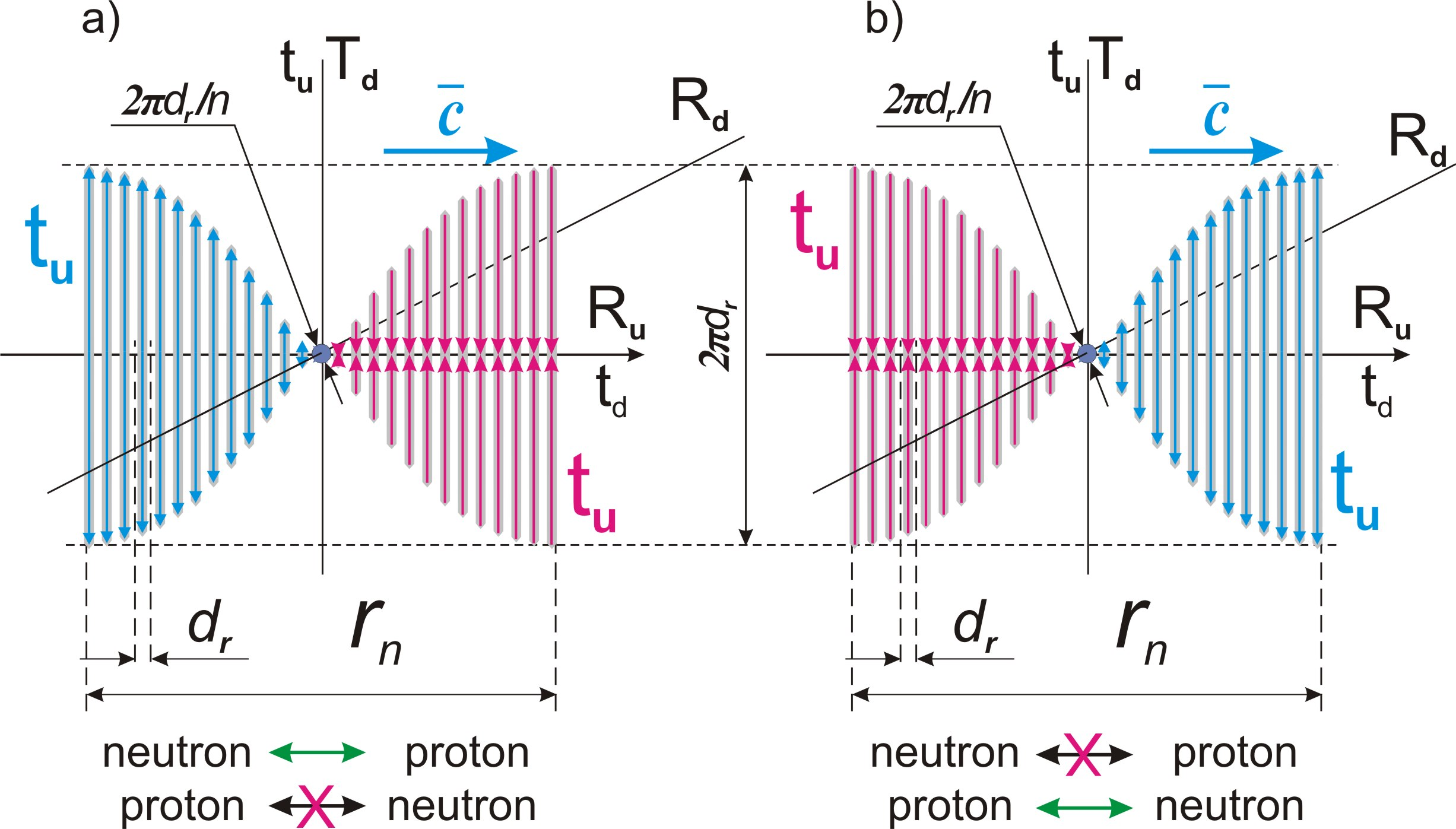Fig. 41.

Conclusion (c128):

Unlike a photon, which is a projection of a double full cycle of elementary localization along its internal indefinite dimension td, onto the definite dimension Ru of physical space, an antineutrino is a projection of a similar single cycle.

Conclusion (c129):

The antineutrino moves with the maximum possible speed c along a definite dimension Ru of the physical space relative to any spot reference frame, regardless of its speed of movement

Since the antineutrino cycle is a change in the orientation of the projection of the definite dimension tu of the cycle of elementary localization from “inside-out” to “outside-in”, its next cycle in the reverse interaction with other particles should be a change in the orientation of the projection of dimension tu from “outside-in” to “inside-out”.

It follows from this condition that the antineutrino can react only with a proton and an electron, the direction of projection of the dimension tu relative to which has the corresponding orientation - “outside-in”. And it cannot react with a neutron (also with a positron), the direction of projection of the dimensiontu relative to which is opposite.

From the principle of symmetry (theorem (t3)) it follows the possibility of the existence of a particle opposite to the antineutrino, the cycle of which is a change in the orientation of the projection of the definite dimension tu of the cycle of an elementary localization from “outside-in” to “inside-out”. This particle is a neutrino, fig. 41, b).

Conclusion (c130):

The neutrino is a projection of the full cycle of an elementary localization into the physical space with a change in the direction of the projection of the definite dimension tu of its potential part from “outside-in” to “inside-out”, and the antineutrino is a similar projection of the full cycle with the opposite change of this direction from “inside-out” to “outside - in”.

Thus, the next cycle for the neutrino should be a change in the orientation of projection of the dimension tu from “inside-out” to “outside-in”, which determines the logic of its reactions with other particles.

Conclusion (c131):

An antineutrino can react with a proton and an electron and cannot react with a neutron and a positron, while a neutrino, on the contrary, can react with a neutron and a positron, and cannot react with a proton and an electron.

This theoretical conclusion is in strict accordance with modern experimental data.

In addition to what has been said, we must write down one more important conclusion that draws a line under the unfinished disputes in physics regarding the speed of the neutrino and whether it has a rest mass:

Conclusion (c132):

Neutrinos and antineutrinos move in physical space with the maximum possible speed c relative to any spot reference frame, and the concept of rest mass in relation to them has no physical meaning.

29. Magnetic forces

Content

As a result of neutron decay, in accordance with the conclusion (c89), the projections of cycles of different elementary localizations are superimposed on each other in physical space. Thus, with respect to each charged particle, all other charged particles of the same sign with it are inside the projection of its cycle.

In this situation, there is a mutual disturbance of symmetry by charges of the same sign relative to each other.

In accordance with the conclusion (c99) regarding charges, the internal definite dimension tu of an elementary localization is projected onto the mobile dimension Td, and the indefinite td onto the definite dimension of the physical space Ru. Thus, identically to the process of gravity passing through the mobile dimension Td, the process of mutual symmetry breaking by charges occurs along the definite dimension of the physical space Ru.

As a consequence of this symmetry disturbance, another type of symmetry restoration cycles arises that is the magnetic. Since we are talking about the projection of the internal cycle of elementary localization, then the force in this new type of cycles is an identical projection of the electrical interaction force.

Conclusion (c133):

The magnetic interaction is a consequence of mutual symmetry breaking by electric charges of the same sign and is an identical projection of the electric interaction force.

The magnetic force vector must coincide with the direction of the definite dimension Ru and be perpendicular to the mobile dimension Td of the physical space relative to the charged particle.

In the case of parallel motion of like charges, the direction of the definite dimension Ru of the physical space relative to both charges coincides with the line connecting them, fig. 42, a).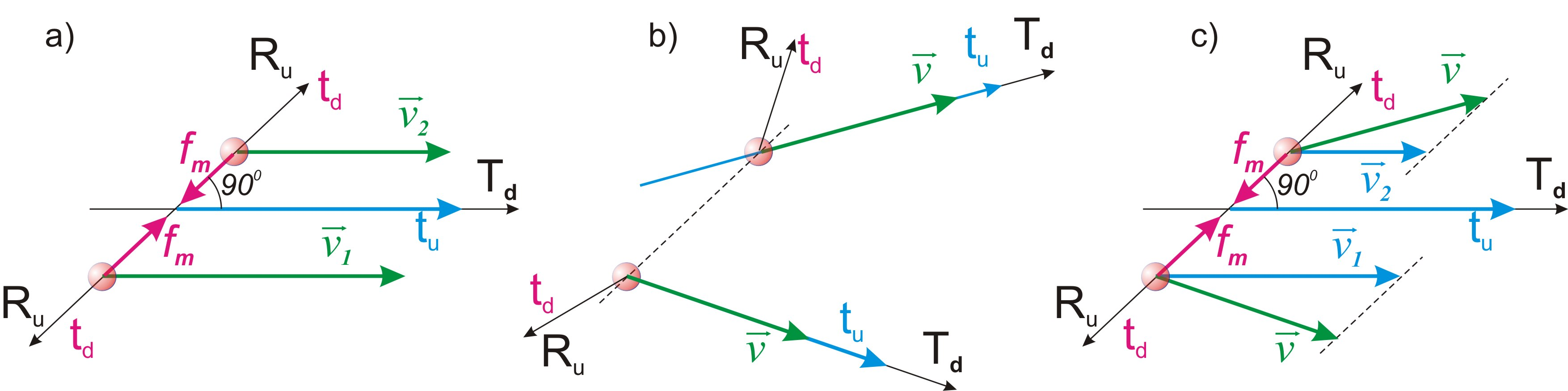Fig. 42.

Since the mobile dimension Td is projected relative to each particle by its motion vector, then with respect to charged particles whose direction of motion is not parallel, the direction of a definite dimension Ru will not coincide with the line connecting them, fig. 42, b).

One of the conditions of the magnetic force is that it, as a consequence of the mutual disturbance of the symmetry by the charges, acts in a straight line connecting them. Therefore, both interacting charges should be considered as a single reference frame, the mobile dimension vector Td relative to which is directed perpendicular to the line connecting them, fig. 42, c).

From this follows the conclusion:

Conclusion (c134):

The magnetic force acting between two like charges is determined by the projections of their motion onto a direction perpendicular to the force vector.

Relative to the proton, the direction of the projection of the definite dimension tu of the inner cycle is reversed (conclusion (c90)). It follows from this that the magnetic force in relation to two protons moving in the same direction will be projected opposite to the gravitational, that is as repulsive force.

The electron moves along the projection of the inner cycle of elementary localization towards the proton. Thus, the direction of its movement along the cycle coincides with the movement along the cycle of the main localization. It follows from this that the magnetic force with respect to two electrons moving in the same direction will be projected by the force of attraction.

Conclusion (c135):

The magnetic force with respect to two protons moving in the same direction acts as a repulsion, and with respect to two electrons moving in the same direction as an attraction.

Let us find the conditions for the projection of the electric interaction force into magnetic cycles.

The inner cycle of elementary localization along the definite dimension tu is projected onto the mobile dimension of the physical space Td. This dimension is indefinite, and in relation to each moving particle is projected by its motion vector. Thus, the speed of the particle in physical space relative to the ideal frame of reference is the only determining condition for the size of this projection.

If a particle moves with a spee v relative to the ideal frame of reference, then its displacement in the state quantum is equal to vdt. This shift in the state quantum corresponds to a complete cycle of size ntvdt.

Conclusion (c136):

The size of the projection of the inner cycle of elementary localization into physical space relative to a particle with a charge is determined by the speed of its movement relative to the ideal frame of reference and is equal to ntvdt.

For simplicity, consider the option of parallel movement of charges.

The definiteness of the charge with respect to the projection of the inner cycle, corresponding to the material definiteness of the particles with respect to the main cycle (definitions (d35) and (d36)), must be determined relative to this size ntvdt. But at the same time, the disturbance of symmetry by charges relative to each other occurs along a definite dimension of the physical space Ru, the size of which is greater than and equal to Rt (conclusion (c38)). Accordingly, the projection of the force of the symmetry restoration cycle into the magnetic cycle should decrease in proportion to the ratio of these sizes in Rt/ntvdt times.

Taking into account formulas (06) and (02):

Rt = ntdr

c = dr/dt

Rt/ntvdt = ntdr/ntvdt = c/v (103)

This decrease in force, projected into the magnetic cycle, occurs with respect to one of the charges moving at speed v. A similar decrease in force in the projection into the magnetic cycle should occur with respect to each of the charges, in accordance with their speed of movement relative to the ideal frame of reference.

Thus, for two particles in a magnetic cycle moving in parallel with velocities v1 and v2 the electrical interaction force is projected proportionally to v1v22 :

fm = fev1v22 (104), where fm – the magnetic force acting between two charges moving in parallel.

It follows from this formula that when particles move in opposite directions, one of the velocity projections becomes negative, and as a result, the force changes direction to the opposite.

Conclusion (c137):

The magnetic force with respect to two protons moving in opposite directions acts as an attraction, and with respect to two electrons moving in opposite directions as a repulsion.

This conclusion is very important for experiments with attraction or repulsion of parallel conductors with electric current. In any inertial frame of reference, the speed of movement of charges is the sum of the speed of movement of the conductors themselves and the speed of movement of electrons relative to the conductors. With regard to the movement of conductors, the magnetic forces of positive charges and negative charges balance each other with their opposite direction. Thus, the resulting magnetic force is determined solely by the speed of the electrons relative to the conductor.

Conclusion (c138):

The magnetic force acting between two conductors with electric current is determined by the speed of the charges relative to the conductors in any inertial frame of reference and does not depend on the speed of the frame of reference or the speed of the conductors.

Let us use the formula (64) obtained by us for the electrical interaction:

fe = q1q2dmvc2nt3dr/πn3q02R2

fm = q1q2dmvv1v2nt3dr/πn3q02R2 (105)

Let us use the formula (65) obtained by us for the electric constant:

Q = dmc2nt3dr/πn3q02

fm = q1q2v1v2Q/с2R2 (106)

This formula is fully consistent with the formula obtained experimentally:

fm = µ0q1q2v1v2/4πR2

µ0 = 4πQ/с2 = 4π•10-7н/А2 (107)

30. Electromagnetic induction

Content

In accordance with the conclusion (c136) made in the previous chapter, the size of the projection of the internal cycle of elementary localization into physical space in relation to the charge is determined by the speed of its movement relative to the ideal frame of reference and is equal to ntvdt.

This size ntvdt is the full size of the projection of the definite dimension tu of the potential part of an elementary localization onto the mobile dimension Td in the state quantum nt and, by the principle of symmetry, is equal to the size of the projection of the definite dimension ru of the material part of this elementary localization.

When a certain force is applied to a charged particle, its speed begins to change. As a result, the size of the projection of the inner cycle onto the mobile dimension Td changes, which is identically reflected in the size of this projection onto the indefinite dimension of the physical space Rd, onto which the definite dimension ru of the material part of the elementary localization is projected.

Thus, when a force f1 acts on a particle with a charge along the mobile dimension Td, this effect is identically projected by the action of the force fd along the indefinite dimension of the physical space Rd, fig. 43.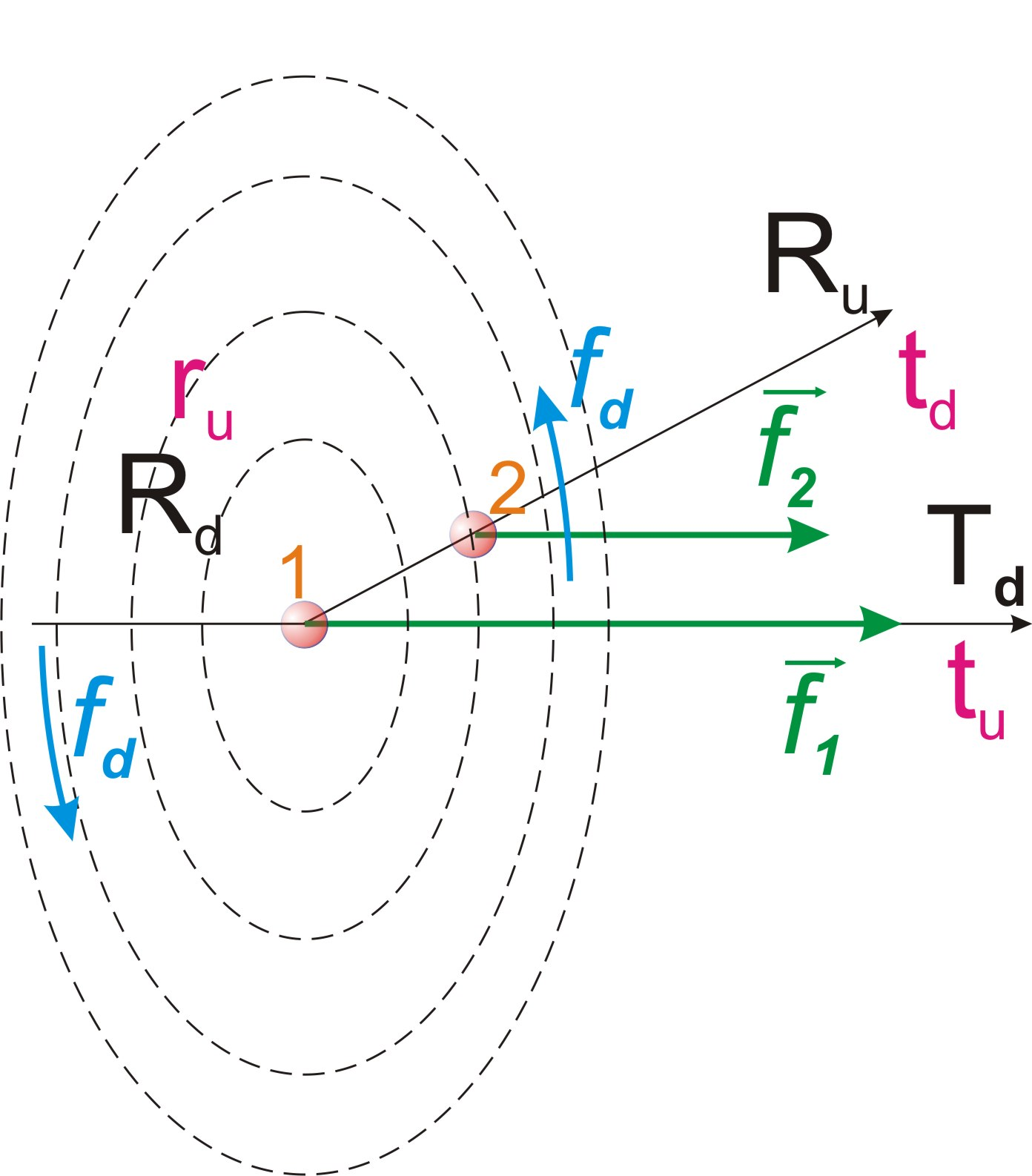Fig. 43.

The force fd is projected perpendicular to the definite dimension of the physical space Ru, and its magnitude depends on the distance to the charge, which is affected by the force f1.

With regard to charged particles, the projections of the cycles of elementary localizations into the physical space are superimposed on each other. It follows from the principle of charge identity that the force fd, acting on one of the charges along the indefinite dimension of the physical space Rd, must act on any particle with the like charge, depending of the projection conditions.

This effect of the force on the second charge, according to the principle of back projection, should be reflected by the force f2 acting on it along the mobile dimension Td.

Conclusion (c139):

Magnetic induction is the projection of the force acting on a charge along the mobile dimension Td onto the indeterminate dimension Rd of the physical space relative to this particle.

It is important to note that there are two options for the projection of the direction of the certain dimension ru of the material part of elementary localization onto the indefinite dimension Rd of the physical space, fig. 44, a) and b).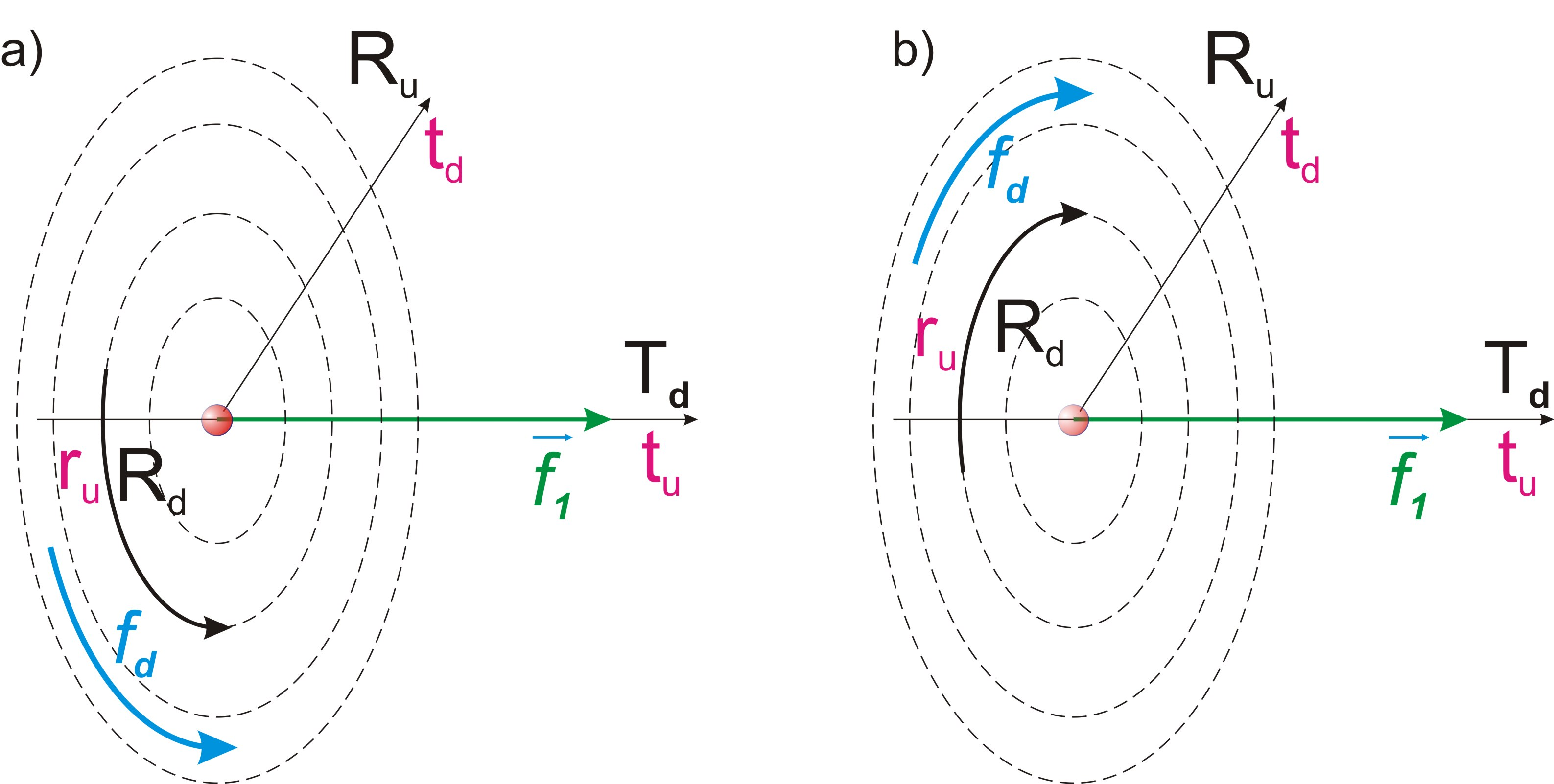Fig. 44.

The fact that only one of the options is implemented, fig. 44, a), is a consequence of the initial act of choosing one of the two dimensions of the potential part of the main localization as the definite (mainstay,Tu), or the one along which the symmetry restoration cycle is shifting

This choice is identically projected by the choice of one of the two directions of the mobile dimension Td as the motion vector of bodies in the physical space. Further, this choice determines the direction of the projection of the definite dimension ru of the material part of elementary localization onto the indefinite dimension of the physical space Rd after the decay of a fundamental particle (neutron).

In the case of our Universe, this choice is reflected by the choice of the right-handed variant of the action of electromagnetic forces. With regard to Limitlessness, both options: right-handed and left-handed are two identical and opposite options.

Conclusion (c140):

The direction of the magnetic induction vector, relative to the direction of the force acting on the charge, the so-called “rule of the gimlet” or right-handedness in physics is a consequence of the initial choice of one of the two dimensions of the potential part of the main localization as the definite dimension along which the symmetry restoration cycle moves.

31. Nuclear forces

Content

When superimposing in the physical space the area of presence of a proton with the area of presence of a neutron, additional conditions of the projection of the internal space of a fundamental particle are imposed on the physical body of the proton, as a projection of the internal cycle of elementary localization.

The direction of the projection of the definite dimension tu of the inner cycle of elementary localization with respect of the proton and neutron is opposite. Accordingly, inside the area of presence of the neutron between the physical bodies of the proton and neutron, a force equal to the force of electrical attraction begins to act, directing them to each other.

In this situation, the physical body of the neutron represents for the proton the boundary of its cycle of symmetry restoration, to which it moves. The physical body of the proton, for its part, similarly represents for the physical body of the neutron the boundary of its cycle, to which it moves.

The approach of the physical bodies of the proton and neutron occurs according to the conditions of the physical space - along the mobile dimension Td. The inner space of the neutron, as a fundamental particle, is indefinite in relation to the physical space, and its conditions, such as the stage of the cycle, do not prevent this approaching.

As a result, the physical bodies of the proton and neutron approach so much that they begin to superimpose in one area of physical space.

Prior to the superimposition of the area of presence of the proton on the area of presence of the neutron, their physical bodies were determined by their sizes of presence which is rt for the neutron and rtp for the proton.

As the physical bodies approach and superimpose, the size of presence of the proton is reduced in relation to the neutron along the mobile measurement Td from the size rtp to the size of the physical body rp, fig. 45.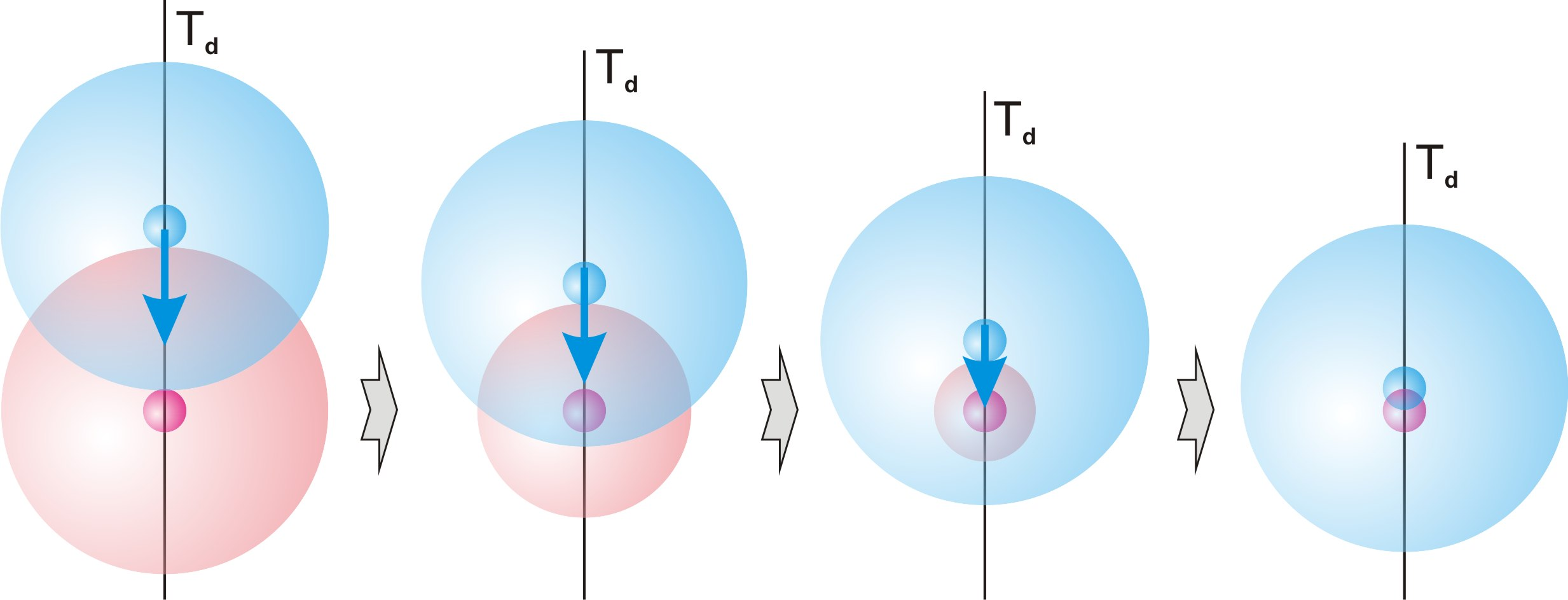Fig. 45.

Accordingly, its definiteness in relation to the physical body of the neutron along the mobile dimension Td increases by the ratio of its size of presence rtp to the size of its physical body rp. In accordance with formula (08) and the found value (89):

rtp/rp ≈ rt/rp = n/nt = 275,0 (108)

This increase in definiteness definition (d35) leads to a proportional increase in the size of the symmetry disturbance of one physical body relative to another, which leads to the proportional increase in the force of their attraction.

Conclusion (c141):

The force of interaction between a proton and a neutron in a deuterium nucleus is in n/nt = 275,0 times greater than the force of the electrical interaction of two unit charges at a similar distance.

Mutual superimposition of the physical bodies of a proton and neutron is possible only until each of them reaches the projection of the center of the inner space of the other.

Their further approaching is impossible, since this requires a reversal of the direction of the projection of the internal definite dimension tu of one of them. Such a reversal of the direction of the projection of the definite dimension tu is possible only as a result of a complete cycle over the indefinite dimension td.

Conclusion (c142):

The superimposition of the internal space of the physical bodies of a neutron and proton cannot occur more than before the mutual achievement of the projection of the center of the internal space of each other.

This process of superimposition of the physical bodies of a neutron and proton does not affect the process of motion of the physical body of the neutron along the internal indefinite dimension td of its localization, caused by the motion of the spot frame of reference associated with the Earth.

The physical body of the neutron moves in the same way as during the decay of a free neutron along the internal indefinite dimension td of its localization with acceleration. The physical body of the proton, coupled with it, moves along with it.

As a result, after a full cycle along the projection of the indefinite dimension td of elementary localization, the physical body of the neutron leaves the projection of its internal space, and the direction of the projection of the definite dimension tu in relation to it changes to the opposite. The direction of the projection of the definite dimension tu in relation to the physical body of the proton is similarly reversed, and it takes the place in the projection of the cycle, vacated by the physical body of the neutron.

Thus, both physical bodies, moving along the projection of the closed indefinite dimension td of elementary localization, turn over relative to the inner and outer boundaries of the projection of its internal space, similarly to the movement along the “Mobius strip”, fig. 46.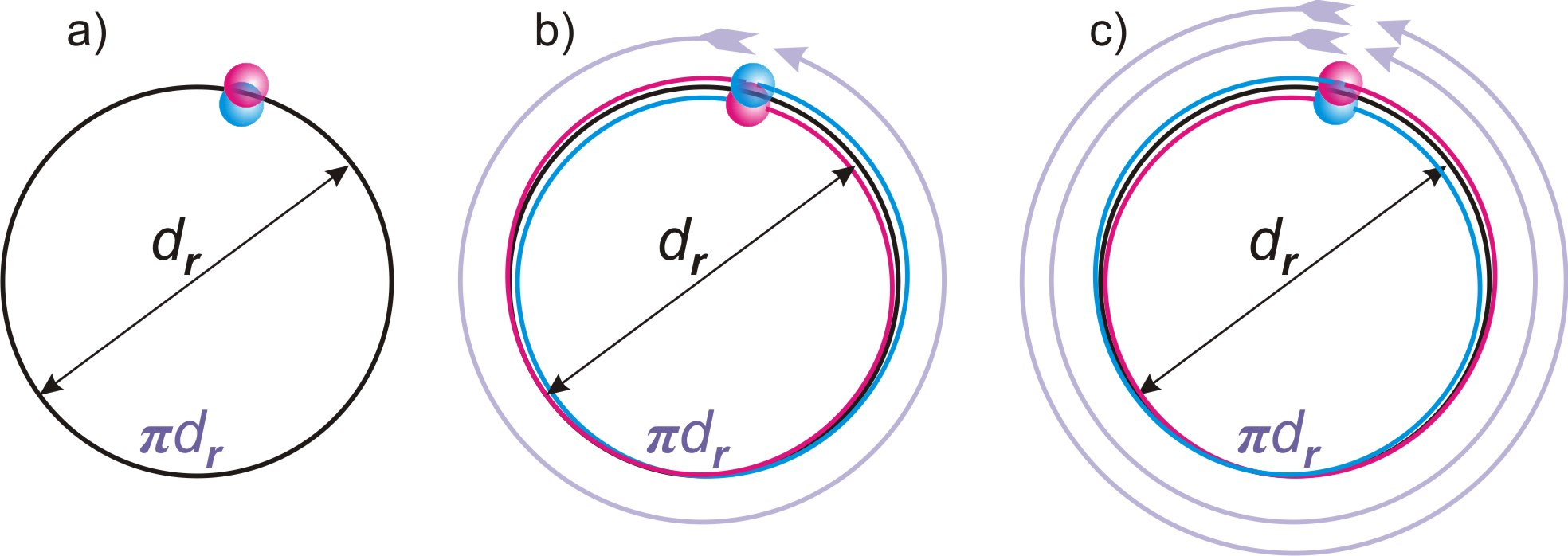Fig. 46.

The position at the beginning of the cycle is shown in fig. 46, a). After a complete cycle along the indefinite dimension td, the physical bodies of the proton and neutron change places relative to the inner and outer space, fig. 46, b). The next full cycle swaps them again, fig. 46, c).

Conclusion (c143):

The physical bodies of the proton and neutron after each full cycle of displacement along the projection of the indefinite dimension td of elementary localization change places, representing in turn a fundamental particle (neutron).

Thus, a stable, non-decaying state of cohesion of the proton and neutron arises.

Conclusion (c144):

A neutron in conjunction with a proton is a dynamically stable state.

The size of presence rt of the neutron remains unchanged, but due to the exit of the attached physical body of the proton beyond its limits, the size of the area of presence of their coupling increases by rp/2, fig. 47.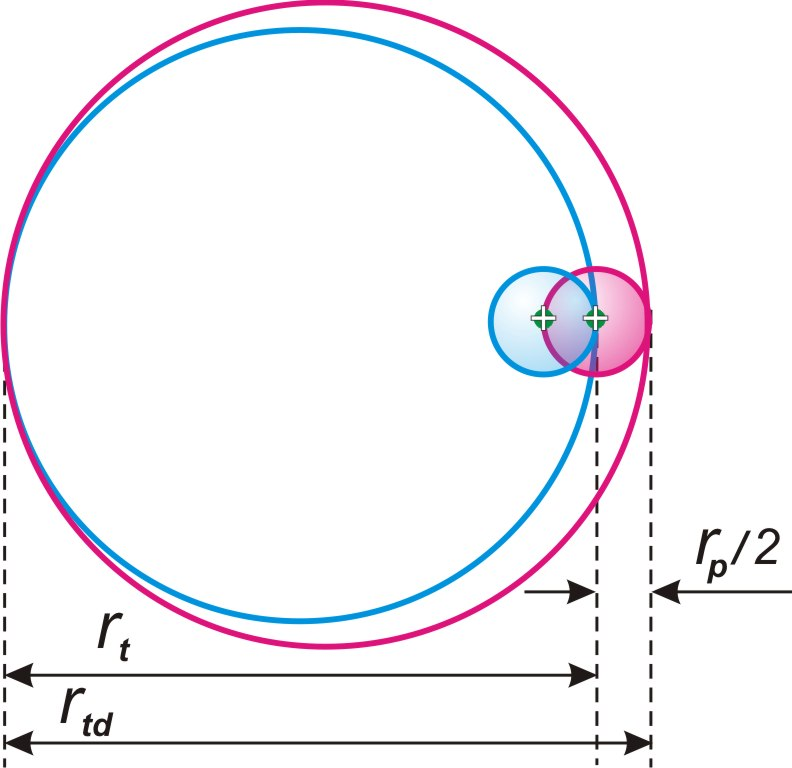Fig. 47.

According to the condition of the connection of both physical bodies, this size of presence will be equally projecting relative to each of them.

rtd = rt + rp/2 (109), where rtd – the size of the area of presence of coupling physical bodys of the proton and neutron.

In accordance with this change in the size of presence, the definiteness of each of them along the mobile dimension Td and, accordingly, the mass will change proportionally.

Let us find the mass of the deuterium nucleus as the sum of two baryons, the mass of each of which is determined by the size of presence of the coupling of their physical bodies, according to the formula (98):

md = 2dmvrt/rtd = 2dmvrt/(rt + rp/2) = 2dmv/(1 + nt/2n) (110) , where md – the mass of the deuterium nucleus.

md = 3,34375•10-27 kg (111)

Experimentally known mass of deuterium nucleus - 3,343 583 20(17)•10-27 kg.

Discrepancy of only 0,005%.

Sufficient accuracy of coincidence of the calculated and experimental masses of the deuterium nucleus leaves no doubt about the correctness of the theory.

Let us find the value of the force of interaction between a proton and a neutron in a deuterium nucleus at a distance rp, using the formula (63)we have already found for the electrical interaction of two charges:

fe0 = dmvc2nt3dr/πn3R2

fnp= (n/nt)fe0 = (n/nt)dmvc2nt3dr/πn3rp2 = dmvc2nt2dr/πn2rp2 (112), where fnp – the force of nuclear interaction in the nucleus of deuterium.

Taking into account formula (09):

rp = nt2dr/n2

fnp = dmvc2nt2dr/πn2rp2 = dmvc2n2/nt2drπ (113)

We calculate the exact value of this force using the tabular and found values (c125),(88),(90):

dmv = 1,674927351(74)•10-27 kg

c = 299 792 458 m/s

n = 2128

nt = 1,23690•1036

dr = 1,0025089•10-10 m

fnp = dmvc2n2/nt2drπ = 36 175,0 N (114)

32. Quarks

Content

Modern physics considers baryons (protons and neutrons) as consisting of three subatomic particles — quarks. In experiments, quarks manifest themselves exclusively in a very small region and a very short period of time, and it is impossible to separate them into independent existence.

Unlike the classical view of physics on the dimensions of space, solely as structural characteristics that are possible for measurement, this theory considers the dimensions of space as parts of the localization of the Universe, identical to any other parts of it, which are singled out material objects. This means that within the state quantum of the process (definition (d30)), in accordance with the conclusion (c68), within certain limits of the experiment, it is possible to fix the projection of an elementary particle on any of the dimensions of the physical space, as an independent physical object.

Conclusion (c145):

Within the quantum of the state of the process, there is a possibility of dividing the process of measuring an elementary particle into three separate stages along each of the dimensions of the physical space, perceived as independent material particles.

Alike process occurs during the experimental detection of three quarks that make up a baryon.

The indefinite and the definite properties of dimensions define two types of quarks.

The neutron, as an identical part of the material part of the main localization, is defined in the physical space by its three dimensions (глава 7, conclusion (c32)): one definite dimension Ru and two indefinite dimensions Td and Rd (udd).

The proton, in accordance with the conclusion (c99), is determined in physical space by the projections of the definite tu and indefinite td dimensions of the potential part of the elementary localization and by the projection of a definite dimension ru of its material part. Accordingly, the proton is defined in physical space by two definite and one indefinite dimensions (uud).

Conclusion (c146):

Quarks are a projection of baryon properties onto definite and indefinite dimensions.

Conclusion (c147):

The quark (u) reflects the properties of the definite dimension of localization.

The quark (d) reflects the properties of the indefinite dimension of localization.

--------------------
﻿
 ««ПУБЛИКАЦИИ««

 Ваше имя:     Н А П И Ш И Т Е     В А Ш     К О М М Е Н Т А Р И Й : Если Вы не робот, Напишите название месяца следующего после осени без мягкого знака.:

 К О М М Е Н Т А Р И И

 Страница 1 из 2 На страницу 1, 2  След.:
А что такое Атма, Манас? Это Лучи, где у Вас определен Луч?:
 Евгений писал(а): «А что такое Атма, Манас? Это Лучи, где у Вас определен Луч?»

Уважаемый, Евгений, тема посвящена устройству физического мира, и его физическим законам.
Надеюсь, Вы понимаете разницу между физическим телом и Манасом? А разговоры о "Лучах" ведите где-нибудь на религиозных форумах.:
Уважаемый Андрей! Вот здесь требуется пояснение.
 Цитата: «Вывод (в31):Направление измерения потенциального времени в частной системе отсчета представляет собой суперпозицию всех возможных направлений, перпендикулярных вектору физического времени.Эта ситуация тождественно проецируется на внутренние измерения Ru и Rd выбираемой части. Эти измерения выбираемой части тождественны измерениям локализации, но не совпадают с ними. Таким образом, пространство частной системы отсчета представляет собой суперпозицию всех возможных направлений трех взаимо-перпендикулярных измерений Ru, Rd и td, с условием их перпендикулярности вектору физического времени tu, рис. 8»

Если тождественны но не совпадают, то это несовпадение желательно показать на рисунке.
Движение по физическому времени для циклов локализаций ( относительно фунд. принципа выбора) и для частной системы отсчета - общее?:
Гость писал(а): «Уважаемый Андрей! Вот здесь требуется пояснение.
 Цитата: «Вывод (в31):Направление измерения потенциального времени в частной системе отсчета представляет собой суперпозицию всех возможных направлений, перпендикулярных вектору физического времени.Эта ситуация тождественно проецируется на внутренние измерения Ru и Rd выбираемой части. Эти измерения выбираемой части тождественны измерениям локализации, но не совпадают с ними. Таким образом, пространство частной системы отсчета представляет собой суперпозицию всех возможных направлений трех взаимо-перпендикулярных измерений Ru, Rd и td, с условием их перпендикулярности вектору физического времени tu, рис. 8»

Если тождественны но не совпадают, то это несовпадение желательно показать на рисунке. »

Тождественность означает, что внутреннее пространство материальной части и внутреннее пространство локализации формируются по одинаковому принципу: с одним определенным измерением, и одним неопределенным.
Несовпадение означает их независимость друг от друга, таким образом, весь процесс движения материальной части в локализации определяется четырьмя независимыми измерениями.
Это невозможно показать на рисунке, только в некоей условности, что я и сделал: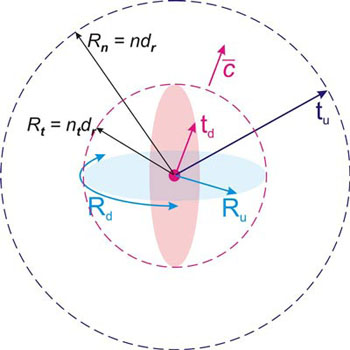Наше представление сформировано в трехмерном пространстве, в котором измерение физического времени никак не отражается.
Пространство локализации Вселенной, по которому движется материальная часть, и физическое пространство это разные пространства, каждое со своими условиями. Эти пространства пересекаются только в отношении измерения потенциального времени. Поэтому, если изображать трехмерное пространство, то измерение физического времени в нем не присутствует, и может быть только указано неким условным вектором, предполагая при этом, что он перпендикулярен измерениям физического пространства. Но при этом необходимо еще учесть, что измерения физического пространства находятся в суперпозиции всех возможных направлений. Таким образом, какое бы Вы не выбрали направление, какую бы не провели черту, измерение физического времени всегда будет перпендикулярно ей.

 Гость писал(а): «Движение по физическому времени для циклов локализаций ( относительно фунд. принципа выбора) и для частной системы отсчета - общее?»

Необходимо различать время, как последовательность квантов состояния материальной части локализации Вселенной, и измерение внутреннего пространства локализации Вселенной, которое определяет собой эта последовательность.
Последовательность квантов состояния Вселенной едина для всех систем отсчета. Это означает, что количество пройденных квантов состояния всегда одинаково и не зависит от системы отсчета.
Все системы отсчета синхронно переходят из одного кванта состояния в следующее.
Другое дело, это пространственное направление этой последовательности квантов состояния в относительности конкретной частицы, физического тела или системы отсчета. И вот это относительное направление различается.:
 Цитата: «Обозначим пространственный размер кванта протяженности как dr, а в единицах времени, как dt»

Значит dt, это в единицах времени, пусть это будут секунды.
А протяженность dr, тогда в каких единицах?:
Гость писал(а): «
 Цитата: «Обозначим пространственный размер кванта протяженности как dr, а в единицах времени, как dt»

Значит dt, это в единицах времени, пусть это будут секунды.
А протяженность dr, тогда в каких единицах?»

Единицы измерений зависят от системы единиц. Я бы создал новую систему единиц, в которой этот размер кванта протяженности был бы равен единице, как ее назвать, это уже второй вопрос. Но, поскольку все экспериментальные результаты записываются в уже принятых международных системах единиц, то приходится пользоваться именно ими.

Мне удобнее система единиц СИ (SI). В этой системе единица времени – секунда, единица длины – метр.

Поэтому, размер кванта протяженности в единицах времени обозначается dt и измеряется секундами, а в единицах длины обозначается как dr и измеряется метрами.

Вычисленные значения:
dr = 1,0025136•10^-10 м
dt = 3,344026•10^-19 с:
ЭТОТ ВОПРОС ИЗ ГЛАВЫ 12. Движ. с релятив. скоростями.

 Цитата: «"rv = r0(1-v2/c2) (24)Вывод (в55):Размер движущейся элементарной частицы сокращается относительно покоящейся пропорционально (1-v2/c2).Радиальный размер элементарной частицы отражается одинаково в любом направлении физического пространства, соответственно уменьшение размера происходит по всем измерениям физического пространства"»

Вывод формулы мы делали для вычисления сокращения размера в проекции на определённое измерение потенциального времени.
Вопрос: На каком основании мы распространяем теперь это сокращение размера по всем измерениям физического пространства?:
 Цитата: «Рис. 1.Но поскольку потенциальные внутренние части первичной части тождественны, это означает, что каждая из потенциальных внутренних частей первичной части должна содержать в себе всю вторую первичную часть целиком, рис. 1, b). С другой стороны, каждая из внутренних частей первичной части должна содержать в себе и всю первичную часть, частью которой она является.Этими условиями определяется двумерное пространство двух независимых протяженностей. Любая часть одной протяженности одновременно является частью и другой протяженности и отражает в себе обе протяженности со всеми их частями, рис. 1, c).К этому нужно добавить условие замкнутости каждой из протяженностей и симметрию относительно любой из частей."»

Получается, что при первичном Акте деления Беспредельности на две части, вынужденным условием такого деления должно стать наличие протяженности. Проще говоря, должно стать наличие некоего пространственного объема, который в самом упрощённом варианте, можно обозначить всего лишь двумя измерениями. Т е из Беспредельности как некоего ничто, или нечто., возник некий объем. Можно представить и по другому: первоначальная Беспредельность ввиде некоей безграничной вширь и внутрь плоскости. При первичном Акте деления эта плосость поворачивается в, тождественный самой себе, перпендикукляр.

Но, вот условие замкнутости, которое в последнем абзаце, тут наверное не подходит, или это вводит сам Наблюдатель для последующих своих целей.:
Там где я писал объём это не физический объём, а пространство для взаимодействий, вопрос только в том, в нашем понимании, это пространство должно быть ограниченным или нет.:
 Гость писал(а): «Вывод формулы мы делали для вычисления сокращения размера в проекции на определённое измерение потенциального времени. Вопрос: На каком основании мы распространяем теперь это сокращение размера по всем измерениям физического пространства?»

На основании:
 Глава 9 писал(а): «Этот размер rt базовой элементарной частицы инвариантен относительно физического пространства и определяет ее размер по измерению потенциального времени и по определенному измерению выбираемой части локализации.Условие полной замкнутости внутреннего пространства базовой элементарной частицы проецируется в физическое пространство его однородностью.Вывод (в39):Базовая элементарная частица в физическом пространстве представляет собой однородный шар диаметра rt, с полностью замкнутым внутренним пространством. »

Дополнительно поясняю, что элементарная частица полностью тождественна основной локализации, и сама представляет собой локализацию. Локализация, как тождественная часть Беспредельности обладает принципом симметрии. От любой точки внутри локализации расстояние в любую сторону до границы всегда одинаково.

Скорость движения по неопределенному измерению потенциального времени не может изменить принципа инвариантности физического пространства.

Не свойства пространства определяют размеры частиц, а частицы определяют пространство.

И еще очень важно: размер предполагает процесс измерения. Любое измерение в физическом пространстве во всех случаях может производиться только по измерению потенциального времени. То есть, этот размер является первичным условием для формирования трехмерного пространства. Два других измерения лишь создают принцип трехмерности, как суперпозицию всех возможных направлений в условиях этих трех измерений, и определяют магнитные процессы.:
 Гость писал(а): «Получается, что при первичном Акте деления Беспредельности на две части, вынужденным условием такого деления должно стать наличие протяженности. Проще говоря, должно стать наличие некоего пространственного объема, который в самом упрощённом варианте, можно обозначить всего лишь двумя измерениями. »

Что значит «упрощенный вариант»? Смотрим по тексту:
 Глава 2 писал(а): «Первичный акт выделения части Беспредельности накладывает на нее первичное условие потенциальной делимости на тождественные части.Вывод (в5):Первичным ограничивающим актом Беспредельность разделяется на две тождественные части.Однако речь идет о выделении одной из частей в первичном акте. Это означает, что обе тождественные части не должны совпадать друг с другом.Этот принцип различия представляет собой первичный принцип протяженности или пространства. Наличие первичного деления в совокупности с неограниченностью Беспредельности и принципом тождественности предполагает возможность дальнейшего деления Беспредельности на части.Вывод (в6):Первичное деление Беспредельности на две части редуцирует ее в состояние субстанции, обладающей потенциальной протяженностью и делимостью. »

Наличие двух несовпадающих частей уже само по себе является пространственным принципом или принципом протяженности.
Из принципа тождественности (Теорема (т2):) следует, что если Беспредельность разделилась на две части, то есть обладает свойством делимости, то и каждая из ее частей должна обладать свойством делимости. Таким образом можно сколько угодно раз делить на два любую часть, полученную предыдущим делением.
Далее:
 Глава 2 писал(а): «Тождественность части целому, необходимо накладывает условие, что каждая из двух первичных частей должна полностью содержать в себе другую, рис. 1, a). »

Подчеркиваю, что не «можно обозначить двумя измерениями», а именно принцип делимости на два в совокупности с тождественностью частей и целого, определяют два измерения. Оно, двумерное пространство, вот так ВОЗНИКАЕТ там, где до этого не было ничего.
Мы привыкли к своему существованию в пространстве, и не задумываемся, включая физиков, а как собственно оно, пространство вот таким получилось, где в каждой точке одного измерения проецируется всё другое измерение целиком (в случае трехмерного пространства – два измерения целиком).

 Гость писал(а): «Т е из Беспредельности как некоего ничто, или нечто., возник некий объем. »

Нужно учесть, что это первоначальное деление на две части сопряжено с первичным актом выбора одной из частей. Так что в первом акте выбора мы имеем лишь одну двумерную часть, выбранную внутри другой двумерной части. Насколько это соответствует понятию «объем»? Я бы сказал, что на этом этапе преждевременно вводить подобное понятие. В любом случае это множество из 4 частей, о чем говорится в следующей главе.

 Гость писал(а): «Можно представить и по другому: первоначальная Беспредельность в виде некоей безграничной вширь и внутрь плоскости. При первичном Акте деления эта плоскость поворачивается в, тождественный самой себе, перпендикуляр. »

До первичного акта деления, Беспредельность представляет собой абсолютный ноль, и ее нельзя каким бы то образом представлять. И что значит «поворачивается»? Плоскость может повернуться только в трехмерном пространстве, которого на этом этапе нет, как нет никакой плоскости ни до первичного акта, ни в первых шагах выбора – первых порядках локализаций. Плоскость определяется рядом условий, которые должны сформироваться и формируются только с усложнением порядка локализации.

 Гость писал(а): «Но, вот условие замкнутости, которое в последнем абзаце, тут наверное не подходит, или это вводит сам Наблюдатель для последующих своих целей.»

Возвращаемся в самое начало:
 Глава 1 писал(а): «Любое объективное бытие невозможно без определенного набора ограничивающих условий – физических законов этого объективного бытия. Поскольку Беспредельность не имеет ограничений, таковой набор ограничений может существовать только в своей собственной относительности.Вывод (в1):Существование объективного бытия в Беспредельности возможно только в собственной внутренней относительности этого бытияТаким образом, любое объективное бытие накладывает на Беспредельность набор ограничивающих условий в своей собственной внутренней относительности. Назовем это состоянием Беспредельности.Определение (о2):Состоянием Беспредельности называется Беспредельность с наложенными на нее ограничивающими условиями в собственной внутренней относительности этих условий. »

Любое условие, наложенное на Беспредельность, ограничивает ее в собственной внутренней относительности этого условия, или, по-другому, в системе отсчета, связанной с этим условием.
Принцип тождественности превращает принцип ограничения в принцип полной замкнутости по каждому из измерений.
Принцип симметрии превращает эту замкнутую двумерную структуру локализации в структуру, не имеющую выделенного центра.:
Выбираемая часть локализации много меньше её самой, а количества частей двумерной структуры времени в выбираемой части уже n^2 и согласно принципу Ч.В. там тоже получаеся n^2 частей.Количество частей времени определяет количество выбранных частей в выбираемой части локализации? Как я понял, потом, из этого, получается количество баз. эл. частиц. в выбираемой части локализации.:
Уважаемый Андрей! Зайдите на форум, мне хоть благодарность поставте. Видите как мне приходится "прыгать" из начала в конец, а из конца в начало. Изложение у Вас идёт, да это как бы и видно, с учётом того, что Вы держите это всё у себя в голове как единое цело, в течение длительного времени. Поэтому, человеку с улицы или со своими заботами вникнуть в это не легко. Честно говоря, временами возникает желание вникать дальше бросить. Например большая повторяемость слова "относительно", оно сразу же приводит к мобилизации воображения, даже там, где это не нужно. Сильно дезориентирует, повторяющаяся из предложения в предложение фраза "ограничивающие условия". А тем более уходящие "вглубь" ограничения.:
 Гость писал(а): «Уважаемый Андрей! Зайдите на форум, мне хоть благодарность поставьте. »

Если «гость» это Вы, Яков, то лучше было бы при заполнении формы комментария заполнить поле: «имя», тогда бы мне не пришлось догадываться, кто это задает вопросы.
На форум я захожу изредка, но в моей теме всё зависло, и отвечать некому. В Вашу психологическую тему, в которую Вы меня приглашали, я не только заглянул, как обещал, но и написал довольно большой пост со своим мнением, причем уже довольно давно. Но, такое ощущение, что Вы это не заметили.
Что касается темы с электроном, то те рассуждения, что там идут, никак не пересекаются с моими взглядами и моей теорией, так что мне просто некуда там вставлять свое мнение.
По поводу благодарности, Яков, я уже говорил Вам, что Вы помогли мне своими вопросами увидеть некоторые места в моем тексте, которые я недостаточно или некорректно объяснил. Вот и с вопросом, касающимся фразы: «К этому нужно добавить условие замкнутости каждой из протяженностей…» Вам большое спасибо, так как эта фраза действительно повисла без объяснения и ссылок. Я полностью переписал это место.
Но что касается «форумных благодарностей» или точнее, «кнопочных благодарностей», то я не воспринимаю эту современную интернет-культуру за культуру, как таковую, и не имею желания в нее играть. Я никогда не ставлю смайлики, так как считаю, что писать нужно так, чтобы тебя понимали без этих убогих костылей, а благодарность нужно выражать открыто в словах, а не в нажатии кнопки. Я уже более 15 лет активно в интернете и на форумах, и на моих глазах все эти кнопки появились и внедрялись. Я изначально был категорически против всех этих «лайков», или, как их называли вначале, каким-то убогим словом – «спасибки». А теперь это вообще коммерческий акт: кто больше получит «лайков», тот больше заработает.

 Гость писал(а): «Видите как мне приходится "прыгать" из начала в конец, а из конца в начало. Изложение у Вас идёт, да это как бы и видно, с учётом того, что Вы держите это всё у себя в голове как единое цело, в течение длительного времени. Поэтому, человеку с улицы или со своими заботами вникнуть в это нелегко. »

Это естественно и по другому быть не может.
 Гость писал(а): « Честно говоря, временами возникает желание вникать дальше бросить. Например большая повторяемость слова "относительно", оно сразу же приводит к мобилизации воображения, даже там, где это не нужно. »

Любое утверждение физики должно быть конкретным. Если закон физики утверждает различные следствия для одного и того же процесса в отношении различных систем отсчета, или в отношении разных составляющих этого процесса, то без слова «относительно» всё утверждение, формула или вывод теряют свой смысл.
 Гость писал(а): «Сильно дезориентирует, повторяющаяся из предложения в предложение фраза "ограничивающие условия". А тем более уходящие "вглубь" ограничения.»

На счет «вглубь» не понял, так как не применял такого термина.
Что касается «ограничивающих условий», то это физический принцип, такой же как, например, масса, даже много важнее, вернее первичнее, или в математике – число «пи». Вы же не будете выбрасывать из формул число «пи», только потому, что оно часто повторяется.
 Гость писал(а): «Выбираемая часть локализации много меньше её самой, а количества частей двумерной структуры времени в выбираемой части уже n^2 и согласно принципу Ч.В. там тоже получаеся n^2 частей.Количество частей времени определяет количество выбранных частей в выбираемой части локализации? Как я понял, потом, из этого, получается количество баз. эл. частиц. в выбираемой части локализации.»

Выбираемая (материальная) часть локализации и сама локализация, как целое, полностью тождественны. Соответственно, если локализация разделена на некое количество частей, то на точно такое же количество частей должна быть разделена материальная часть.
Таким образом и получается, что количество частей двумерной структуры времени определяет количество элементарных частей, на которые разделена материальная часть.Страница 1 из 2 На страницу 1, 2  След.Сейчас посетителей в разделе : 1 .ya-page_js_yes .ya-site-form_inited_no { display: none; }